Rese rch 1 . Solutions & Ideas .
Share this Site ' D N A ' if you like it .

' Assuming one Infinity, one Time is just a little grain of Sugar . '

Everything can be slighter by production without poisoning and new exploiting
with a strong recommendation to recycle and to clean from industrial toxics ,
and to take care for each other Living ! Stop ( y ) our war and helping on the way ?

• 1. Photon Size and Weight
• 1.2 Viscosity of Light and Photons per Cubical Metre
• 1.3 New Photon Modell and its Prove
• 1.4 ' Golden Ratio plus 1 ' – ( Division Delta of Frequency ) per ( Division Delta of Diameter )
• 1.5 Photon Shell Mass and Photon Core Mass
• 1.6 Reconstruction Research : An antique Way to Calculate Light Speed ... ( The ' Antique Algorithm ' )

• 1.2.1 Light Particles per cubical metre and ' Ratio ' – Approach 1
1.2.1.1 Light Particles per cubical metre and ' Ratio ' – Approach 1 . 1
• 1.2.2 Light Particles per cubical metre and ' Ratio ' – Approach 2
• 1.2.3 Light Particles per cubical metre and ' Ratio ' – Approach 3
• 1.2.4 Light Particles per cubical metre and ' Ratio ' – Approach 4

• 1. Photon Size and Weight' The Nature of a Photon understood as an average electromagnetic Particle –
Antimatter Shells orbiting a Core consisting of Matter . 2020 / 2021 .

We have found that the possible Superluminal Motion of Antimatter
might to be for the benefit of Intergalactic Space Journeys
by enabling us to move with c . Luminal speeds might even allow
a standing still of time and to stop the process of aging hence ...
The speed of the Photon Shell is calculated by the ' Wavelength Approach ' .
And for cosmic radiation its motion should to be about 67 times Light Speed c ... '

Understanding a Photon as an ' electromagnetic Particle Gemini System ' .
Whereas a shell of negative mass spins around a core of positive mass
and the strength of electromagnetic attraction stucks both the components
together , while the speed our universe expands with counteracts the pull
so that adding energy grows the radius and frequency of spins .
Reaching a certain level of Energy , the motion of the core is not influenced
by the shell ' s spinning movement any longer , resulting a movement of only
forward of the core , because its force by moving straight forward is greater
than acceleration due to electromagnetic attraction between the shell and the core .
And since the ratio of positive and negative mass is ' close to ' 1 we might
speak about a ' spinning gemini satellites system ' as a ( twin ) particle .
This knowledge might to be for a large benefit of Quantum Computers Technology
and the progress and advanced comprehension of Health and Medical Care and Treatments ...

We have done an experiment during the weekend a few weeks ago
and it seems to be a further proof for the electromagnetic particle
consisting of both , positive ( matter ) and negative mass ( antimatter ) :
We hold a magnet very close to our ( right ) eye with the other eye closed ,
and we hold the magnet very close to the sun visible in our pupil ;
Now , we focus on the little specular highlight of our sun on the magnet :
The sun appears dark , since the photon particles are attracted
of the strong magnetical force and redirected from their natural source ( sun ) .
Also , light is broken down to all of the spectral colours at some differing points
which can be seen best with a simple glow or halogen lamp and a magnet .
If we let sunlight shine through a very tiny – but massive – aperture ,
spectral colours appear too , because different masses of light of varying colours
are attracted by the mass of the aperture and light is separated to the spectral colours hence ...

Important Note :
Do not focus your view on the light source directly , because that might damage the retina !
Watching the reflected , indirect or redirected light might not do harm to your eye ...

Watching a dark sun requires a bit of patience and a few trials for fine adjustments of the magnet ...' Shell Super Quantums consisting of antimatter – the matter of a photon ' s core is spinning with ... '

This new Photon Modell understood as Super - or Sub Quantums spinning around a core
has become more likely during these days ...
Each movement of a medium or object is circular :
From waves at the sea shore
( sometimes broken down to half of a circle
because of gravitational force and inertia )
over atomes with electrons orbiting a core
to satellites , planets , stars , galaxies and even more , like clouds shaped by air movement.

And actually we will be able to explain each characteristic of a
' lightwave ' by a photon shell spinning ( circular ) around a core ...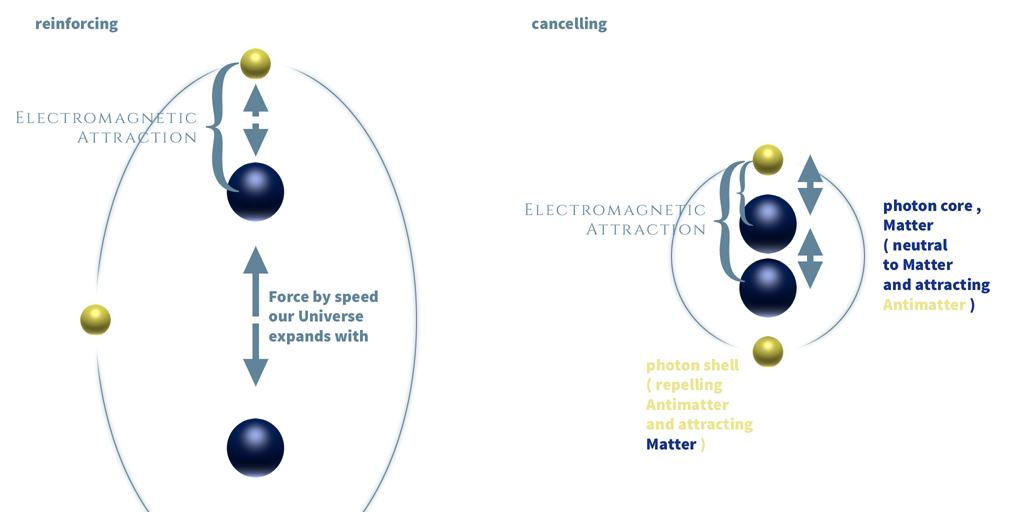' Reinforcing and Cancelling of the Photon Spin perceived as a Lightwave ... '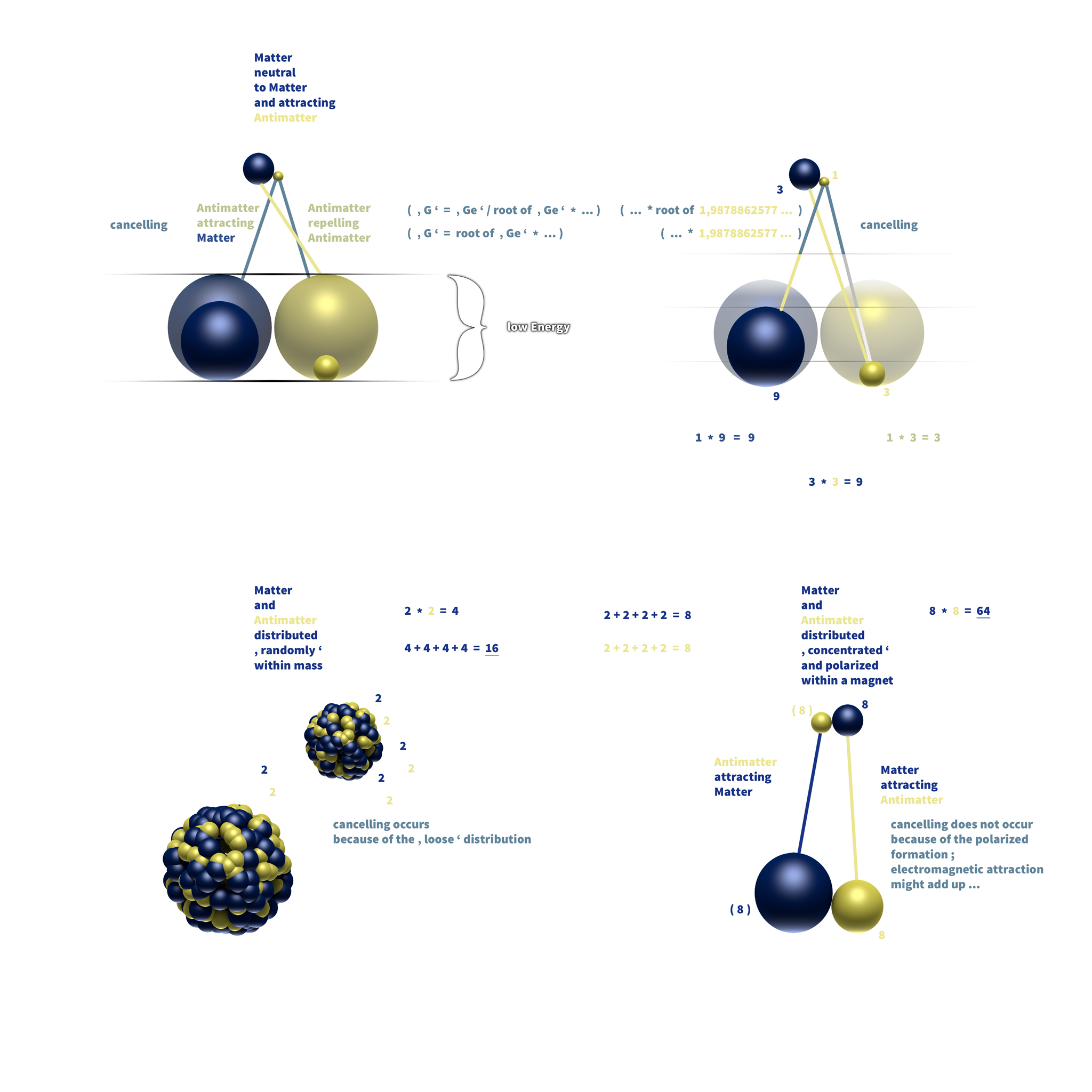' The Principle of Electromagnetic Attraction acting as Gravitational Force . 2023 '

Summed up we will see if mass contains no Energy , matter and antimatter
are contained at an ( almost ) fifty fifty balance , meaning a mass of zero .
So this could explain , why matter can be accelerated up to light speed .
As enriching the fifty fifty proportion of matter and antimatter with Energy ,
we will recognize a movement , while the proportion of matter increases in
parallel to the acceleration ( from zero movement with ' zero ' mass up to
Light Speed with a tiny surplus of matter , meaning to own ' a bit of ' positive mass ) .

Adding energy to electromagnetic particles increases the surplus of matter ,
while the total amount of matter and antimatter shrinks ,
so energetic light particles might to be attracted slightly weaker by gravitational
force than light particles owning a very low level of energy ...

It seems to be not a final proof for matter
being neutral to matter , while the circumstance
of matter acting neutral to matter is still very
likely at times , may be we are going to see more
facts about the strongly assumed mutual neutrality
of matter in the future .
This understanding might also be in harmony with
our recognition of time to move faster as we can
perceive it . Matter might play along this game
while antimatter counteracts and hence time is
passing at this certain noticeable speed to all
of us within our known existence .
And time moves forward , because matter is in a
bit of surplus within all of our Universe except
for black holes , where the surplus of matter might
to be infinitely small and that is why time comes to
a standstill at the event horizon ...

Anyway , if matter would attract matter , we might see a
force of attraction stronger than the power of rejection
within a magnetical force field because matter attracting
antimatter would add up with matter attracting matter ...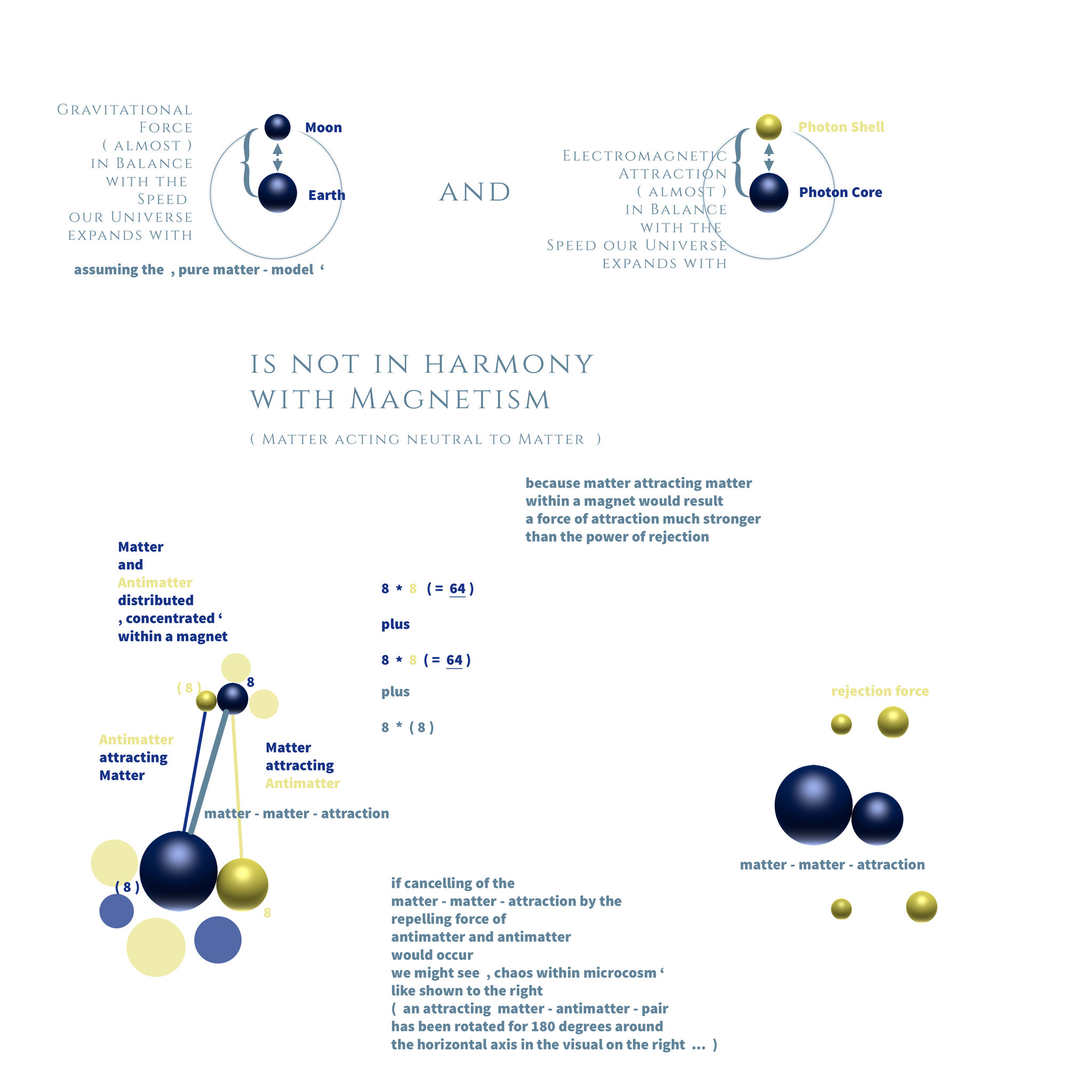' The ( false ) assumption of Matter attracting Matter . 2023 '

Photon Size by Max Planck :

We are happy to say that we were able to calculate
the Size of a Photon by the Planck ' s Quantum of Action   ( h )   :

We have found to a new , elementary Quantum ,
referred to as   (   I . 1   )   and the value is going to be
1,753287443505388042612046671084e-27   .
This value is calculated by the delta Energy
of a photon at one spin per second and the
maximum radiation ( emitted directly from the
Super Massive Center ) ...

The Energy Value of a photon at one spin per second is
6,62607015e-34 and the delta to
1,753287443505388042612046671084e-27 is
3,7792263753126213313061036080214e-7
while this value should pose the Energy of the
3,7792263753126213313061036080214e-7   |   per h
570.357.133.226.641.334.502.941.175,17324   |   * 1,9878862577579345578272656386017
1.133.805.107.155.451.756.702.297.926,7205
and the delta to the maximum radiation calculated by the ' Antique Approach '
( 1.134.869.703.006.486.329.734.652.378,7289 spins per second )
is
1,0009389584191462019024295701562 ;

Multiplying   (   I . 1   )   with   h   ,
with   { third root of ( frequency squared ) }   squared ,
extracting the square root ,
and multiplying with   pi   , and with   root of 2
results the diameter of an electromagnetic particle .

We are going to see an example , using red light
with a frequency of   428274940000000   spins :
1,753287443505388042612046671084e-27   *   6,62607015e-34   =
= 1,1617405593780863073318610477677e-60   ;
{   3rd root of   ( 428274940000000 square )   }   square   =
32282231721683086762,643961820567   ;
1,1617405593780863073318610477677e-60   *   32282231721683086762,643961820567   =
3,7503577938321111420562121865286e-41   |   the square root is
6,1240164874305418072086222012103e-21   |   times pi   ,   times root of 2   =
2,7208288365005683163120626008398e-20   ,
what is the same value like the first solution calculated by the   ' Wavelength Approach '   ...

h   *   (   I . 1   )   =   1,1617405593780863073318610477677e-60
1,1617405593780863073318610477677e-60   *   428274940000000 square   *   third root of 428274940000000   =
=   1,1617405593780863073318610477677e-60   *   1,3825670853669920682286136990066e+34   =
=   1,6061842591319797694374557508128e-26   |   the reciprocal value is
62259357499893805366847318,893388   |   times   428274940000000   =
2,6664122597705569499858213488227e+40   |   the reciprocal value is
3,7503577938321111420562121865286e-41   |   the square root results
6,1240164874305418072086222012102e-21   |   multiplied with pi and times root of 2   =
2,7208288365005683163120626008398e-20   ;

Today ( 2022 - 2 - 22 ) , in the late afternoon ,
we were able to see a new proportion of
( 1 kilogramm times speed squared )
per
1 metre squared
for the equivalent ' Energy ( E ) = r squared '
which might to be described with a ratio - value of
5,2224305027273181459851848605027
for red light with a wavelength of 700 nanometres ;
because the Energy a photon holds is defined by mass , and
since kilogramms and meters do not have much to do with each other
we have to look for a ratio of mass per dimension of size ...

If we modify Planck ' s Quantum of Action ' h ' to
3,7773432898853245299273804905425e-34
we are going to see an exact ratio of
( mass times speed squared ) per distance squared
which is defined by
1,7541614943345960207779019426033 ...

Multiplying the value of
3,7773432898853245299273804905425e-34
with ( frequency exponent two ) times ( third root of frequency )
we are going to see the diameter exactly ,
an example by using the frequency of red light :
3,7773432898853245299273804905425e-34     |   * frequency exponent two * third root of frequency
3,7773432898853245299273804905425e-34 * 428274940000000 square * third root of 428274940000000
3,7773432898853245299273804905425e-34 * 1,3825670853669920682286136990066e+34 =
5,2224305027273181459851848605028 ;

E = h * f
E = r squared
( kilogramm times speed squared ) per metre squared is
5,2224305027273181459851848605027
for the equivalent E = r squared
and for red light
and E = mass * c squared

We are going to multiply Max Planck ' s Quantum of Action h
with
5,2224305027273181459851848605027
what results 6,62607015e-34 *
5,2224305027273181459851848605027 =
3,4604190864570976356665775746409e-33     {   mentioned as   h   ( 1 )   }

We will have the reciprocal value of   h   ( 1 )
because we set it to ' 1 ' and multiply this value with Frequency
( of red Light with a wavelength of 700 nm )
2,8898233855941310580758189009606e+32 * 428274940000000 spins = 1,2376389370759233432495578552598e+47     |   root of
351800929088586027180599,48800524     |   reciprocal value
2,842516654491815575574791338017e-24     |   * pi
( because in the Planck ' s Quantum of Action h one pi is missing , and pi is contained in ' spins ' )
8,9300294394581243638934573944016e-24     |   * root of 2
( because we use the radius and have extracted the squared root before )
= 1,2628968745672687176015896482243e-23
( The second solution of the Wavelength Approach results
1,2628968745672687176015896482243e-23 metres for Photon Diameter ) .

And yes , we are still alright ! ;)-

For calculating the diameter of blue light with a delta frequency ( f ) of 1 , 75
we will see the following calculation :
( A delta of 1,4521964333909260217517351196628 for the diameter results the Wavelength Approach . )

2,8898233855941310580758189009606e+32 * 428274940000000 spins * 1, 75 =
2,1658681398828658506867262467046e+47     |   root of
465388884684933773149100,77554645     |   reciprocal value
2,1487406186699014077347221180521e-24     |   * pi
6,7504677420833495828753054130893e-24     |   * root of 2
= 9,5466030332163572061561126746761e-24     |   per third root of ( root of delta f )
= 8,6964603791125098547104452291979e-24     |   * third root of ( root of delta f )
9,5466030332163572061561126746761e-24     |   * root of delta frequency ( = 1,3228756555322952952508078768196 )
= 1,2628968745672687176015896482243e-23 ( The diameter of a photon with a wavelength of 700 nanometres )

9,5466030332163572061561126746761e-24     |   * delta f
= 1,6706555308128625110773197180683e-23     |   * third root of ( root of delta f )
1,8339743369871353013107951920154e-23 ;
1,8339743369871353013107951920154e-23     |   / 8,6964603791125098547104452291979e-24 =
2,1088744811533262378623234591529 = root of delta ( r ) * delta ( f ) =
1,2050711320876149930641848338016 * 1,75

We might like to add :
9,5466030332163572061561126746761e-24     |   * { ( third root of delta frequency ) squared * root of delta frequency } { = 1,9210753087836824613553639650657 }
=   1,8339743369871353013107951920154e-23

(
1,4521964333909260217517351196628   /   4 th root of 1,4521964333909260217517351196628
= 1,4521964333909260217517351196628   /   1,097757319304961406488779408609   =
1,3228756555322952952508078768196   =   root of delta f
)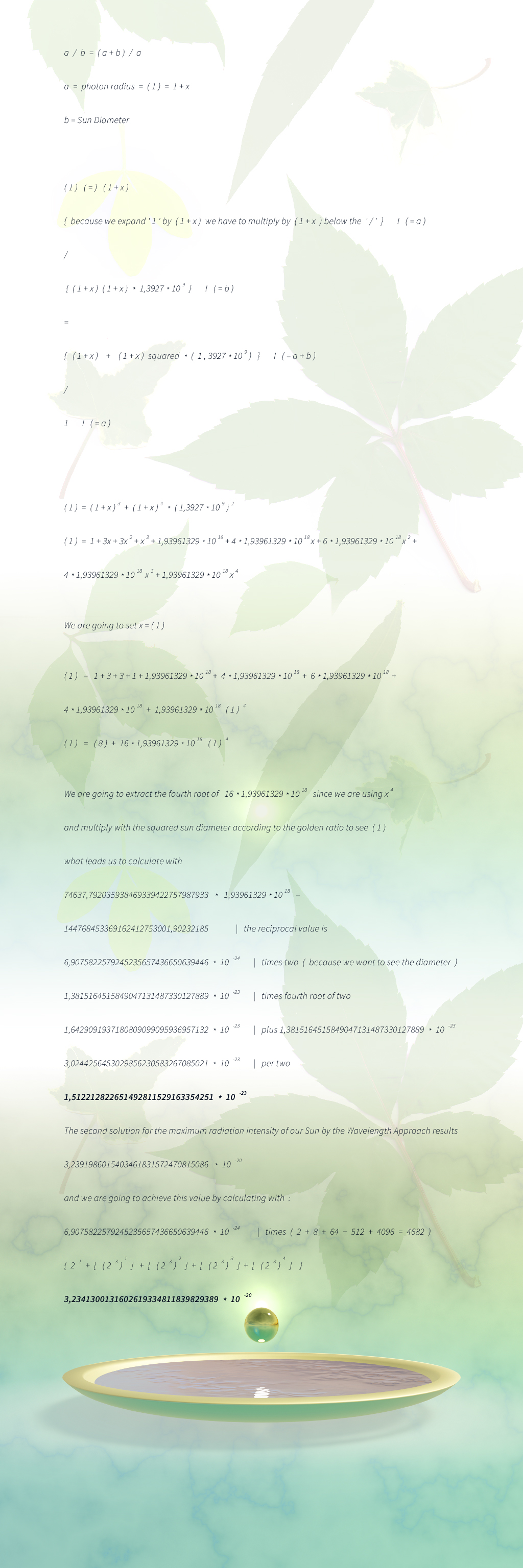' Photon Size 2021 – Golden Ratio Approach ' .
Another reconstructed piece of the Library of Alexandria ...

A valid physical and mathematical prove for this piece of rework
is in progress , and we got to mention that it poses a reconstruction
based on an assumption of some skills during the High Antique ...

A lot of understandings created during the High Antique have been lost
and are difficult to get in today ' s ones – sometimes completely different working – nature of thoughts .
With an assumption for remembrances to be able to be inherited to our children by pure genetical information ...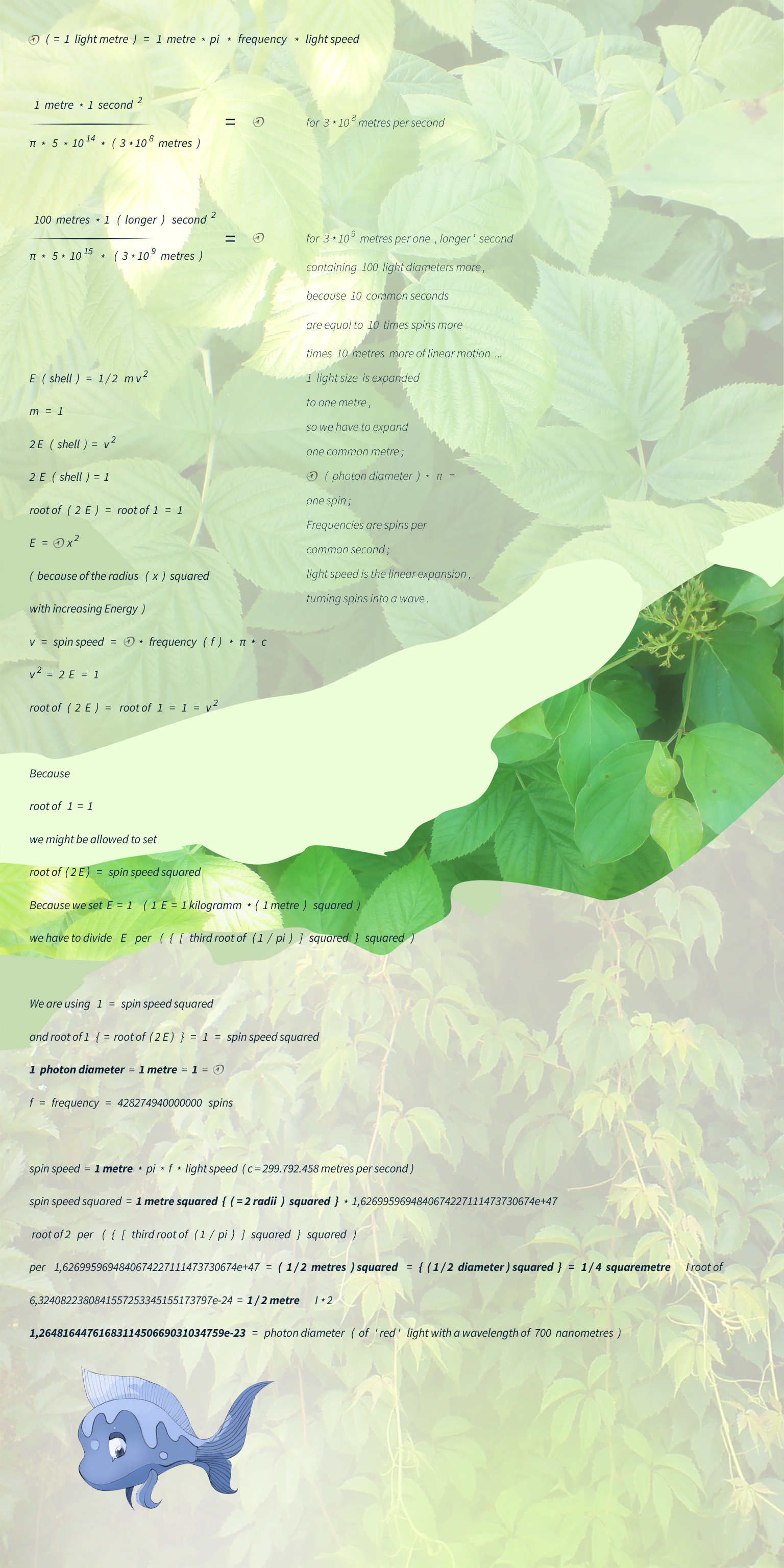' Photon Size 2020 . Frequency Approach '
When extracting the square root of 2 it is not just to our liking ;)-

Calculating with the Frequency Approach , using light speed , pi and frequency – for blue light :

frequency ( f ) of blue light : 749481145000000
f red : 428274940000000
delta : 1,75

f * pi * c = 705880666723841961469264,62058547
root of 1,9878862577579345578272656386017 * { [ ( third root of pi ) squared ] squared } / { ( f * pi * c ) squared } =
6,4872743172225877286583721125891 / 4,9826751565449564820528888300189e+47 =
1,3019661353402250258578501929109e-47   |   ( root of ) and ( * 2 )
= 7,2165535689558212434376551327103e-24

delta blue light Wavelength Approach :
2,5413437584341205380655364594099
1,75 square per 1,9878862577579345578272656386017 plus 1 = 2,5405811011813541323149598948997 ;
2,5413437584341205380655364594099 per 1,75 square = 0,82982653336624344100099149695017 ;
The reciprocal value of 0,82982653336624344100099149695017 = 1,2050711320876149930641848338016 ;
1,2050711320876149930641848338016 square = 1,4521964333909260217517351196627 – the delta of the radius ;
The diameter of blue light calculated by the Wavelength Approach results :

54526390028052756541009,854060104 as an expanded metre   |   1 / x
= 1,8339743369871353013107951920154e-23

The ' Frequency Approach ' has led our idea
to determine the value ' 0 , 5 ' for ' E = 0,5 m v squared ' exactly ,
and that is still a thing we are not a hundred per cent sure
about it to be physically correct , but we are going to give it a trial :
Since we are calculating the size of an electromagnetic particle by

2 * [ root of { ( root of x = ~ root of 2 ) * { [ ( third root of pi ) squared ] squared }
per
( frequency * pi * light speed c ) squared } ] = ( 1 ) ( = photon size )

with the ' Frequency Approach ' we can calculate ' x ' since
the exact Photon Size is known by the ' Wavelength Approach '
and we see ' x ' as 1,9878862577579345578272656386017 ...
The reciprocal value is 0,50304689018166665544978282282439 ... ,
and we may even use that value for calculating with E = ( 1 / ' x ' ) * mass * velocity squared
for solar systems and planets consisting of both positive and negative mass .

Since one year we would like to add the calculation for blue light
( we have seen the result for red light with a wavelength of 700 nanometres above )
and luckily here we go with the ready shaped equivalent ' s values :
( Diameter of blue light ) to the power of 4 is
1,1312875742383230664956598167781e-91
( frequency * pi * light speed c ) squared is
2,4827051715650306584749155670062e+95
( third root of pi ) exponent 4 results
338,72946525108850899893168649641

1,1312875742383230664956598167781e-91 * 2,4827051715650306584749155670062e+95
28086,53511088743226553721087017 I per 338,72946525108850899893168649641
82,917307149727435855353448285833

Now we will see the interesting part of this former work
The delta radius of 700 nm and 400 nm photons is
1,4521964333909260217517351196628
The delta frequency poses 1, 75
1,4521964333909260217517351196628 exponent 4 * 1,75 exponent 4 =
= 41,711293503908460823503389915336 ;

82,917307149727435855353448285833
per
41,711293503908460823503389915336
is equal to
1,9878862577579345578272656386016 ...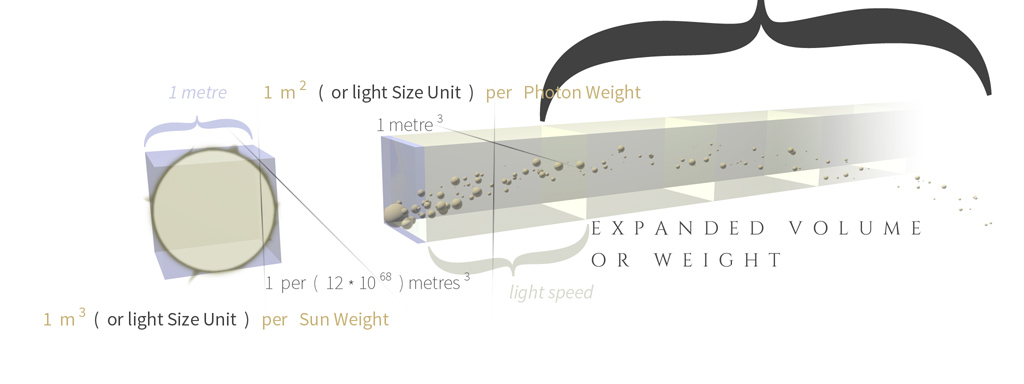' Photon Size 2020 . Classic Approach – Graphical Solution '
Below , we are going to see the entire way of the Classic Approach :

We would like to sum up ( pre ) finally with the ' Classic Approach '
by having calculated the exact mass of our Sun by the exact ' G '
( calculated by the ' Light Speed Approach ' ) , and here we go again :

We have calculated the ~ exact Sun Mass by the new ' G '
( delta measured G and calculated G = 1,0049909870350892935461222363847 )
to 1978525206346527113925355671009,2 kilogramms   | square
3,9145619921485676950475806358989e+60   | * c
1,1735561616195958104777086257893e+69   | cuberoot
105479474953210075506440,38207523   | 1 / x
9,4805174224046213194954628425972e-24   | - 10 exponent 24
8,4805174224046213194954628425972e-24   | times root of pi
1,5031325763012391761300541193832e-23   | times 23,071577649295200737047093070627 th root of pi ( = 1,0510302405504569495503175832494 )
( because we have expanded one common metre to
117917333364366046695060,23513564 light metres
( 1 / 8,4805174224046213194954628425972e-24 ) ,
so we come along with the logarithm of
117917333364366046695060,23513564
what results
23,071577649295200737047093070627 )
1,5795938693837716561070403615765e-23   | 1 / x
63307412074859357170738,9174809

Now we move ahead with the Wavelength Approach in reverse
63307412074859357170738,9174809   | per 10 exponent 18
63307,4120748593571707389174809   | per third root of pi
43225,292050649840950170025690245   | times pi
( ' 1 per third root of pi times pi ' is the same like ' ( third root of pi ) squared ' )
135796,25995559482918671901686504   | square root
368,50544087651518579812572906701   | cube
50041660,64431833701684551593749   | in nanometres
500,41660,64431833701684551593749 nanometres ,
the wavelength of the maximum of radiation intensity ...

And this value should pose the photon sizes of the maximum of radiation intensity .
It should be shifted a little to the left from the value what can be seen within the graphic below
by clicking right here to

be the cause light has to pass through a vacuum which is not perfect until it reaches our Earth ...

We are going to let us know too , how to calculate with the temperature delta
of our Sun and other Stars , like e.g. the Star Betelgeuse :

We are going to use same mass like our Sun to calculate with
since 1 kilogramm on our Sun would weigh heavier on Betelgeuse ,
directly proportional to the higher mass value .
Betelgeuse has a surface temperature of 3.500 Kelvin ,
and our Sun owns a surface temperature of 5.778 Kelvin ,
what results a delta of 1,6508571428571428571428571428571 .
Since we can calculate with ( delta r ) exponent 3 =
= ( delta E ) squared = ( delta frequency ) squared
we are going to see a delta radius r of
1,3968174828249854731611220474704 for the photon ...

We are going to multiply Sun Mass times the temperature delta ,
what results 3265183306639068480812934876634,1 | square
1,066142202595444110629076105927e+61 | * c
3,1962139149362216952711465206494e+69 | cuberoot
147303120107538213663238,51492659 | reciprocal value
6,7887224606644646326882857453331e-24 | * delta r
( delta r = 1,3968174828249854731611220474704

= 9,4826062191027789464805162975533e-24
minus 10 exponent -24 ( = 1 cubical metre ) =
8,4826062191027789464805162975533e-24 | * root of pi =
1,5035028058763799974045012306386e-23 metres ,
what is the size of a sun light electromagnetic particle at the maximum radiation ...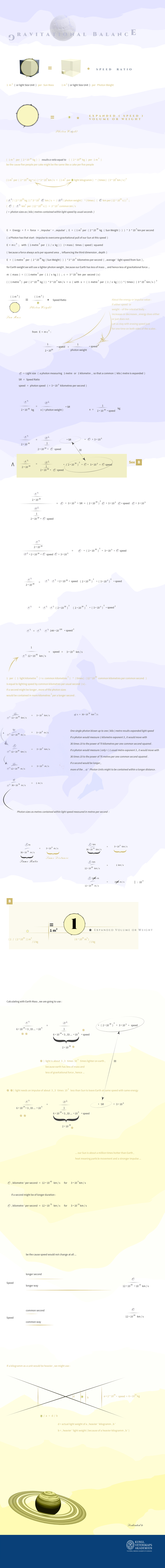' Classic Approach '

We are happy to say that we could continue with our research work
about light during the last three weeks ,
what delivered new and interesting results ,
which would explain gravitational and electromagnetic force
hand in hand with mass of a photon ' s shell .

Our calculations have shown , that the shell sub - quantums
spinning around the core of a photon
might consist of negative mass as well as
the photon core consists of positive mass ...

If we transfer the complexion of this little quantum
( owning both positive and negative mass ) to
other mass objects like a simple breakfast cup
or to our planet earth , we could speak about
each object consisting of positive and negative mass
super - or sub - quantums .

This might explain gravitational force either ,
which is the weakest of all electromagnetic forces ,
because the ' plus - mass - sub quantums ' will attract
the ' negative - mass - sub - quantums ' and vice versa .

Furthermore , this positive and negative sub - quantums - modell
would be a reasonable explanation for
the ' weakness ' of gravitational force ,
because both positive and negative mass ' particles '
might to be contained almost at a balance ,
and the ' strength ' of other electromagnetic energy :

Positive mass should act neutral to positive mass ,
negative mass should repell negative mass and
positive mass should attract negative mass
( e . g . the photon shell spinning around the positive core ,
while the core might even be visualised with an ' Antimatter - loaded ' screen of an oscilloscope ) .

If we would increase one type of mass particles ( either + or - )
we will higher the force of attraction or deflection of both the ' objects '
like we might see it is the case within other electromagnetic energy .

So far have been our thoughts about the latest research
which have led us to give the size of an ' average ' photon core a trial
calculating the way the ' Classic Approach ' works for photon size :
Since we have to get rid of the outer shell weight –
( or more precisely : mass , I am still not sure ,
whether the Classic Approach results weight in relation of the celestial body
or just pure mass , may it to be both is the case ,
and it depends on the way how we think the equivalent ,
1 kg of photon mass would weigh ... on Sun ,
and 100 kg of photon mass would weigh ... on
the 100 times ' heavier ' star R 136 a1 ;
We have decided to use our Sun and 1 kg of mass
to build the scale upon and to adapt it for each other star ) –
we are using 1 / 10 exponent ( e ) 18 for photon core mass
( We treat negative and positive mass like numbers on a value scale )
and as we have removed the shell , the photon would need an impulse
of 1 / 10 e18 lighter , because the negative mass has not to overcome
the gravitational pull ( or electromagnetic attraction ) of
the positive mass sub - quantums in our Sun ...

So we are going to use :

1 metre e 3 / ( Sun Mass * 10 e 18 ) = impulse =
= ( 1 metre e 2 / photon core weight ) * light speed ( 3 * 10 e 8 metres per second )

What should result a value of something about
1 per 10 e 25 metres for photon core size ,
and which is enhanced by a first estimation
using the diametre of our Solar System with
our Sun representing the photon core ...

We are going to continue searching for different approaches ,
like the frequency or wavelength approach for validating photon core size
and in order to determine ' average ' shell sizes !

We have found , that we might be able
to use the ' Classic Approach ' for calculating a single , average shell part also .
( We can only calculate an average shell mass at times )
1 metre exponent 3 per positive Mass proportion of Sun Mass = 1 metre exponent 2 per ( 1 ) kg times ( light speed plus spin speed )
( 1 ) = mass of an average single shell - sub - quantum

This ' Classic Approach ' results cubical metres for expanded Light Speed .
By using the cubical root of mass delta Sun as the value for temperature delta ,
we might have a look at other Stars like ' R 136 a1 ' or ' Sirius A ' and ' Rigel ' et cetera :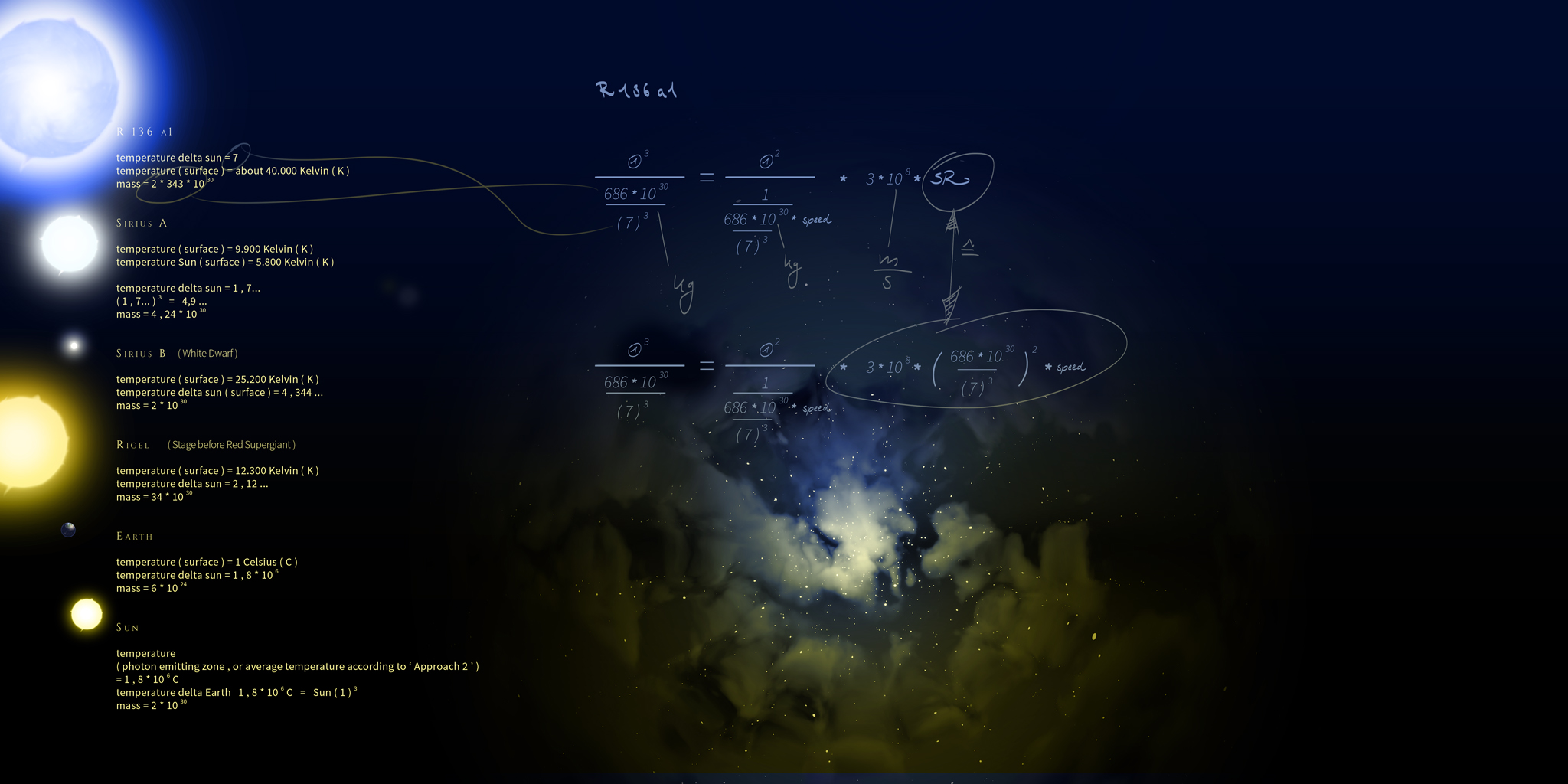' Right click on the graphic in the web browser firefox to enlarge '

What should to be mentioned is ,
that light speed is not expanded at all ( as increasing speed ) ,
but if we expand a single photon to one common metre , we have
to grow metres per second and that is the result ...

Another thing the ' Classic Approach ' represents is
calculating using the mass of other stars
without a temperature delta
photon size expands ( or better it measures more common metres )
what should to be in harmony
with the higher temperature proportional to the photon radius squared ...

If we calculate with the star R 136 a1 –
which is about 7 times hotter than our Sun ,
we will * multiply * the result ( 1 / ... metres ) by
( 7 squared - because we use mass of the star squared )
and then we have to multiply with ( the squared root of 7 ) ,
( for photons leaving R 136 a1 ) as actual metres .
If we calculate with our Sun we have to expand ( * multiply , too * )
photon radius by ( the squared root of 7 )
which has a higher temperature , and hence ,
photon radius increases by root of Energy ( = Temperature ) ...

Finally all the Classic Approach serves for
validating photon size , to catch its size precisely
we might use the wavelength approach either
or just – the easiest way – frequency approach ...

If we regard using the seven times hotter star R 136 a 1
within our equivalents , we might think about the whole
electromagnetic spectrum of radiation to be shifted for
( root of seven ) times of radius and
( root of seven ) times seven times spin speed ,
meaning that ' infrared turns to visible light and
visible light converts to ultraviolet light ' ...
But we have to regard the higher mass of this star also ,
meaning a stronger gravitational pull , what might balance
the energy the photons carry to the same like sun light photons hold ...

I am still working on the calculation using Sun with a temperature of ' 1 ' ...
We do not know yet , if light even might get lighter by higher energy ,
because negative mass is speeded up .

Using Earth weight we are going to calculate
with the temperature delta
' Approach 2 ' ( see below ) delivers .
The temperature delta of the light emitting zone
should result values exactly .

We just have found to a solution for calculating
with the higher temperature of White Dwarfs
beside that some owning a mass same like our Sun ...
For example , if we have a look at the White Dwarf Sirius B :
Temperature Delta of Sirius B and Sun is about 4 ;
Mass is the same approximately .
We regard temperature as Energy :
Temperature = Energy = E = 4 times more than our Sun ;
E = m c c = 4
with c = light speed , m ( mass ) is ' very small '
and can be set to 1 since it is a linear parametre .
Energy should be directly proportional
to speed squared , frequency and a squared radius :
E = Amplitude squared = ( Photon ) radius squared ;
( diametre increases by adding energy )
{ E per ( root of radius r ) } squared = spin speed s ( of the electromagnetic shell )
( 4 * Energy = 8 * spin speed )
1 spin = diametre * pi
1 spin * frequency = spin speed

The speed of light does not change at all –
spin speed of the shell does indeed ...
s = spin speed of the Super - or Sub - Quantums
around the Photon Core = 8
because E = 4 and root of 4 ( = radius ) ;
Resulting a four times higher frequency of the spins
( a shorter wavelength , too ) and
Amplitude = Radius of the Photon = 1 / 2 Diametre
E = Radius or Amplitude squared
E = 4 * Energy of our Sun ;
root of 4 = 2
resulting double photon radius and a four times higher frequency for E = 4

Using the higher mass – but lower temperature – of Red Supergiants ,
we might have seen that energy is less what might mean
lower spin-speed accompanied by longer wavelength , resulting : Red Light !

We will see the radius squared with increasing energy
what goes along with a higher frequency , spin speed and a shorter wavelength hence
which is seen as blue light – we even might talk about a ' Blue Shift ' – the opposite of Red Shift ...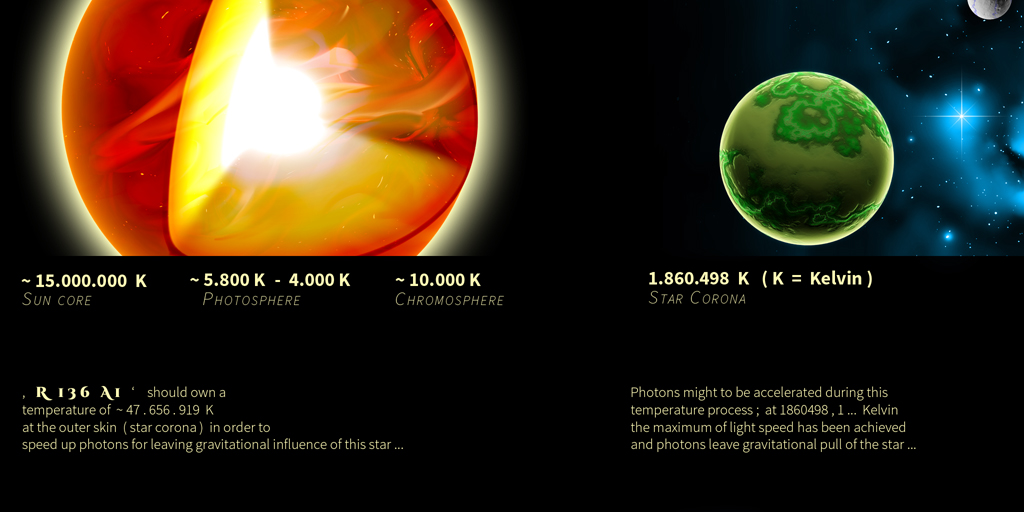' The part responsible for speeding photons up to light speed
while breaking gravitational influence
should to be the star corona .
Photon shell spin speed increases by higher temperature or energy
while light speed stays pretty much the same ... '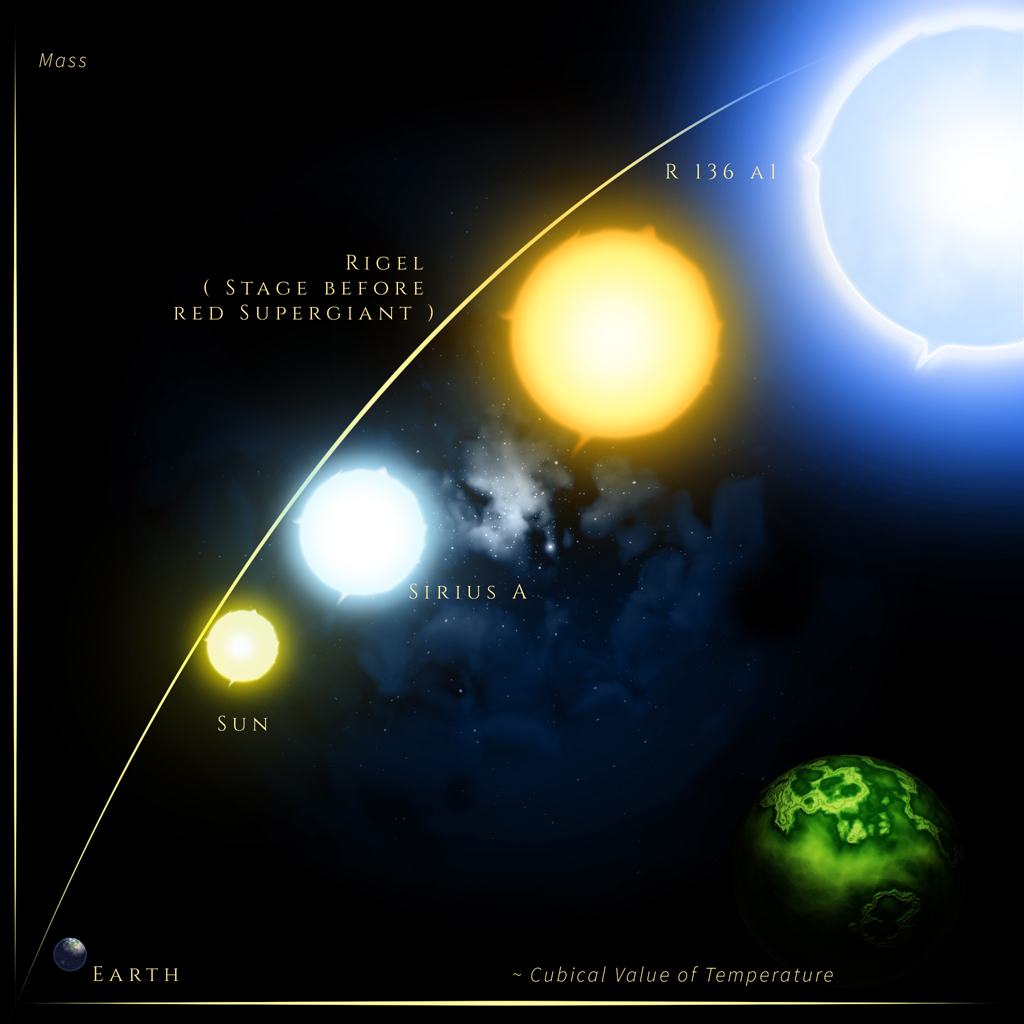' Assuming energy to increase with mass ... '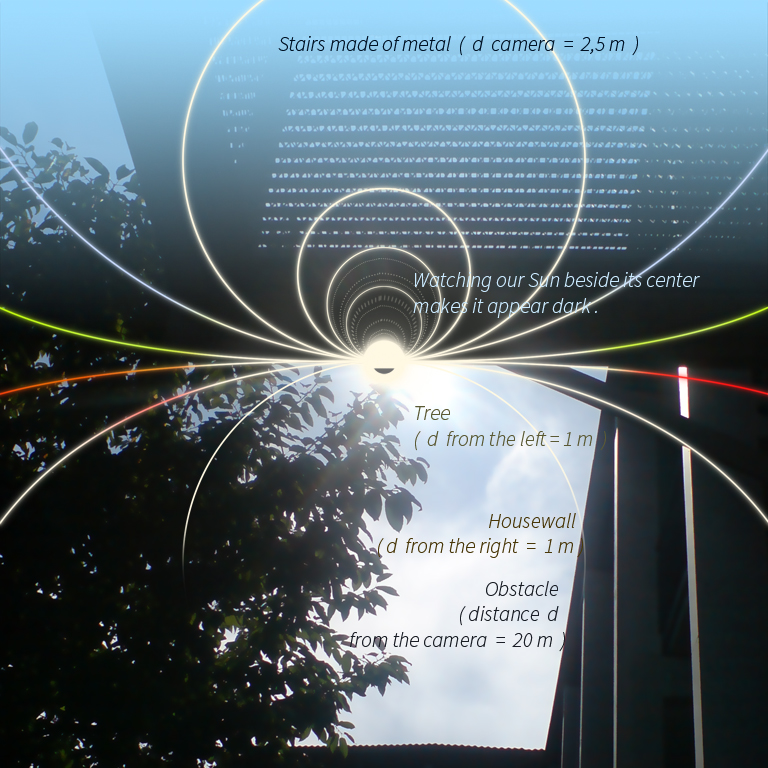When watching light shining through a treetop and then
through glass , we might to be able to see photons with
the naked eye , appearing as little dots which sometimes
seem to move backward very slowly at some viewing angles .
This depends on the very high speed of motion , looking like
moving backward . I have watched this phenomenon
when I was a child , seeing a computer game animation ,
with the background moving very fast what appeared like
moving slowly in a direction counterwise the actual way .
The glass , a simple window in this case , should act as a magnifying glass .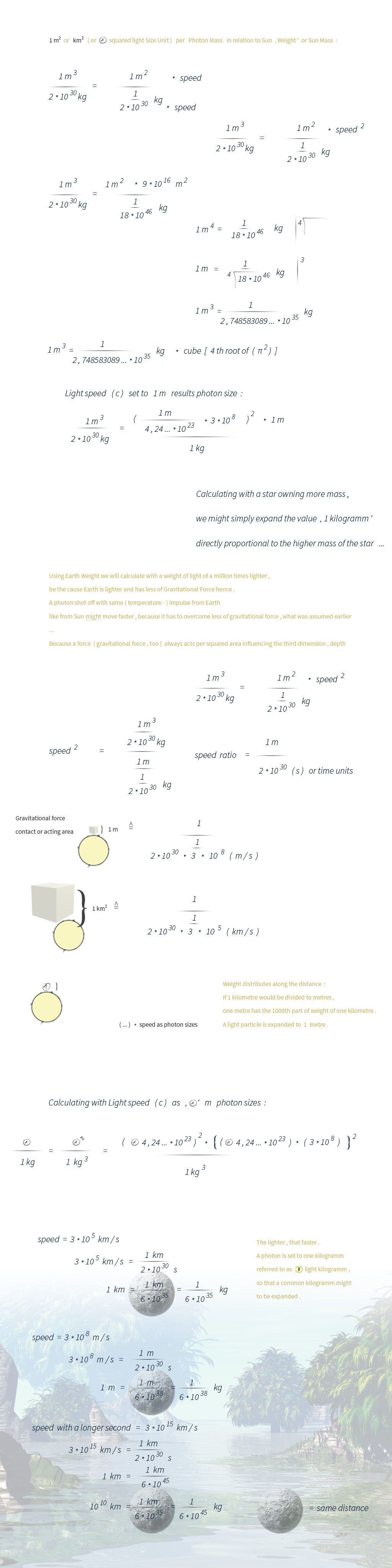Photon Size 2021 ' Diameter Approach '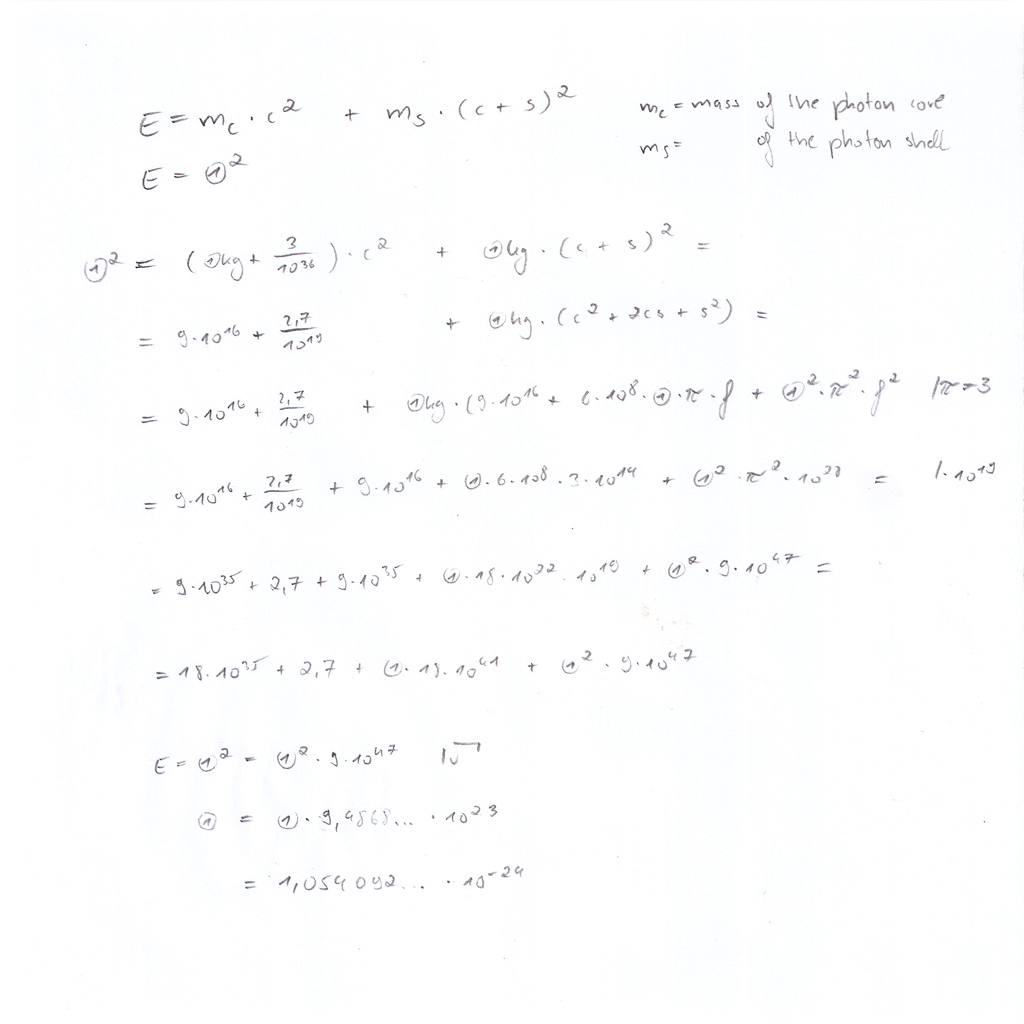' Photon Size 2020 . Diameter Approach '

Actually , the ' Diameter Approach ' results
the radii squared , that is a fact we would
like to have corrected for some time ago and
we go ahead right now with a few renewals :

We will calculate the diameter of red light with
a wavelength of 700 nanometres and
a frequency of 428274940000000 spins ...

( 1 ) = photon diameter
... + ( 1 ) squared * ( pi ) squared * ( c ) squared * ( 428274940000000 ) squared =
( r ) squared = 1,6269959694840674227111473730674e+47

Recognizing a delta of
6,487274317222587728658372112589
for the true value of
2,5079808405275470129776126245674e+46 = ( r ) squared
we are going to calculate this delta by
6,487274317222587728658372112589   per   root of   1,9878862577579345578272656386017   ,
resulting   6,487274317222587728658372112589   per   1,4099242028413919520474070213994   =
4,6011511144704899609836928301589     |   per ( third root of pi ) exponent 4
=   1   ;

Using the ' Diameter Approach ' the following way
results the same size like using the ' Planck Method '
by calculating with h instead of h ( 1 ) :
Energy ( E ) of a red light particle gemini system is
2,837779795927041e-19   e = exponent , we are going to
modify this value to   3523881597279896511,5529858996806 by
having the reciprocal value and then we are going to calculate using
... + 3523881597279896511,5529858996806 * pi squared * frequency squared
what results   6,3792023582791090690759205181408e+48 ,
we will extract the square root and have the reciprocal value ,
ending up in   3,9592854193738104965075353825536e-25 ,
now we are going to multiply with 2 because we have calculated with the diameter ,
7,9185708387476209930150707651072e-25 , multiply this value with pi squared
resulting 7,8153161600441374034235097042169e-24 , and we come along with
dividing per square root of 2 because we have been calculating with the diameter
which is two times the radius and we have extracted the squared root before
resulting   5,5262630538840186993226433108161e-24   ...

The division delta to
1,2628968745672687176015896482243e-23   ( what is the size by the ' Wavelength Approach ' )
is 2,2852637709304626671431070920876   ;
2,2852637709304626671431070920876   squared results
5,2224305027273181459851848605028   ,
and this value is possible to determine by the ' Planck Approach ' ...

Adding Details : Photon Size 2021 ' Diameter Approach ' for Planets , Satellites and Solar Systems

Again , we have found that our latest work about
the Diameter Approach for Solar Systems needs
to be refined . We are going to show intermediate
results , the main problem we have to solve , is to
find out a ratio of 1 metre per kinetic Energy , and
kinetic Energy consists of four factors , ' 1 / 2 ' ,
mass and velocity squared .
By calculating the radius of the shells with the
Antique Approach we are going to see the most
likely case of a planet ( Jupiter orbiting Sun )
or our Moon within an orbit of our Earth , owning
this special kinetic Energy at that certain distance ...

We have been calculating with the following values :

E Solar System Gemini System =
4,8172082086415181941771117935619e+40

E Moon Earth Gemini System =
2,6519229803109859492156955674285e+33

E = radius ( r ) exponent 4 * 5th root of r * 2048th root of ( 5th root of r ) ...

The average distance of Jupiter from Sun calculated by the Antique Approach is
8,8230472535069089155488041494151e+49
Delta E = 1831568591,4674345212024722489001 I exponent 0,24999999999 ...
206,87394524715170287916999259487
we are going to see that the ratio of
1 metre
per
kinetic Energy of Jupiter
( delta mass of Moon and delta speed of Moon vary )
is
206,87394524715170287916999259487

And the average distance of Moon from Earth calculated by the Antique Approach is
8,2830350538360195963118870043452e+35
Delta E = 444,40433460645187889986602277297 I exponent 0,24999999999 ...
4,5913940974382376290686384125964

We will calculate the distance of our Moon from Earth by the Diameter Approach below ...

( 1 ) = the most likely planet radius ( with most of mass )
around a star or the radius of a satellite orbiting a planet
... + ( 1 ) squared * ( pi ) squared * ( c ) squared * ( 1 / frequency ) squared =
radius ( r ) exponent 4 * 5th root of r * 2048th root of ( 5th root of r ) ...

Frequency of Moon is 4,2362203208709831650571062833111e-7
The reciprocal value squared results 5572408187482,2144 ;
5572408187482,2144 * pi squared =
54997464371840,839050680994458454 ;
54997464371840,839050680994458454 * mass ( of the shell , moon in this case ) =
54997464371840,839050680994458454 * 7,31 * 10 exponent 22 =
4,020314645581565334604780694913e+36 ;
Comparing with the value delivered by the radius ,
1,140062208218261291036423165706e+36 , we see a delta of
3,5263993636493639974992043672078 ;

We are going to calculate this delta by
3,5263993636493639974992043672078   per   1,9878862577579345578272656386017   ,
resulting
1,7739442334224207875943649448897     |   per ( root of pi )
=   1,0008408582915393535558186870716   ;

And that way to calculate should work out very nice for
Jupiter ( owning most of mass within our Solar System ) , too ...

Below we see a way to calculate with ' Kinetic Energy = Radius squared ' :

Since we are calculating with the division delta ' kinetic Energy of Moon
and Jupiter ' we see that we are using the value ' 1 ' for kinetic Energy
of our Moon , because we calculate with mass ratio per Speed Ratio
and these are 1 / 1 , so we have to expand ' 1 ' by 1,11111111111 ...
( which is the ' smallest factor ' ) for the kinetic Energy Jupiter holds .

( Gravitational Energy ( Mass in kilogramms ) acting per metre should to be E = m c c ( or E = m v v ) )
and
Gravitational Energy ( Mass in kilogramms ) projected to metre exponent 0,5 should to be E = m squared c ( or E = m squared v )

We are going to have a look at a basic example
E = ' 1 / 2 ' m v squared
mass ( m ) = 2
velocity ( v ) = 3
18 = 2 * 3 * 3     |   root of
because in the second equivalent written above we have metre exponent 0,5 ;
root of 18 * m exponent 1, 5 = 4 ( = m squared ) * 3
4,2426406871192851464050661726291 * 2,8284271247461900976033774484194 = 12

Now , we have seen that the ratio of mass m per Speed Ratio might to be m exponent 1 , 5
and we go on with squaring the average distance of our Moon from Earth of 384.400.000 metres :
r squared = 147763360000000000 = E
1,5 th root of mass of our Moon ( = 7,31 * 10 e22 kilogramms ) = 1748282396377211,7508274264211912

1748282396377211,7508274264211912 / 1,9878862577579345578272656386017 =
879468022656908,2538567713562203
147763360000000000 per 879468022656908,2538567713562203 =
= 168,01447715358763003480568372036     |   root of , because we have velocity squared
12,962039853109063405831891824452     |   per speed of Moon ( = 1022 metres per second )
0,01268301355490123620922885697109 and this value is understood as ' Speed Ratio '
( the factor ' 1 / 2 ' should to be necessary ... )

We are going to calculculate with Jupiter and his distance from our Sun now ,
which is 779.000.000.000 metres by an average
( the square results 606.841.000.000.000.000.000.000 )
Speed Ratio * speed of Jupiter =
0,01268301355490123620922885697109 * 13.060 metres per second =
165,64015702701014489252887204241     |   squared
27436,661619932578281912008911392
mass of Jupiter is 1,899 * 10e 27     |   1,5 th root of =
= 1533498338680500621,1053521475577
1533498338680500621,1053521475577 * 27436,661619932578281912008911392 =
42074075013105661740788,457850306     |   root of , because we want to see the radius
205119660230,57288045335245936384 and the division delta to the actual radius 779.000.000.000 is
3,7977832018848616841703601613517

Now , let us see how we might calculate this delta :
Division delta of mass of Jupiter per mass of our Moon is
25978,112175102599179206566347469     |   1,5 th root
877,14567272324748968456420437809     |   per 1,9878862577579345578272656386017
441,24540289973560109200927291152 ;
division delta speeds = 12,778864970645792563600782778865 ;
441,24540289973560109200927291152 * 12,778864970645792563600782778865
5638,6154225739206949722515696913
5638,6154225739206949722515696913 * 1,11111111111 ...
6265,1282473043563277469461885458     |   logarithm ( log )
3,796929965438155504596750009689

We are happy that we were able to calculate
the speed of our Sun around Milky Way by
using the ' Antique Approach ' and Sun ' Shell '
mass , whereas all of our eight planets
orbiting Sun are understood as Sun ' Shell ' mass .
It works out by the most likely ratio to be 1 / 1
for Sun Speed per Sun ' Shell ' mass ...

And here we go right now :
x = Speed of our Sun around Milky Way ;
( Mass of all of our eight planets orbiting Sun )
to the power of five =
1,3446995650741207209108093594574e+137
e = exponent

[ x * ( pi e3 / 2 ) ] e4 * 5th root of [ x * ( pi e3 / 2 ) ] * 2048th root of ( 5th root of { [ x * ( pi e3 / 2 ) ] } * ... = Sun ' Shell ' Mass ;
|   to the power of 2048     |   to the power of 5

' pi e3 / 2 ' should be because we use the radius of an orbit to be spherical ...

We have decided to get rid of the * 2048th root * 8192th root etc.
for a rough calculation , which should be very tiny in comparison ...
1,3446995650741207209108093594574e+137     |   21th root
3387930,8414229985100784985766743     |   per ( pi e3 / 2 ) =
218531,9363789047094526763741409 ( metres per second of Sun speed )
This seems to work out for Earth speed and our Moon ' s mass also ,
and may even be possible for photons as electromagnetic particles ...

Our result for average Sun speed around Milky Way is 218531,9363789047094526763741409 ... metres per second
A stronger calculator , a bit of patience and the exact Sun ' Shell ' mass will result an exact average speed
( since the speed around the black hole in the center might vary ... )

We have found that the equivalent ' delta radius
exponent 3 is equal to the reciprocal value of delta frequency
squared ' is valid for planets orbiting a star ,
and below we are going to see three examples which are
working out quite nice ...
The equivalent ' E = radius squared ' should be valid for
electromagnetic particles as well as for planets and solar systems .
We have decided to calculate with Mercury and Venus , Earth
and Mars & Jupiter and Earth .
The second part of our latest understanding is , that we will be
able to calculate a new Quantum of Action ' h ' by knowing
frequency of planets , their kinetic energy and the equivalent
E = ' h ' * frequency f
what works out for objects made of Matter and Antimatter both .
Now , we are going to show the examples mentioned above :

The distances ' r ' from our Sun are
58.000.000.000 for Mercury
108.000.000.000 for Venus
what results a delta r of
1,8620689655172413793103448275862 I to the power of 3
6,4563532740169748657181516257328

The frequencies ( spins around our Sun per second ) are
1,3156991751723986943211897457143e-7 for Mercury
5,1508778661750833659280884704893e-8 for Venus
and the delta is going to be
0,39149358480825630504537140466665 I 1 / x
2,5543202719139696938694312769271 I square
6,5245520515106560740960460771043

Now we move on with Earth and Mars 150.000.000.000 r for Earth
228.000.000.000 r for Mars
with a division delta of
1,52 I to the power of 3
3,511808

For the frequencies we calculate with
3,168756727904284686377246116169e-8 for Earth
1,684775986793512776801955526227e-8 for Mars
and the delta poses
0,53168360068706512562228885848205 I reciprocal value 1,8808178373524322666841886238693 I square
3,5374757373030803561521341218919

And our last calculation for this day is
150.000.000.000 r for Earth
778.600.000.000 r for Jupiter
resulting a delta of
5,1906666666666666666666666666667 I exponent 3
139,8522381202962962962962962963

2,6780989354021111989527561923004e-9 Frequency of Jupiter
3,168756727904284686377246116169e-8 Frequency of our Earth
with a delta of
0,08451576329033377682651703592295 I 1 / x
11,832112271940775784655145979806 I exponent 2
139,9988808158115068536406715789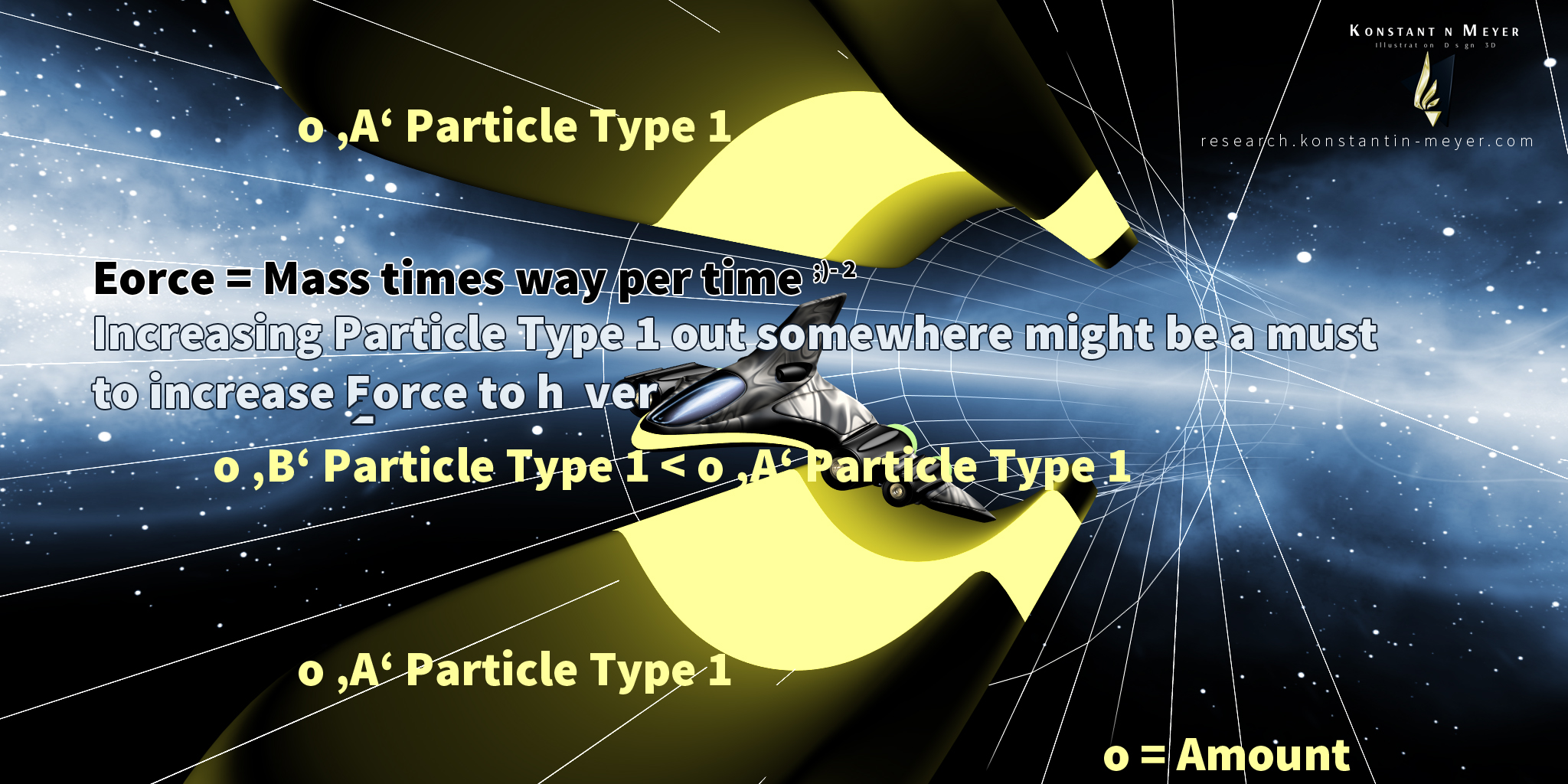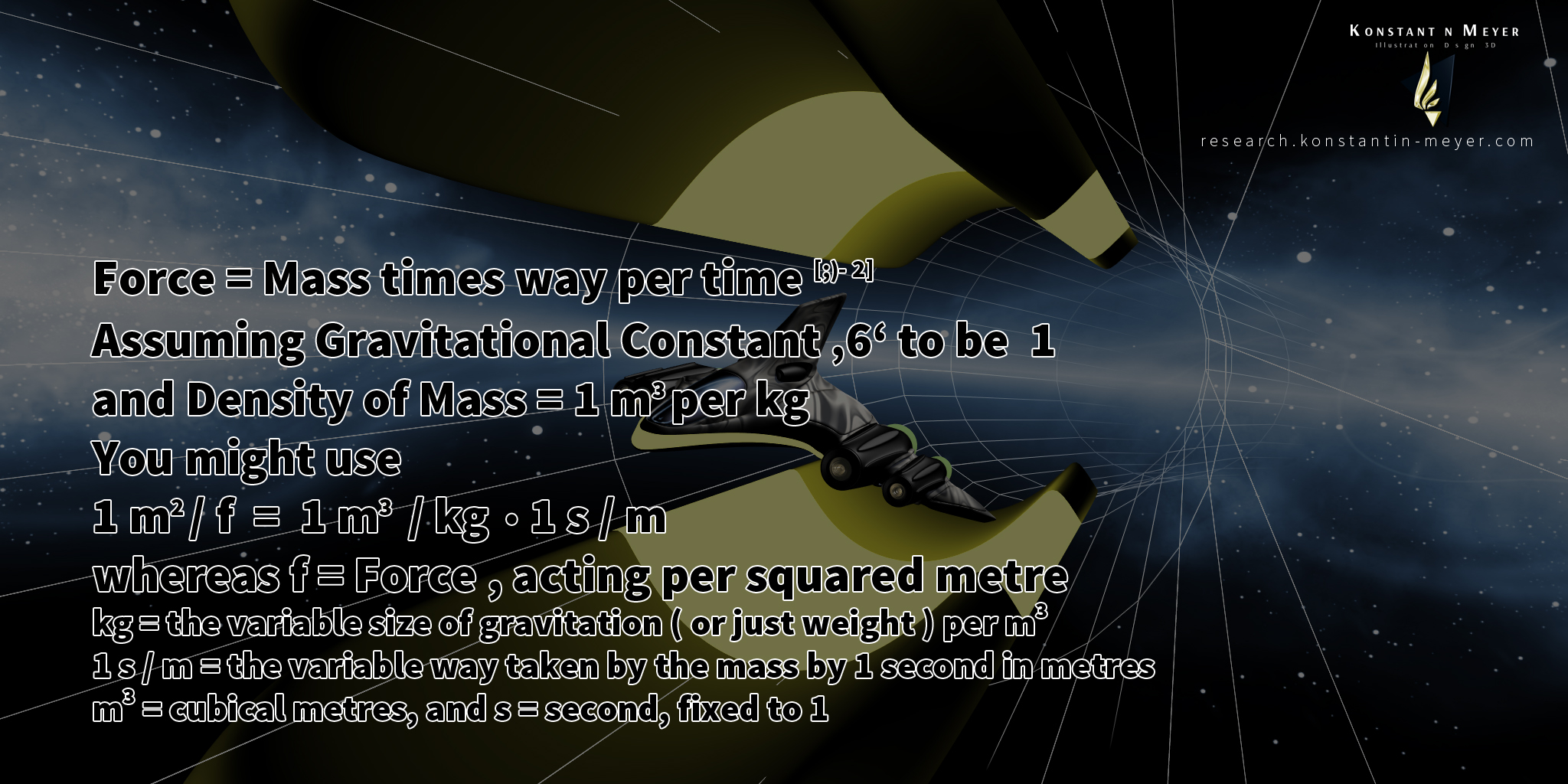' Calculating Gravitational Energy . 2016 . '

Gravitational Energy ( Mass in kilogramms ) acting per squared metre should to be E = m c ( or E = m v )
and
( Gravitational Energy ( Mass in kilogramms ) acting per metre should to be E = m c c ( or E = m v v ) )
and
Gravitational Energy ( Mass in kilogramms ) projected to metre exponent 0,5 should to be E = m squared c ( or E = m squared v )
We might like to add :
( Gravitational Energy acting per point should to be E = m v v v )
and
Gravitational Energy ( Mass in kilogramms ) projected to point per metre should to be E = m exponent 3 * c ( or E = m exponent 3 * v )

We have decided to find a way to determine the value ' 1 / 2 ' exactly :
Since we are calculating the size of an electromagnetic particle by
2 * [ root of { ( root of x = ~ root of 2 ) * { [ ( third root of pi ) squared ] squared }
per
( frequency * pi * light speed c ) squared } ] = ( 1 ) ( = photon size )
by the ' Frequency Approach ' we can calculate ' x ' since
the exact Photon Size is known by the ' Wavelength Approach '
and we see ' x ' as 1,9878862577579345578272656386017 ...
The reciprocal value is 0,50304689018166665544978282282439 ... ,
and we may use that value in order to calculate exact values for kinetic Energy
of Solar Systems and Planets consisting of both positive and negative mass .
Where as the proportion of these both mass types is defined by the ' Golden Ratio ' ,
we use a = negative mass = 10 exponent 20,896214729178052078794838660519
and c or b = entire mass ( positive and negative mass , where as positive mass is a bit in surplus ) .
We target now to enhance this value ~ ' 1 / 2 ' by a ' Golden Ratio Approach ' ,
using
a / c + 1 = ( a + c ) / c
whereas a = 20,8962147291780520787948386605198 ( = exponent photon shell mass of red light , 700 nm )
and c = 36,3157455849006048044077212909739 ( = exponent photon mass of red light , 700 nm )
so we have started calculating with { ( a + c ) / [ ( a / c ) + c ] - 1 } squared .
We try to replace ' a ' by ( a / c ) either or by { ( a / c ) + 1 }
in order to near infinitely close to this ' 1 / 2 ' value ...

Water might to be an exception for these types of mass ( as water is the base for each Life , too ) ...
I have sent a way to determine shell sizes of a photon and this might result negative scale
for the electromagnetic shell size – what might be an explanation for the curvature of space ( and time ) ...

Division Delta of ( cubical roots of both Mass Deltas ( e.g. cuberoot ( Sun per Earth )
per
cuberoot ( Earth per Moon ) ) ) = ~ 16
is equal to
squared ( Division Delta of Speed of increasing Distance of both Celestial Objects
( e.g. ( between Sun and Earth )
per
( between Earth and Moon ) ) ) = ~ 4 ( from Centimetres per Year )

Division Delta Mass means e.g. ( Mass of Sun per Mass of Earth )
per
( Mass of Earth per Mass of Moon )
All this should be explained by
E = m c c ,
using speed squared as Energy acting per squared metre ,
and the ' temperature ' as ' particle movement ( of Planets ) '
within our universe : Using an exponent 3 !

We have seen a new equivalent for Gravitational Force
( resulting Force of Movement ) during the last few days ,
it is quite simple , Force or Energy distributes alongside an area
( a line , or even a point , too ) –
it is like : 10 mass times 10 speed squared acting on a 4 squared area
is equal to 10 mass * ( ( 10 speed squared ) per ( 16 the area acting on it distributes along )

Corona temperature = { ( cubical root of surface temperature ) squared } times surface temperature .
This should work out for a simple candle light
as well for Stars like our Sun .
The cubical root is because of the increasing mass
proportional to a cubical temperature value
and the ' squared ' is because E = m ( c ' squared ' ) ,
heat always means particle Movement ...
It should even be possible to melt iron with a little candle ,
if the piece of iron is held exactly
at the corona hot spot
( which is very tiny in case of a small candle light ) ...

( e = exponent )

Using the ' Classic Approach ' , Light Size ( 1 ) =

= 105.830.148.117.051.055.628.839,62282178
= 1,0583014811705105562883962282178e23
reciprocal value = 9,4491032828752397284138656973619e-24

Calculating with the frequency approach , using light speed , pi and frequency , light size ( 1 ) =

frequency blue light : 749481145000000
f red : 428274940000000
delta : 1,75

* pi * c = 705880666723841961469264,62058547
2,8382616123699438343626193703283e-48
= 3,3694282080910665008361241174928e-24

delta :
2,5374868391178390999596468399708
1,75 square per 2 plus 1 = 2,53125

( Some magical number , we still have to find the reason for is
1,449992479495908057119798194269 )

wavelength approach blue light :

54526390028052756541009,854060104
= 1,8339743369871353013107951920154e-23

Since E = amplitude ( radius of a photon ) squared E = 16 :

Sun should have 16 times more of Energy than a candle light
We will use the ' Hot Spot ' Equivalent :
A candle light is about a thousand degrees celsius ,
for calculating the corona temperature ( using the hot spot equivalent )
we will have to go with the cubicroot of 1000 degrees of the candle light ,
which is 10 and then 10 squared is 100 .
100 times surface temperature of the the candle's 1000 degrees is
100.000 degrees of the candle light
( a very , very tiny really hot spot )
And 100.000 times 16 ( Sun has 16 times more Energy , written above )
results something about 1.600.000 degrees of Sun corona temperature
( which is close to scientifical measurements )

And so , Sun Light Photons should own a four times bigger radius than the candle light Photons –
provided that Sun Light Photon size really increases , and it is strongly assumed that it does not ,
because gravitational force of the ' large ' Sun mass restricts the energy photons carry by attraction ...

1.2 Viscosity of Light and Photons per Cubical Metre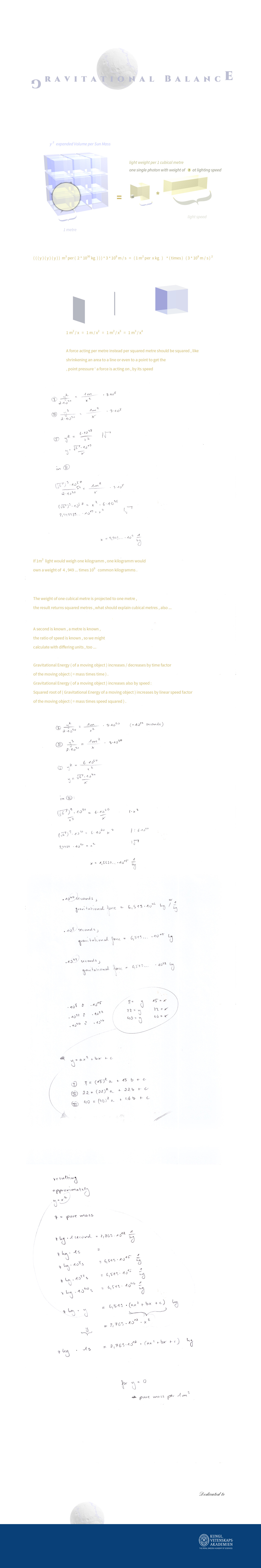Knowing Light Weight per Cubical Metre enables
the calculation of Viscosity of Light and Photons per Cubical Metre ...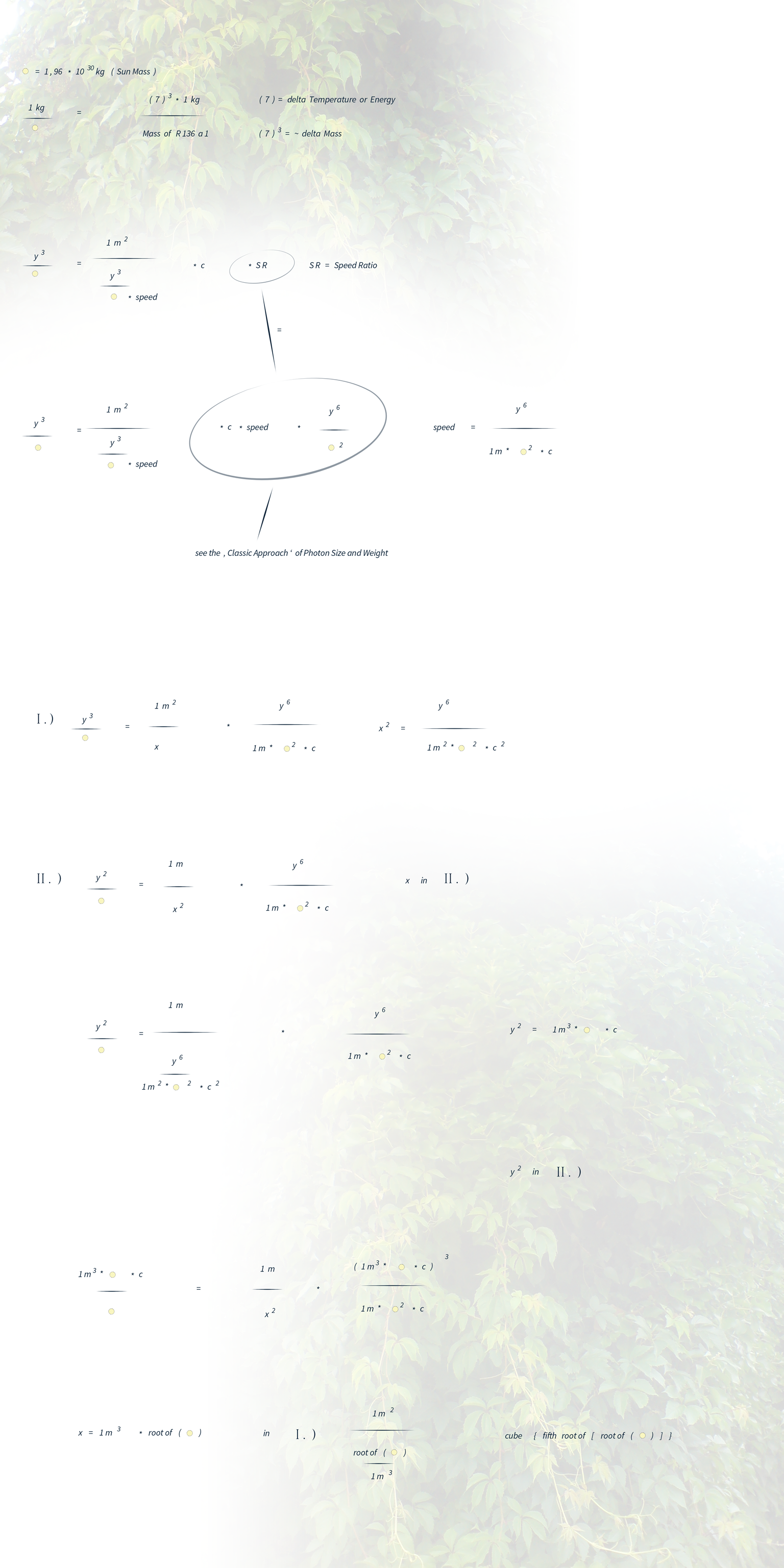Exact Values delivered by using Speed Ratio ...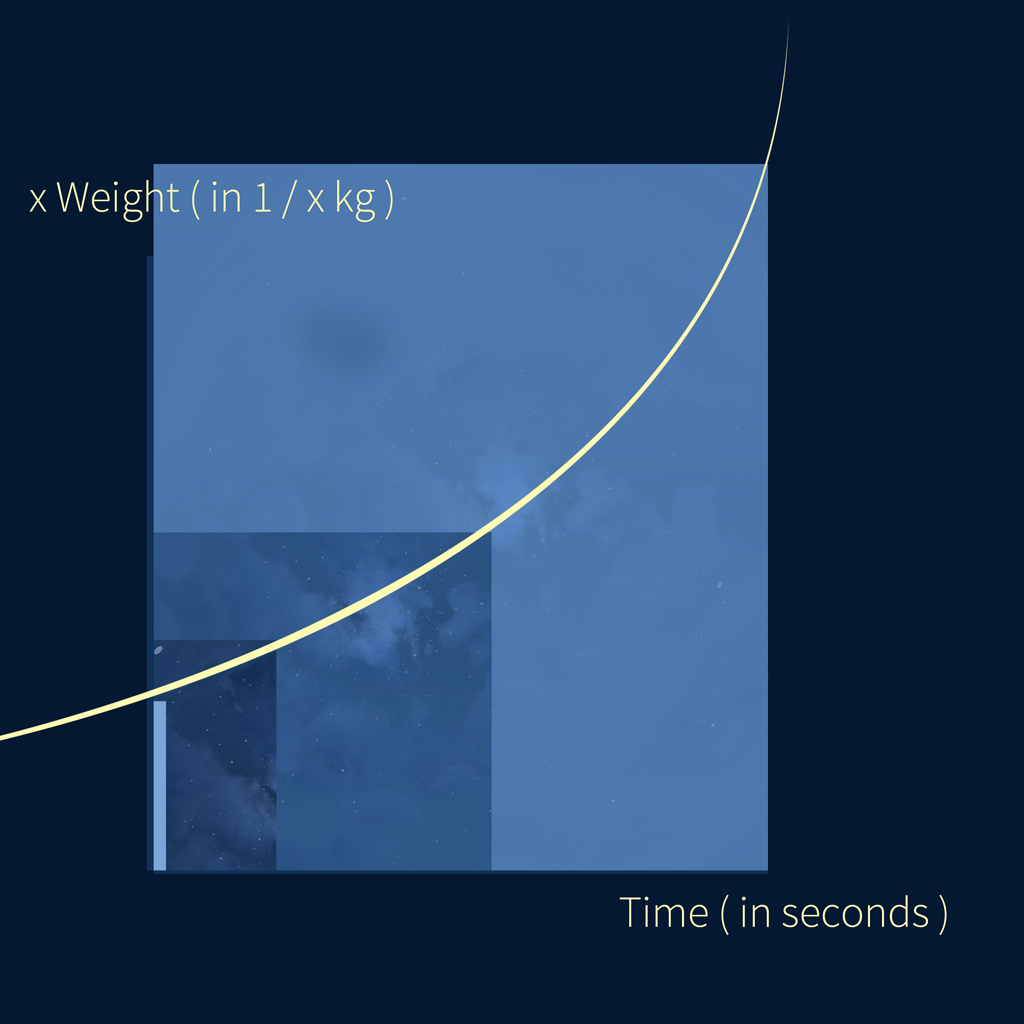Light Weight per Cubical Metre – Graphical Solution .

Weight of light for 1 squared kilometre results 10 exponent 10 ( 1 / kilogramm )
according to the equivalent .
1 metre is not equal to 1 kilometre – but we are still using ' 1 ' ( for squared kilometres )
1 exponent 2 ( kilometres ) = 10 exponent 6 ( metres )
1 ^= 10 exponent 6 .
So we are going to divide the result of 10 exponent 10 through 10 exponent 6
resulting 10 exponent 4 .
As we are using kilometres exponent 4
( because we multiply kilometres squared by squared speed )
we will take the fourth root of 10 exponent 4 to get weight per
1 kilometre exponent 1 resulting 1 kilogramm .
And as we are using kilometres instead of metres
( but both referred to as 1 unit )
we have to divide the exponent 1
per 3 again because
1 ( kilometre ) = 1000 ( metres )
1 ^= 10 exponent 3 .
And than we are going to take the cubical value to get
light weight per cubical kilometre in dependence of one second
which should be approximately 1 kilogramm ...

For the ' y - value ' of y = a x x + b x + c = 0
we are going to see the pure Light Density !

1.3 New Photon Modell and its prove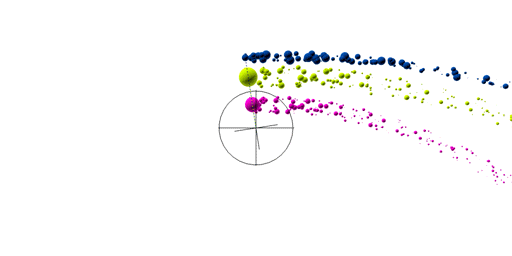' Photon Spin 2020 . Assuming a new photon modell . '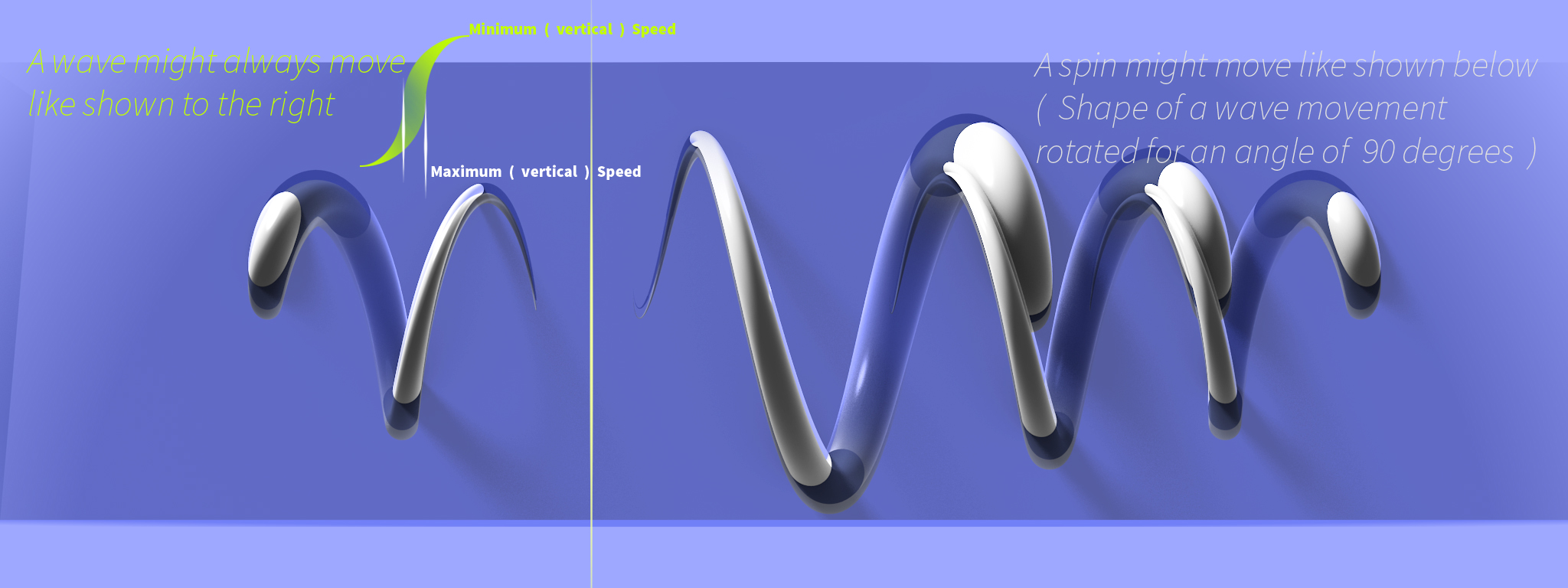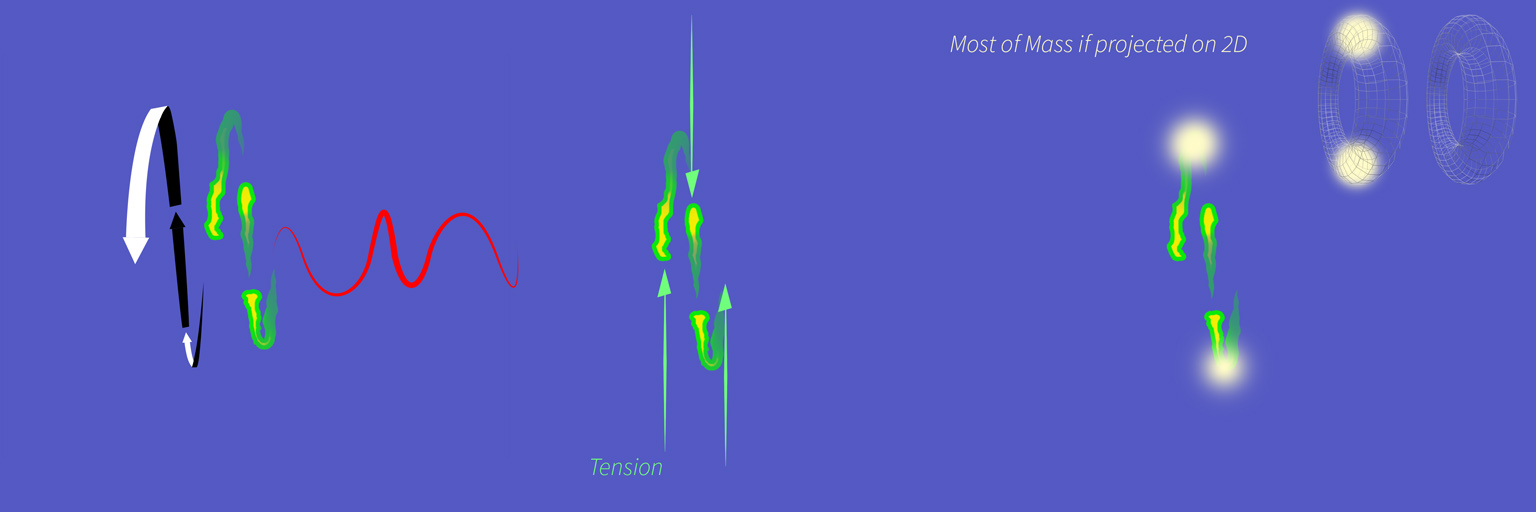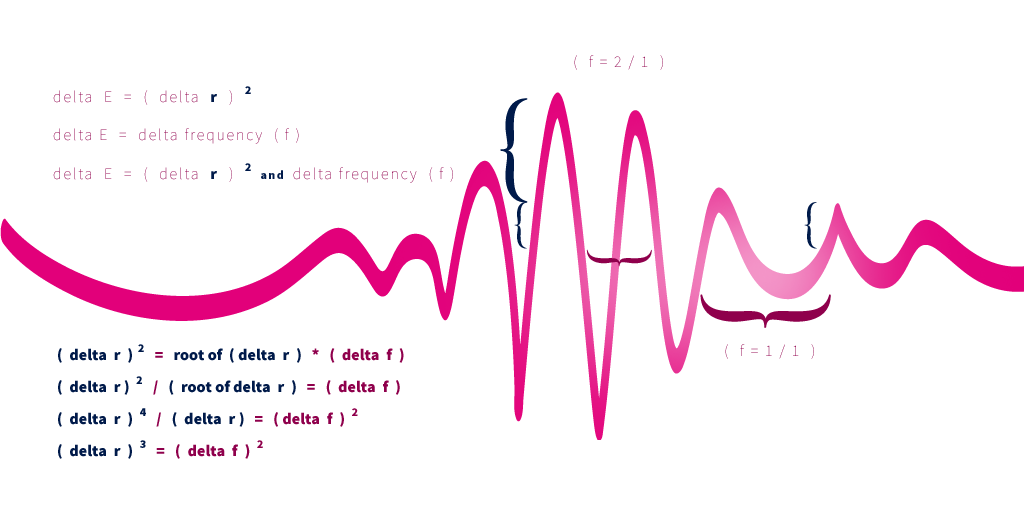' Dependency of Energy , Frequency and the Radius of the electromagnetic Particle System in harmony . '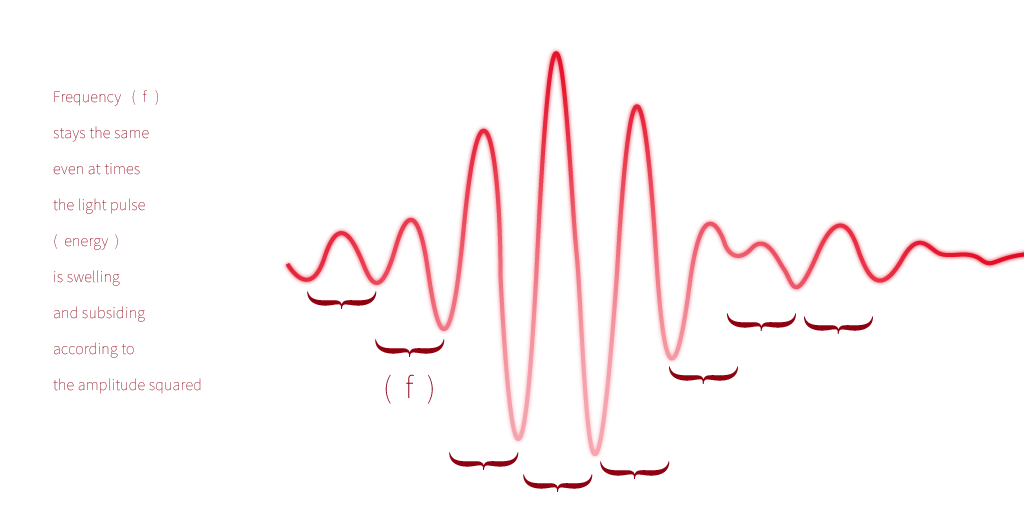' According to this interpretation , frequency stays the same despite a higher amplitude . '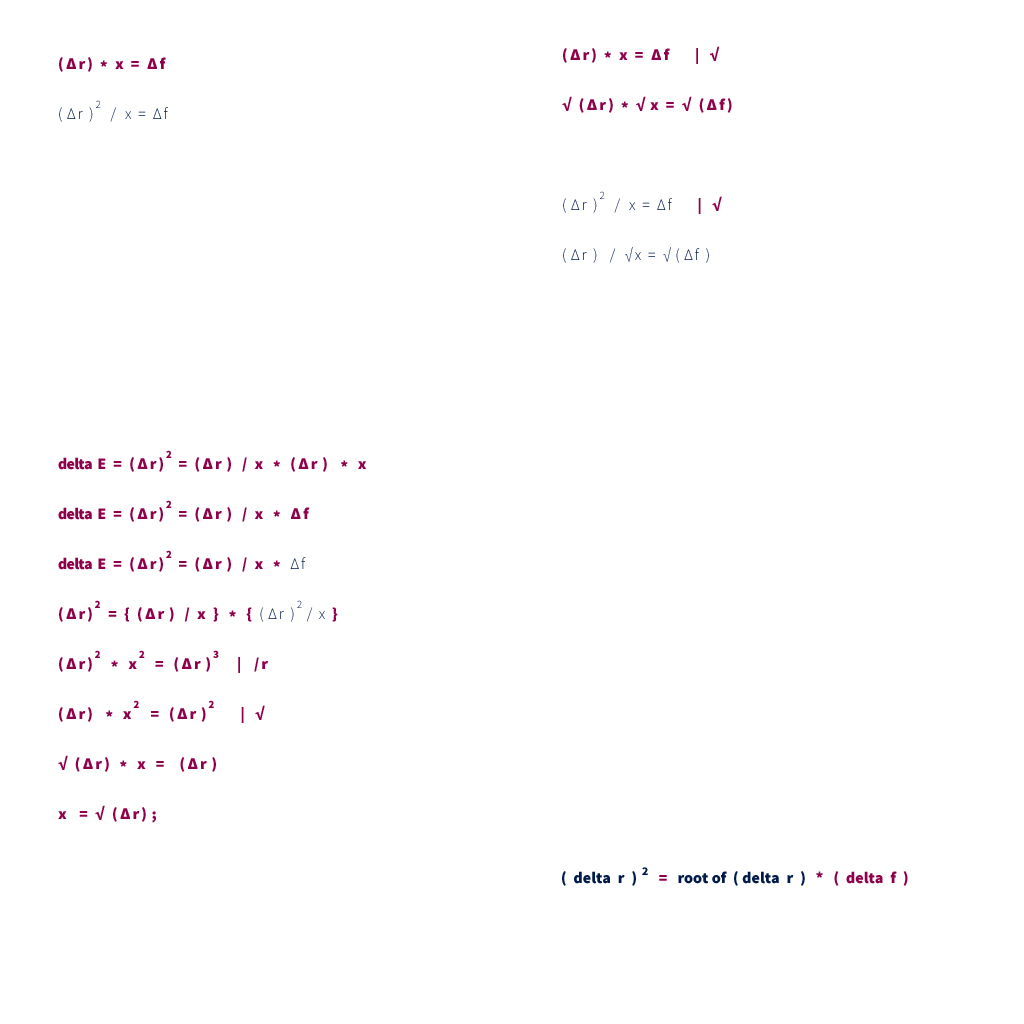The proof for the equation ' ( delta radius ) squared = ( delta frequency ) exponent 3 '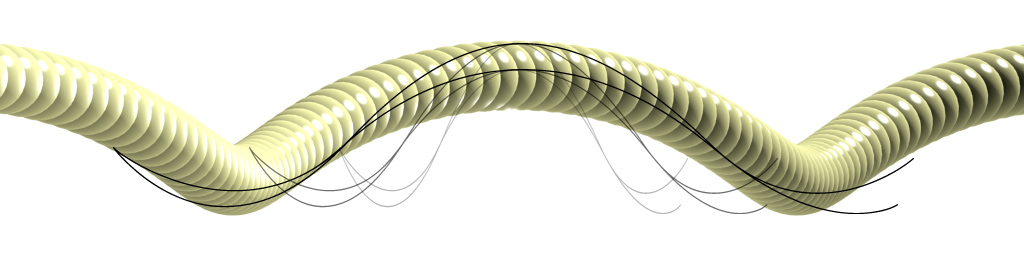' Spin Movement . '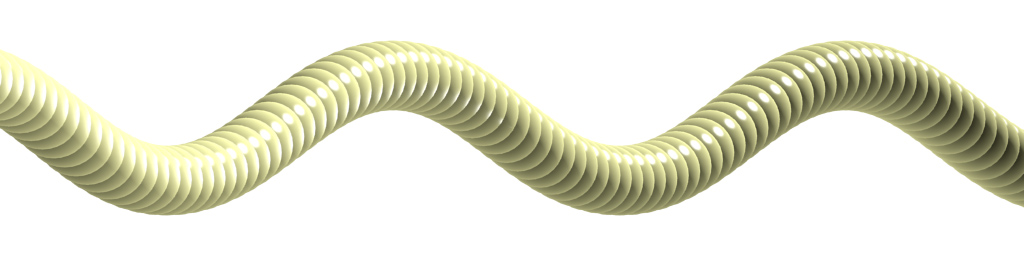' Wave Movement . '' Spin Movement distorted . '
The faster the linear movement of the spinning ' gemini ' system , the more wavelike the shape ...' Wave Movement distorted. '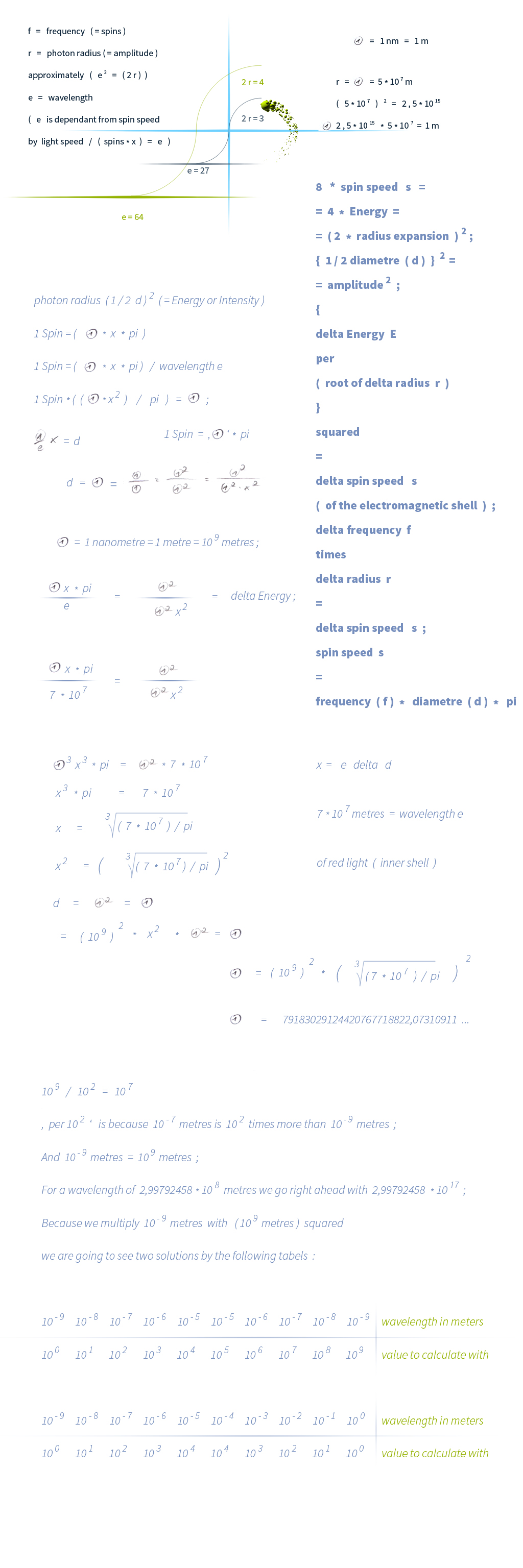' Photon Size 2020 – Wavelength Approach . '

' New elementary Quantum ( I ) for E = r squared . '

Since some time , we have found too , that ' h ' should
pose the Energy of an electromagnetic particle at one spin per second

This new ratio is determined as ' ( I ) ' :
radius r squared ( of an electromagnetic particle with frequency f = 1 )
/
( I ) ( = 8,652126734397744018474064251695e-27 )
=
Energy E ( of an electromagnetic particle with frequency f = 1 ) = h

E ( a ) =
r ( a ) squared /
( I ) /
root of
( 1 / { division delta [ r of 1 spin r ( 1 ) / r ( a ) of an electromagnetic particle with ( a ) energy ] } ) *
{ f ( a ) / [ 1 / division delta [ r ( 1 ) / r ( a ) ] * root of ( 1 / division delta [ r ( 1 ) / r ( a ) ] ) ] }

1.4 ' Golden Ratio plus 1 ' –
( Division Delta of Frequency ) per ( Division Delta of Diametre )

We would like to precise our latest work
about the complexion of a single photon
by adding the term ' Division Delta '
to the Equivalent of the ratio
( Division Delta of spins of the photon shell ) per
( Division Delta of photon diametre ) .

The ' Golden Ratio ' is defined to be

a   /   b   =   (   a   +   b   )   /   a   .

For the ratio of a photon ' s   frequency   ( f )   per its   diametre   ( d )   ,
we are going to use

(   1   +   a   )   =   (   a   squared  +   b   squared  )   /   a   squared

(   1   +   root of   a   )   =   (   a   +   b   )   /   a

whereas
red light ( 700 nm )
diametre ( d ) = 1,2628968745672687176015896482243e-23
blue ( 400 nm )
diametre ( d ) = 1,8339743369871353013107951920154e-23
calculated by the ' Wavelength Approach '

division delta of   ( d )   =   1,4521964333909260217517351196628   ( = a )

division delta of   ( frequency   f )   =   1,75   ( = b )

division delta of   ( f )   /   division delta of   ( d )   =   1,2050711320876149930641848338016

Since we have calculated the photon radius ( r )
by the Wavelength Approach resulting a delta of
1,4521964333909260217517351196628
for the diametres of red and blue light ( 700 and 400 nanometres )
we have come to the conclusion that
Energy ( E ) = root of delta radius ( r ) * delta frequency , and that
E = delta ( r ) squared :
delta ( r ) squared = 2,1088744811533262378623234591529
root of delta ( r ) * delta frequency = 1,2050711320876149930641848338016 * 1,75 =
= 2,1088744811533262378623234591529 .

So we have found out , how Energy distributes
over spin speed consisting of
photon radius ( r ) and frequency ( spins ) :

( root of delta r ) * ( delta f )

/

10 exponent

{

exponent of ten from ( root of [ delta r ] ) plus

exponent of ten from ( delta f ) minus

exponent of ten from ( delta r ) minus

exponent of ten from ( delta r )

}

=

Delta Energy E ( by delta r squared )

An example is going to be :

4 E = ( delta 2 r ) squared

4 E = root of ( delta 2 r ) ( = root of 2 ) * delta frequency

Down here we will see the dependency of the photon ' s radii ( and diameters )
and frequency ( = Photon Shell Spins ) from Energy E :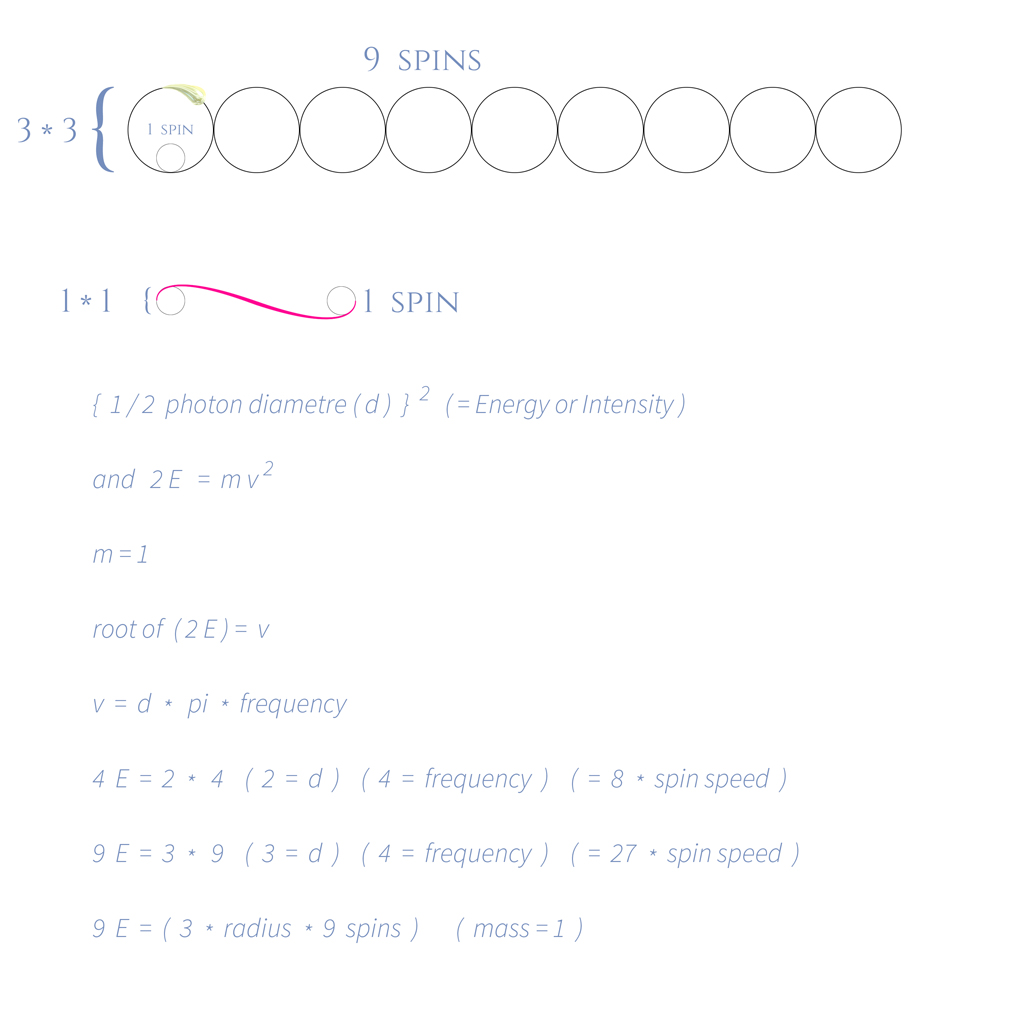Later we are going to calculate Shell and Core Mass of a Photon
using three Approaches , the ' Approaches A , B and C ' ...

A tiny detail has to be added to the term above ,
since Energy = Radius squared ,
but Energy of the shell is not equal to ( radius squared = Energy of the shell and the core )

Summed up and corrected is all of the latest
photon diameter and its belonging frequency :

delta   E   =   root of   (   delta   r   )   *   root of   (   delta   spin speed   s   )

( Delta E ) cube = ( Delta Spin speed ( s ) ) squared ;
Spin speed ( s ) = Diameter ( d ) * pi * frequency ( f )
Delta E = ( Delta ( 1 / 2 Diameter ) ) squared
Delta E = ( Delta ( 1 / 4 Diameter squared ) )

' Delta E ' is named ' E ' within the following parts :

4 E = 1 Diameter squared ( = 1 E ) | root of
2 * root of E = 1 Diameter | * 2
4 * root of E = 2 Diameters | square
16 E = 4 d squared | per 4
4 E = 1 d squared ;
4 E = 8 * s ;
16 E = 4 d * pi * 16 f ;
16 E = 2 * ( 2 d * 16 f ) =
= 2 * { ( root of 2 ) d * root of 16 * root of f ( with f = 1 ) } squared

We have tested with red light { 700 nano metres ( nm ) }

Diametre ( d ) = 1,2628968745672687176015896482243e-23
4 E = 2,5257937491345374352031792964486e-23 { = 2 ( d ) = 1 new , larger ( d ) }
lambda ( according to the Wavelength Approach )
= 438,65994806042508792275892484193 nm
= 4,3865994806042508792275892484193e-7 m
c / wavelength = frequency
299792458 / 4,3865994806042508792275892484193e-7
is equal to 683427924809547,02423507888968654 spins =
= 428274940000000 { ( f ) of 700 nm } * 1,5957691216057307117597842397375 spins ;
1,5957691216057307117597842397375 * root of pi = root of 8

{ 2 * ( root of 2 ) = 2,8284271247461900976033774484194 }

Below , we are going to calculate Shell and Core Mass of a Photon
using three Approaches :

1.5 Photon Shell Mass and Photon Core Mass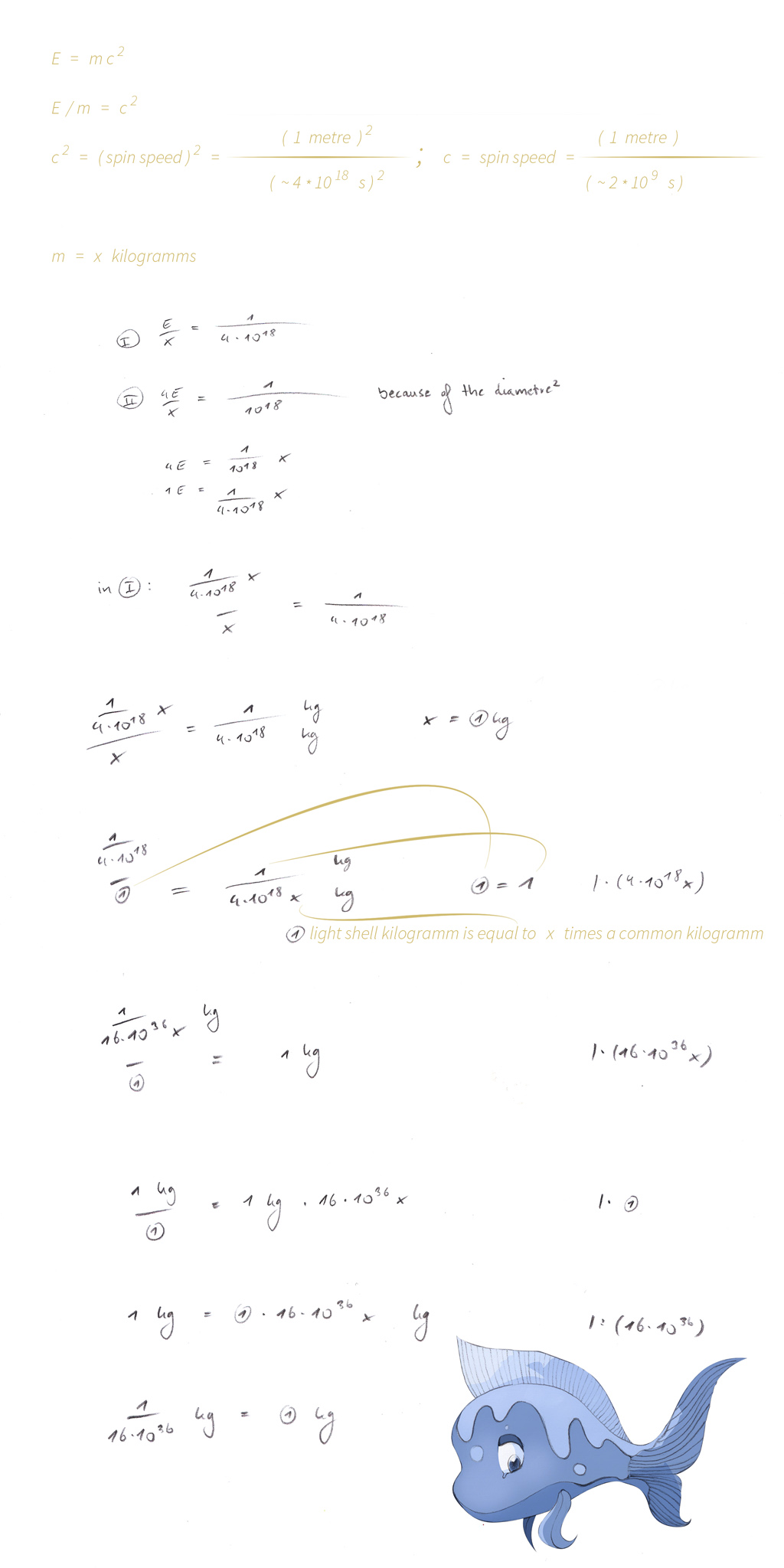' Photon Shell and Core Mass 2020 . Approach A '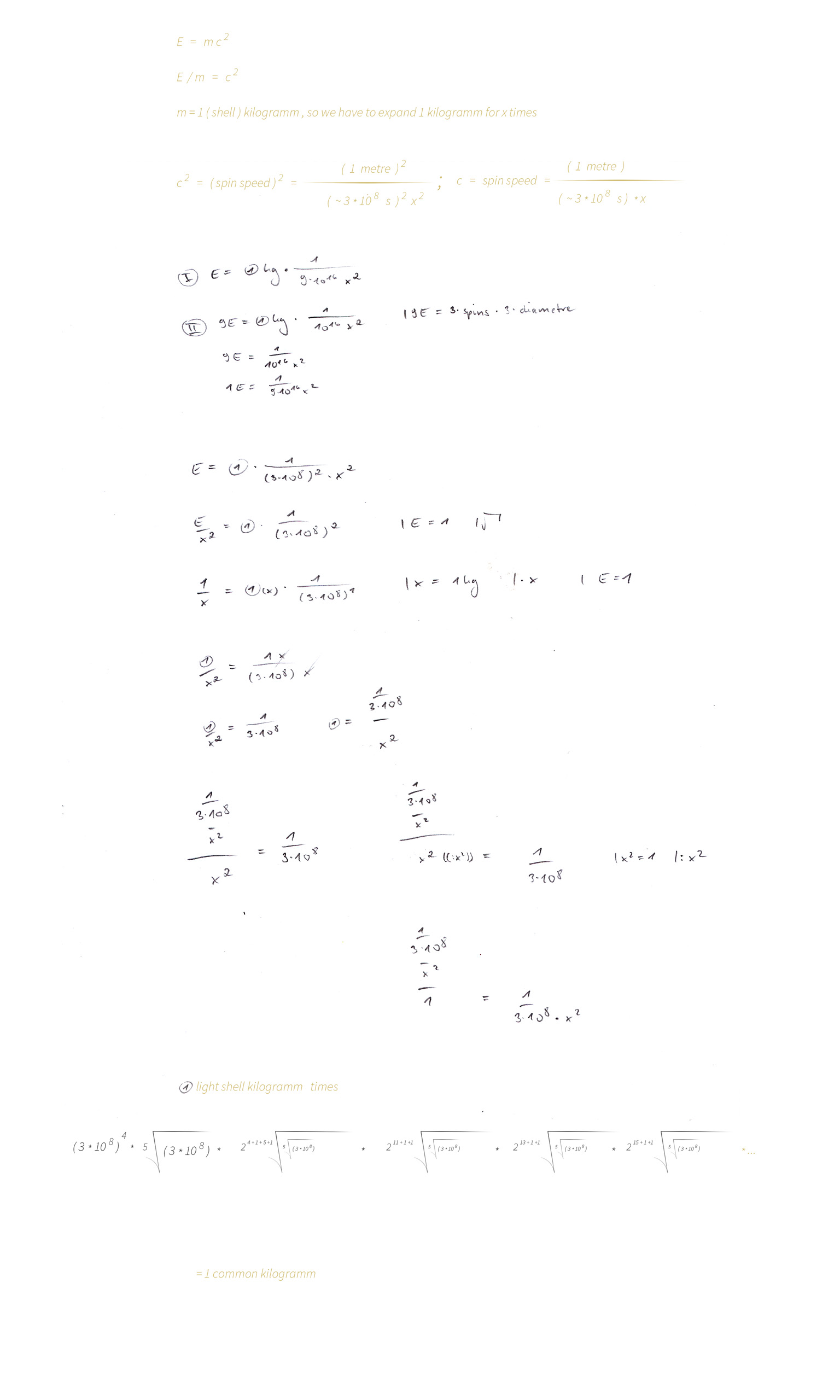' Photon Shell and Core Mass 2020 / 2021 . Approach A polished '
For a mathematical Prove , we will see the ' 1.6 Antique Light Speed ' below ...

Photon Shell and Core Mass 2021 . ' Approach A ' polished & corrected finally :
For a mathematical Prove , we will see the ' 1.6 Antique Light Speed ' below ...

During our last days of work , we have found
to this conclusion what goes calculating photon shell mass
by using the ratio of 1 mass unit per speed ( representing an expanded mass unit ) ...

We are going to start with Energy ( E ) of the shell :

2 E = 1 kg / { ( spin speed ) squared ( = 1 expanded common kilogramm ) }
2 E = 1 kg / { 1 light metre ( 1 ) * pi * frequency ( f ) } squared   | root of
root of 2 * 1 E ( E = 1 ) = 1 light metre ( 1 ) * pi * frequency ( f )   | / root of 2

1 kg ( shell kilogramm ) / { 428274940000000 * pi / root of 2 = 951387711883399,00746937580503269 }

We will have the value ' 951387711883399,00746937580503269 ' to the power of four ,
times 5th root of this value , times
2048th root of 5th root of this value ( and so on ... )
what is ~ 8,1457311378889680506301406011433e+62 ;
Now we have to multiply with the actual size in metres
because we have calculated with ( 1 ) light metre
( * 5,8875504282979383133964130248092e-24 )

what results

4,795840284967804625956909340793e+39 ;

We are going to take the square root and the reciprocal value
what is 1,444001499709211787429441403832e-20 ;

As we have divided per ( root of 2 ) at the start to get 1 Quantum of Energy
we have to multiply with ( root of 2 ) =

= 2,0421265049758561761166972293155e-20 ;

And be the cause we have expanded by ' to the power of 4 times fifth root of ( ) times ... '
we have to multiply with { ( root of 2 ) to the power of 4 , times fifth root of ( root of 2 ) ,
times 2048th root of fifth root of ( root of 2 ) ( ... and so on ... ) }

{ = * 4,287275225259577281211680733266 }

What results 8,7551583716289170530802972929092e-20 ( pre ) finally
which is very close to the Values of Approaches B , C and D and
what might near the actual mass exactly with a bit of a patience ...

We have seen that we have to correct our way to determine the shell masses
of electromagnetic particles and we would like to introduce this refined method
for calculating shells using the Antique Algorithm and the average value of
spin speed of the present electromagnetic spectrum , which is

8,9916951289054100383376381642493e-6 meters per second , calculated by
'
Frequency f of the maxium radiation emitted from the Super Massive Center is
1.134.869.703.006.486.329.734.652.378,7289 ;
The minimum frequency of our present time is
29,9792458 spins per second ( every value below should travel into the past )
1.134.869.703.006.486.329.734.652.378,7289 per 29,9792458 =
37.855.178.565.115.414.935.977.221,239131   |   squareroot
6.152.656.220.293,4282695784294193625 ;

1.134.869.703.006.486.329.734.652.378,7289 per 6.152.656.220.293,4282695784294193625 =
184.451.993.151.075,63421836039794102 ;
( the exact average frequency value of our present time )

The belonging wavelength is 1,6253142775988037966475344418977e-6
with a diameter of
1,5517021010106311346874269957105e-20
( calculated by the Wavelength Approach )
'
8,9916951289054100383376381642493e-6   |   1 / x
111.213,73508153333464045323323964   |   square
12.368.494.870,785478351387056980404   |   exponent 4,200150305 ...
2,4504605181515293034445427434933e+42   |   * 7,7585105050531556734371349785525e-21
19.011.923.672.296.639.161.663,272822464   |   1 / x
5,2598570099308633720305533433966e-23

Calculating the shell mass for this average of present radius by Approach C results
-5,8048685577977809201795823632307e-23
and the delta is
1,1036171794856608260798276971477 ;

Now , let us see the values for red light , 700 nm :

8,9916951289054100383376381642493e-6   |   1 / x
111.213,73508153333464045323323964   |   square
12.368.494.870,785478351387056980404   |   exponent 4,200150305 ...
2,4504605181515293034445427434933e+42   |   * 1,3604144182502841581560313004199e-20
33.336.418.202.464.026.210.356,007810653   |   1 / x
2,9997223874701873464462885273324e-23

Calculating the shell mass for this red light radius by Approach C results
-3,3105959766524303458973928082775e-23
and the delta is
1,1036341197708024931557687540451 ;

Let us move on to the values of an electromagnetic particle at one spin per second :

8,9916951289054100383376381642493e-6   |   1 / x
111.213,73508153333464045323323964   |   square
12.368.494.870,785478351387056980404   |   exponent 4,200150305 ...
2,4504605181515293034445427434933e+42   |   * 2,3943600123792969381313194102613e-30
5.867.284.676.576,2740923603885195712   |   1 / x
1,7043659122119299876442682473471e-13

Calculating the shell mass for this one spin particle radius by Approach C results
-1,8809963732909820832002036433454e-13
and the delta is
1,1036341197705724343502555126753 ;

Finally let us calculate with values of the maximum radiation , emitted directly from the SMC :

8,9916951289054100383376381642493e-6   |   1 / x
111.213,73508153333464045323323964   |   square
12.368.494.870,785478351387056980404   |   exponent 4,200150305 ...
2,4504605181515293034445427434933e+42   |   * 5,612462499403642218893594234753e-13
1.375.311.776.439.467.633.593.991.761.370,3   |   1 / x
7,2710785810973789715366878000373e-31

Calculating the masses for this electromagnetic particle at the maximum radius by Approach C results
m shell =
3,5213133133718465211706455489065e-38 ;
m core =
8,3668238482914078695391737594154e-24 ;
m shell * m core =
2,9462208207625601175756012792726e-61   |   squareroot
5,4279101141807425084898287741202e-31
and the delta is
1,3395724004532145234369355224828 ;

For Approach C we have been using 4 times Energy E owning 8 times spin speed ,
while using 1.000.000 * E owning 1.000.000.000 * spin speed results
a delta of
2,4796874667465969808361847826896
for shell mass of the same radius , we are going to see detailed values later ...

So it might to be an assumption , that shell and core masses might vary for the same
frequency , radius and hence spin speed ; despite we have to remind , that the force of
electromagnetic attraction between the shell and the core differs by varying masses ,
meaning an other radius , what seems to exclude each other at times ...

So we would like to correct a few details within our latest calculations :

The lower the delta Energy , the closer we can get to the exact value of
the shell mass of an electromagnetic particle spinning gemini system ...
For the following new calculations , we have used
1,000000000001 Energy is accompanied by
1,000000000001500000000000375 spin speed
( 1,000000000001 * root of 1,000000000001 )
calculating with Approach C ...

We have seen that we have to correct our way to determine the shell masses
of electromagnetic particles and we would like to introduce this refined method
for calculating shells using the Antique Algorithm and the average value of
spin speed of the present electromagnetic spectrum , which is

8,9916951289054100383376381642493e-6 meters per second , calculated by
'
Frequency f of the maxium radiation emitted from the Super Massive Center is
1.134.869.703.006.486.329.734.652.378,7289 ;
The minimum frequency of our present time is
29,9792458 spins per second ( every value below should travel into the past )
1.134.869.703.006.486.329.734.652.378,7289 per 29,9792458 =
37.855.178.565.115.414.935.977.221,239131   |   squareroot
6.152.656.220.293,4282695784294193625 ;

1.134.869.703.006.486.329.734.652.378,7289 per 6.152.656.220.293,4282695784294193625 =
184.451.993.151.075,63421836039794102 ;
( the exact average frequency value of our present time )

The belonging wavelength is 1,6253142775988037966475344418977e-6
with a diameter of
1,5517021010106311346874269957105e-20
( calculated by the Wavelength Approach )
'
8,9916951289054100383376381642493e-6   |   1 / x
111.213,73508153333464045323323964   |   square
( Because Energy contains speed squared )
12.368.494.870,785478351387056980404   |   exponent 4,200150305 ...
2,4504605181515293034445427434933e+42   |   * 7,7585105050531556734371349785525e-21
19.011.923.672.296.639.161.663,272822464   |   1 / x
5,2598570099308633720305533433966e-23   |   * root of pi
9,32285381246433184136854986384e-23

Now , let us see the values for red light , 700 nm :

8,9916951289054100383376381642493e-6   |   1 / x
111.213,73508153333464045323323964   |   square
12.368.494.870,785478351387056980404   |   exponent 4,200150305 ...
2,4504605181515293034445427434933e+42   |   * 1,3604144182502841581560313004199e-20
33.336.418.202.464.026.210.356,007810653   |   1 / x
2,9997223874701873464462885273324e-23   |   * root of pi
5,3168694973190220217293862203749e-23

Calculating the shell mass for this red light radius by Approach C ,
using 1,000000000001 E = 1,000000000001500000000000375 spin speed , results
-5,1714725962247911498067334050882e-23
and the delta is
1,0281151835166585938325478485442 ;

Let us move on to the values of an electromagnetic particle at one spin per second :

8,9916951289054100383376381642493e-6   |   1 / x
111.213,73508153333464045323323964   |   square
12.368.494.870,785478351387056980404   |   exponent 4,200150305 ...
2,4504605181515293034445427434933e+42   |   * 2,3943600123792969381313194102613e-30
5.867.284.676.576,2740923603885195712   |   1 / x
1,7043659122119299876442682473471e-13   |   * root of pi
3,0209099244521279724190998045936e-13

Calculating the shell mass for this one spin particle radius by Approach C ,
using 1,000000000001 E = 1,000000000001500000000000375 spin speed , results
-2,9382991058635709334376170864052e-13
and the delta is
-1,0281151835167841375046545966632 ;

Then , we might have a look at ' Photon Shell and Core Mass 2020 , ' Approaches B and C ' :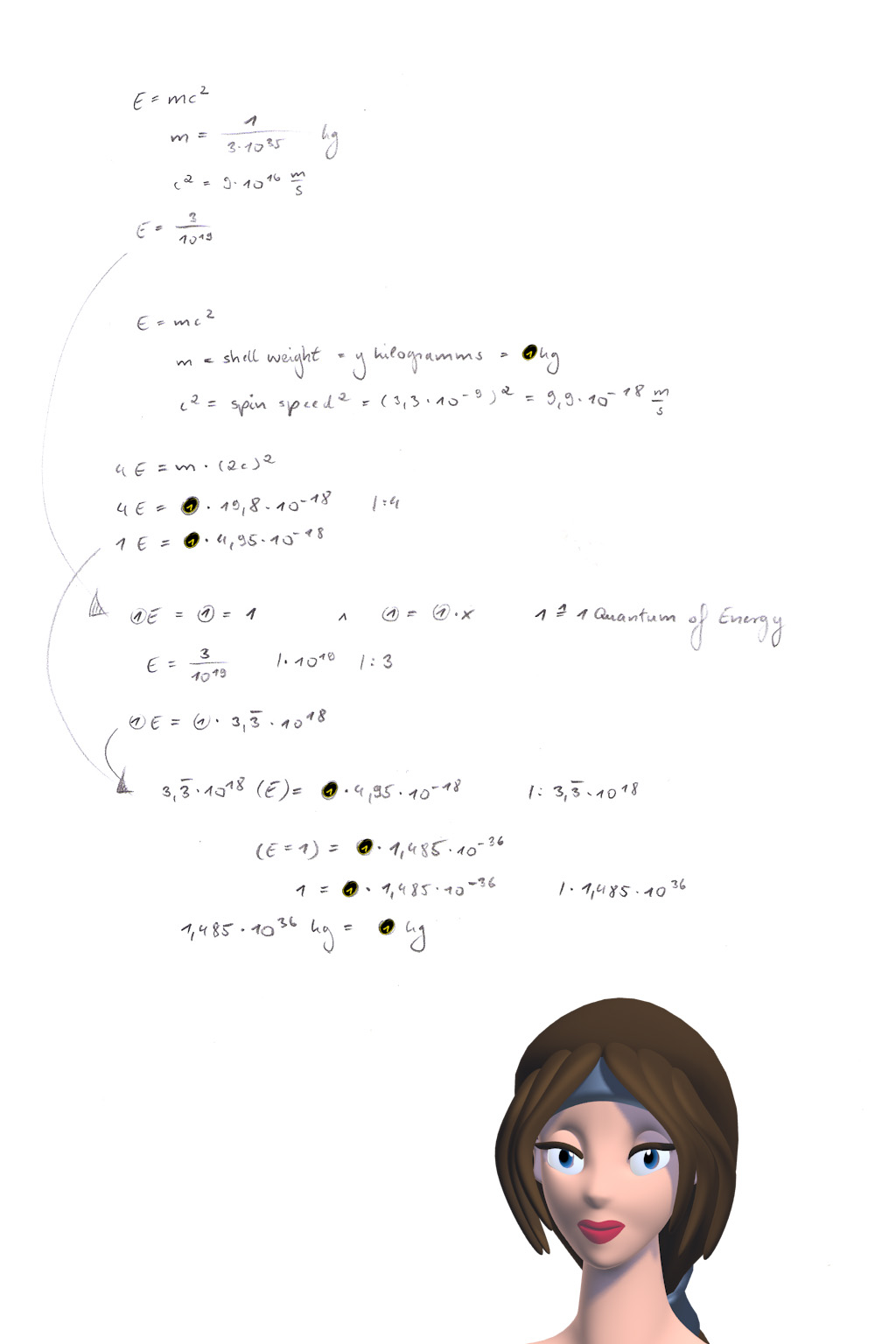' Photon Shell and Core Mass 2020 – Approach B . '

Photon Shell and Core Mass 2021 – ' Approach B ' refined finally :

We are happy to introduce that we were able to precise ' Approach B '
for calculating a ( red light ) photon ' s shell mass .
Here we go right now with the refined ' Approach B ' !

Energy ( E ) of red light ( with a wavelength of 700 nanometres ) =
2,837779795927041e-19 ( e = exponent )
E = 2,837779795927041 / 10 e19

{ Using E = m * c squared ,
with c = 299.792.458 metres / second
and
mass calculated by
m = h / ( c * wavelength ) }

spin speed = 7,921495422766658494420478482012e-9
{ based on the diametre delivered by the Wavelength Approach and an exact frequency value ( f = c / wavelength ) }

1 E = ( 1 ) = 1 / 1 = ' 1 Quantum ' of Energy ( Energy of the shell )
E = 2,837779795927041e-19
( in E is contained a ( pi squared ) because the spinned distance is circular
beside the linear motion of the whole photon system ,
so we have to divide per ( pi squared ) in order to catch the linear movement )

Since we expand ( 1 ) Quantum of Energy we have to ' divide per ' or ' multiply with ' ( root of pi ) .
( pi squared ) is contained in E ( of m photon and is a delta of c squared )
and ( pi squared ) turns to ' ... * ( times ) or just / ( per ) ( ( 1 ) / ( root of pi ) ) ' :

( 1 ) per { [ ( 10 e19 / 2,837779795927041 ) * ( 7,921495422766658494420478482012 ) squared * ( 10 e9 ) squared ] / ( root of pi ) }
= ( m ) shell mass of red light squared as one usual kilogramm per an expanded common kilogramm

And because we use one Quantum of Energy we have to divide the ' ( 1 ) / Energy Value '
per 2,837779795927041 in order to receive ( 1 ) ; Simply see that above ...

Will we believe a result of negative mass for photon shell ,
which would explain superluminal speed well ?
Let us move on straight ahead with ' Approach C ' :

We are going to calculate with :

Photon Core = x kilogramms
Photon Shell = y kilogramms

I )
x + y = Photon Mass ( known )

II )
Photon Energy = x * light speed squared + y * ( light speed + spin speed ) squared

III )
4 * Photon Energy = x * light speed squared + y * { light speed squared + 2 * light speed * ( root of 8 ) * Diameter * pi * frequency + 8 * spin speed squared }

Energy = Light Intensity = Amplitude squared = Photon Radius squared
Diameter * pi * frequency = spin speed
Energy = mass * ( spin ) speed squared

I . b )
( 1 ) = photon mass = x + y

II . b )
E = h * frequency ( f ) = { ( 1 ) - y } * ( c + spin speed of the core ) squared + y * ( c + spin speed s ) squared

III . b )
E ( of maybe blue light ) = h * f = { ( 1 ) - y } * ( c + spin speed of the core ) squared + y * ( c + spin speed s ) squared

{ E per ( root of radius r ) } squared = spin speed s ( of the electromagnetic shell )

Energy E of a photon can be calculated exactly by using
either ' E = m c squared ' or just ' E = h * f ' ;

m = mass ( in general writing ) ;
( we use it for ' matter ' or ' antimatter '
within this special description
of ' Approach C . c ' , like e.g. ' mc 1 ' ) ;
f = frequency ;
( delta m shell * delta m core )
{ is directly proportional to ( delta f ) ? }
which is directly proportional to ( delta r ) squared

Matter of the core of a photon at one spin per second = ' mc 1 ' ;
Antimatter of the shell of a photon at one spin per second = ' ms 1 ' ;
Matter of the core of a red light photon = ' mc red ' ;
Antimatter of the shell of a red light photon = ' ms red ' ;
Radius of a photon at one spin per second = ' r 1 ' ;
Radius of a red light photon = ' r red ' ;

I . c )

{
[ ( mc 1 ) * ( ms 1 ) ]
per
[ ( mc red ) * ( ms red ) ]
}

per

{
( r red ) squared
per
( r 1 ) sqared
}

=

1 ;
because
( delta m shell * delta m core )
{ is directly proportional to ( delta f ) ? }
which is directly proportional to ( delta r ) squared

II . c ) and III . c )

( mc 1 ) + ( ms 1 ) = photon mass of a photon at one spin per second ;
( mc red ) + ( ms red ) = photon mass of a red light photon ;

IV . c )

{
( mc 1 ) + ( ms 1 )
}

per

{
( mc red ) + ( ms red )
}

=

delta f ;
because ( delta f ) is directly proportional to ( delta mass as a sum ) ;

V . c ) ,
VI . c ) and
VII. c )

Photon Energy ( of a particle with 1 spin per second )
= ( mc 1 ) * ( c + core spin speed ) squared +
( ms 1 ) * ( c + shell spin speed ) squared ;

Photon Energy ( of a red light particle ) =
= ( mc red ) * ( c + core spin speed ) squared +
+ ( ms red ) * ( c + shell spin speed ) squared ;

Photon Energy ( of a red light particle ) =
= ( mc red ) * { c squared + 2 * c * [ root of delta ( f ' red per 1 spin ' ) exponent 1,5 ] * mc 1 spin speed +
+ ( delta f exponent 1,5 ) * spin speed of ( mc 1 ) squared } +
+ ( ms red ) * { c squared + 2 * c * [ root of delta ( f ' red per 1 spin ' ) exponent 1,5 ] * ms 1 spin speed +
+ ( delta f exponent 1,5 ) * spin speed of ( ms 1 ) squared } ;

Light speed = c ;
Photon Core = x kilogramms ;
Photon Shell = y kilogramms ;

The equation has no solution for a red light
particle spinning ( gemini ) system , calculating
with a wavelength of 700 nanometers in this case ...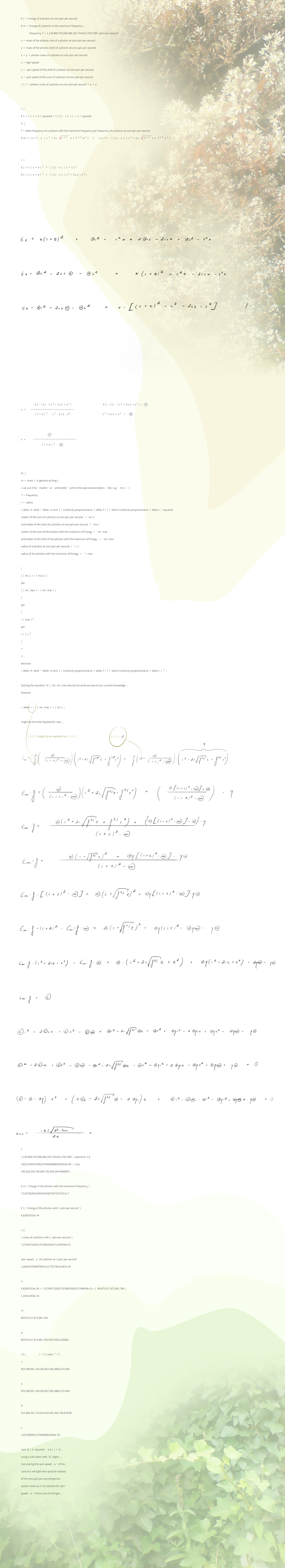Below , we are going to precise our results ,
using the values of red light instead of the
maximum energy photons :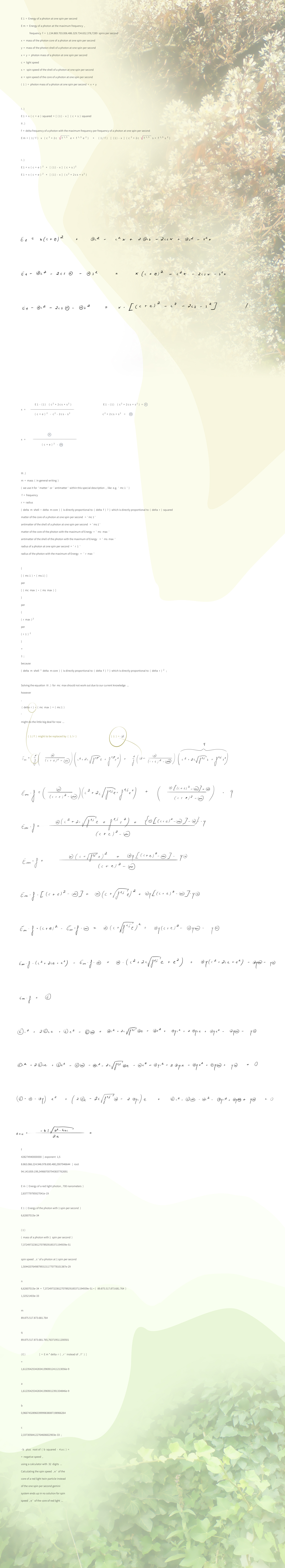' Photon Shell and Core Mass 2023 – Approach C . c ,
calculating Photon core spin speed equal to zero ' ;
As well as we do not see a solution as an exact value ,
we can understand our result calculating with all possible
values to result zero movement of the photon core
( like using ' 1 / f ' or ' 1 / r ' and other possible values
within the equation II . ) for the common , up today known electromagnetic spectrum .

At these days it seems , that the photon core spin speed
of a ' one spin per second particle ' results negative speed
and if the particle system reaches some certain level of
energy , acceleration of electromagnetic attraction is
that less in comparison to the force by moving straight
forward , so that a spin of the core does not occure ...

After all , we are going to recognize , that Albert Einstein has been right ,
when seeing that no ' matter ' ( = mass , consisting of both Matter
and Antimatter ) is able to move faster than light :
We , our Moon , our Breakfast Cup , etc. all consist of both Matter and
Antimatter ( resulting the ' weakness ' of Gravitational Force ) and
since Matter can only travel back into the past by a very low level of
Energy photons hold while Antimatter travels back into past in parallel
by nature with this associated level of Energy , we are going to see only
one possible direction through time , backward , for the twin particle system
in case very low Energy .
And if Antimatter is speeded up to superluminal speed by a very high
amount of Energy , it travels into future while Matter does the same ,
like usual for us . So we are going to realize , that both states of low or
high Energy photons carry , are not possible at the same time up today .
So photons ( these little ancestral or primary kind of particles everything
consists of ) cannot travel through time as a twin particle because they cannot
own both states of high and low Energy at the same time up to this day ( like known for us ) ...

Since Antimatter exists from Future to Past , we will see , that superluminal
motion of Antimatter reverts this circumstance , so that superluminal
Antimatter moves right into the Future what we will recognize as the
' Smallest Circular Flow of Magnetism ' in our present , bending space ,
what can be seen in our work about the big bang .
And that the ( possible ! ) superluminal motion of Matter exists into
our Past , because Matter exists from Past to Future ...

During yesterdays work , have come to the conclusion , that superluminal
motion of Matter might exist right in the past , so that it is not possible to
measure this kind of electromagnetic radiation within our present time ...
The expanded electromagnetic spectrum : Reaching from the particles
emitted directly from the Super Massive Center up to photons which exist
only and ' forever ' at a certain point in the Past !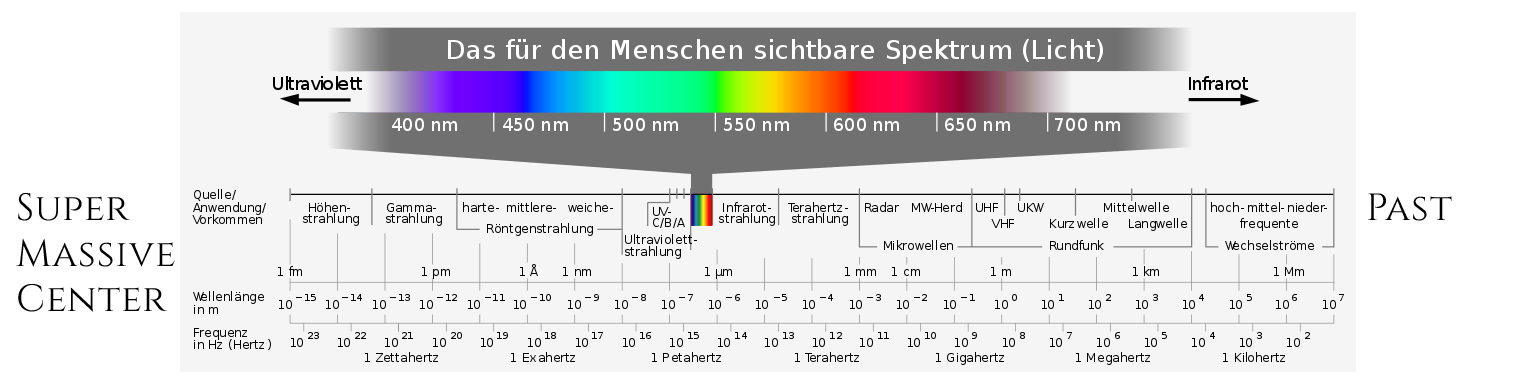CC BY-SA 3.0
Horst Frank / Phrood / Anony / Konstantin Meyer

We have corrected this tiny detail , which has affected the result in a very
important manner , here we go right now with time travelling of Matter !
We have recalculated the value for the parameter ' c ' of our quadratic
equation , which can be seen above ( not light speed right here ) to
2,33730584122764606822903e-33 ;
So we might see a ' negative speed ' for the photon core spin speed of
a photon at one spin per second , for this photon moving with light speed ( c ) ;
That might to be described also as meters per negative time , meaning
nothing else than travelling a distance – backward through time ...

We have seen that the equation ' delta products of the shell mass of a photon
and its core mass to be equal to the delta radii squared ' might result true values ,
even for the maximum radiation of the Super Massive Center :

The frequency of the radiation of the SMC ( Super Massive Center ) is equal to
1.134.869.703.006.486.329.734.652.378,7289 spins per second
Mass of the shell returns
3,5213133133718465211706455489065e-38
( Antimatter turns into positive Mass by superluminal speeds ,
that is why we are going to multiply with the value of frequency ) ,
Mass of the core as a valid result is
8,3668238482914078695391737594154e-24 ,
Mass of the shell times mass of the core results
2,9462208207625601175756012792726e-61 ;
2,9462208207625601175756012792726e-61
per
1 / 1.134.869.703.006.486.329.734.652.378,7289
results
3,3435767478503329938672318338932e-34 ;
5,612462499403642218893594234753e-13
2,3943600123792969381313194102613e-30
for a photon at one spin per second ;
the delta of both these radii is
4,2661487941054609113264802176884e-18   |   square
1,8200025533447478315350331045731e-35 ;
The division delta of
3,3435767478503329938672318338932e-34
and
1,8200025533447478315350331045731e-35
is
18,371275038628955542224068728035   |   per 1,862268410022608512766339873271
( per the exact ratio of meters per kilogramms )
9,864998482364807228886839415715   |   squareroot
3,1408595133123683265150333777927   |   delta pi
1,0002334202705716493625026561581 ;

At times , our single explanation for this slight delta is based
on rounded or cut off values within our Windows Calculator ,
which uses 32 digits , because we have been calculating the
following two delta values by the equation mentioned above ,
' delta ( shell mass times core mass ) per delta ( radius r ) squared ' :
1,0000000000005122856112697628493 delta 1 spin per second photon and red light ( 700 nm ) I per two
1,0000000000002561428056348486201 ;
1,0000000000007896082294664422101 delta red light ( 700 nm ) and blue light ( 400 nm ) I per two
1,0000000000003948041147331431698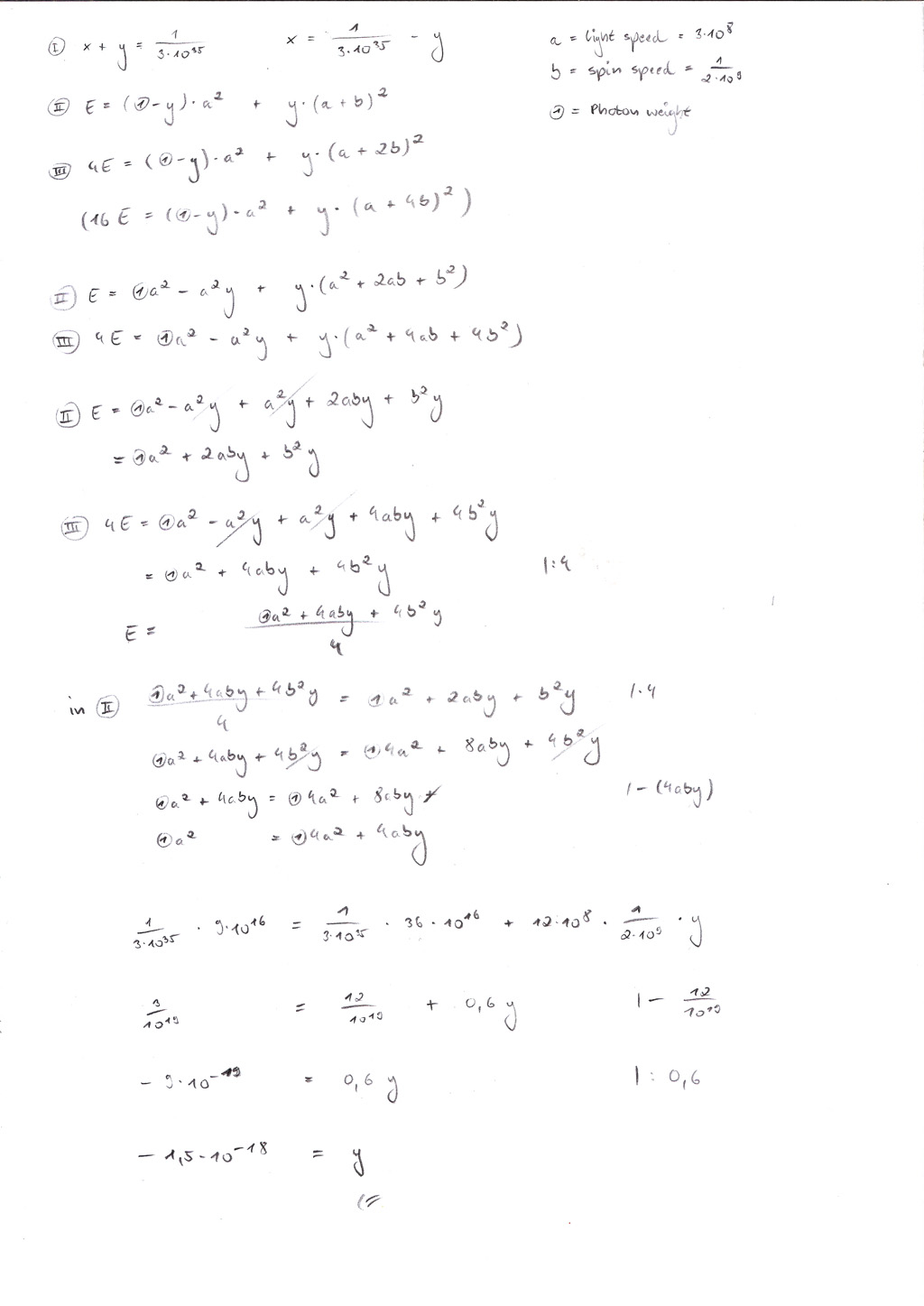' Photon Shell and Core Mass 2020 – Approach C . '

It is only a logic that – starting with 1 kilogramm for shell weight of a photon –
the result is the positive value of x squared negative kilogramms .
The reason is the following :
Starting with 1 shell kilogramm to be an expanded common kilogramm
can only result positive mass , because 1 shell kilogramm is yet positive .
But if we use negative mass , to simplify we use - ( 1 / 3 ) = 1 shell kilogramm
we will see that ( -1 ) = 3 kilogramms
and that ( -1 ) ( - 1 ) = 9 kilogramms ( the squared value )

I )
1 / 2 d * pi * ( c + s ) = 9,2481425865901218670656733756264e-24 * ( c + s ) =
= ( 1 / f = 2,3349486663870643470290370013244e-15 ) * root of root of ( 1,9878862577579345578272656386017 ... ) = 2,7725233979683304730471674687042e-15
1 / 2 d * ( c + s ) * pi = 8,8252160724919586497184437908039e-16 * pi = 2,7725233979683305463062866374713e-15

II )
1 / 2 d = root of negative m ( shell ) * { ( 1 / frequency ) = 1 spin } * 2 * root of pi * root of ( 1,9878862577579345578272656386017 ... )

whereas

d = photon diametre ( = 5,8875504282979383133964130248092e-24 ) according to the ' Wavelength Approach '
m ( shell ) = mass of the photon shell ( = -6,3371951240106583218717495029571e-20 kilogramms )
according to ' Approach C '
f = frequency of 700 nanometres ( = 428.274.940.000.000 spins )
and
c + s = light speed plus spin speed of the shell around the photon core
( = 299.792.458,00000000792149542276666 ... metres per second )
( ( for some reasons ) it seems to work out for nearing exact values ; it should be the Energy = ( 1 / 2 d ) squared what does the deal once ,
and using the radius of a photon to be ' 1 ' what should be in harmony with the ' Frequency Approach ' )

1 ) E = photon radius ( = 1 / 2 d ) squared = Amplitude squared
2 ) E = photon core mass ( = m ( core ) ) * light speed ( = c ) squared plus
photon shell mass ( = m ( shell ) ) * ( c plus spin speed ) squared
2.1 ) E ( photon ) = E ( photon core ) plus E ( photon shell )
2.2 ) m ( photon ) = m ( core ) plus m ( shell )

The ratio of photon shell mass per photon mass might to be :

a   /   b   +   1   =   c   /   b
whereas   a   =   19,198102920620302695573476544672   ( = exponent photon shell mass of red light , 700 nm )
and   c   =   36,3157455849006048044077212909739   ( = exponent photon mass of red light , 700 nm )
and   b   =   delta   a   c   =   17,117642664280302108834244746301
a   /   b   =   1,1176470588235294117647058823529
c   /   b   =   2,1176470588235294117647058823529

Actually , the ratio method described above works out for each value ...
We have found to another , new conclusion :

logarithm   (   1   /   mass shell )   /   logarithm   ( frequency )   *   logarithm   {   1   /   [   (   cuberoot   of   2   )   squared   ]   plus   pi   squared   }
is equal to
logarithm   {   1   /   [   (   radius   *   2   *   pi   )   squared   ]   }   /   logarithm   {   mass of the whole photon   ( shell and core )   }

logarithm   ( mass shell )   =   19,198102920620302695573476544672
( frequency )   =   428274940000000
logarithm   ( frequency )   =   14,631722662913670723680049949633
logarithm   {   1   /   [   (   cuberoot   of   2   )   squared ]   plus   pi   squared   }   =   1,0211713034379207271790951438432
logarithm   {   [   1   /   (   radius   )   *   2   *   pi   ]   squared   ]   }   =   48,65855044806320159717378050027
logarithm   {   mass of the whole photon   ( shell and core )   }   =   36,3157455849006048044077212909739

1.6 Reconstruction Research : An antique Way to Calculate Light Speed ...

I have had this idea some time ago and I have worked to my best
to reconstruct this assumed ' ancient ' way to calculate light speed ,
which might had been lost for over 2000 years ...

The reason why this ' antique ' method should work out fine ,
is that we are targeting the most likely ratio of diameter per distance :
If our Moon would be heavier it would be ( larger and ) closer to our
Earth due to gravitational force , and if it would own less mass , it would
be ( smaller and ) farer away . The density of our Moon also obeys physical laws ...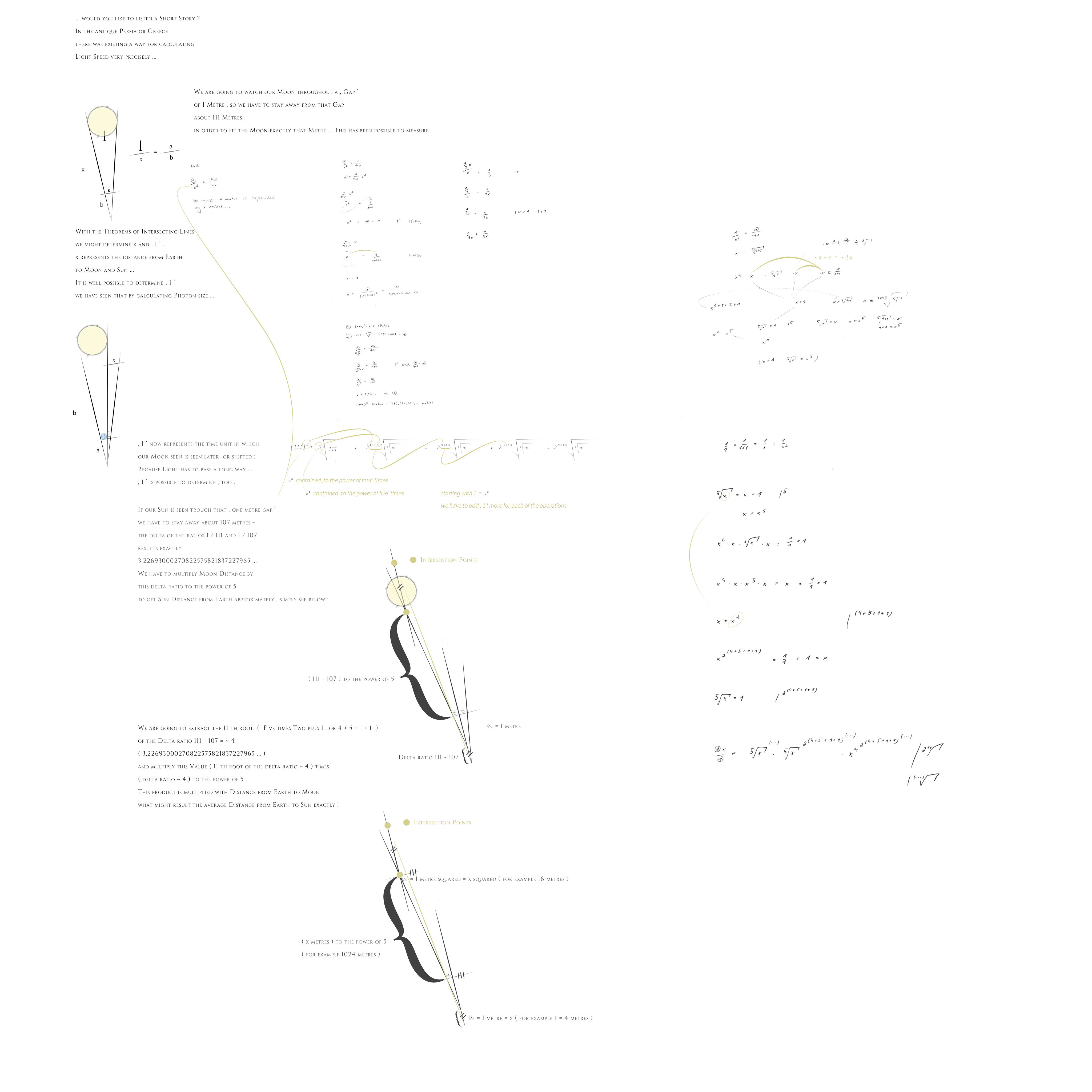' An assumed antique Way to calculate Light Speed ...
Right click on the graphic in the web browser firefox to enlarge '

We have seen that it is possible
to calculate moon distance from earth by
using the ratio 1 / ~ 111 ( = 110 , 65 ... ) .
So it is possible too , to calculate photon mass
by using just a photon ' s speed ;
Since we are using 1 per 1
( which is the most likely ratio – 1 size per 1 weight ) ,
we can use ( c ( light speed ) to the power of four )
times fifth root of c ,
times 2048th root of ( fifth root of c ) and so on ,
to calculate one photon as a kilogramm
resulting a common kilogramm expanded –
what results photon mass precisely .
Furthermore it is possible to determine density and
weight of celestial bodies ( e.g. our Sun )
by knowing only speed , since our Sun moves within
the ' vacuum ' of space , and Archimedes ' Law
should work out well , out in our Universe , too .
A bit of work is required to calculate the Speed
of e.g. Sun to use , because our Sun turns around
our Milky Way Core and Milky Way moves also
( these relations might to be continued ) .
We are using either total mass compressed to 1 ' metre '
( for calculating photon mass which has a size of ' 1 ' )
or density ( total mass per volume )
calculating with e.g Sun Speed ,
knowing maximum or just average speed within the relations .
Calculating density and weight for each celestial object
( Moon , Saturn , Jupiter , etc . )
by using just relative speed should work out well ...

We cannot use the ratio of 1 / 107
for calculating Sun Distance from Earth
by using the Moon Distance Approach ,
because our Moon turns around Earth and
Earth turns around Sun what inherits that
our Sun is much farer away than moon ,
and the method to determine distance by using a ratio
works only for one time – the most likely case of
' 1 per 1 ' , Moon Diametre per Moon Distance from Earth ...

During the last few days , we could serve
with the mathematical prove part two
of the ' Light Speed in the High Antique ' :

We are using the ratio ( 1 per ~111 )
for Moon Distance from Earth and
( 1 per ~107 ) for Sun Distance from Earth and
Moon Diametre per Moon Distance from Earth to be 1 per 1 .
Since Sun Distance from Earth is equal to
x times Distance from our Moon and Moon Distance from Earth
is set to ' 1 ' like Distance from Earth to Sun also ,
we will calculate using

( 1 x / 111 x ) / ( 1 x / 107 x ) = 1 x ( = Distance from Moon for x times = Distance from Earth to Sun )   | / x

( ( 1 x / 111 x ) / ( 1 x / 107 x ) ) / x = 1

We are going to set x = 1
so we have to expand all the ' times x '
by multiplying with an x more for each x
or just for each ' x - expansion ' ,
simplified we might say :
Count of x occuring is the exponent of x :

{ ( 1 x / 111 x * x )
/
( 1 x * x * x / 107 x * x * x * x ) }
/ x exponent 5
=
1

what results
( 1 ) per ( x exponent 5 ) finally ,
which means that the expansion of 1 x
is equal to an expansion of x exponent 5 !
We will see the cause for multiplying with
11 th root of the delta ratio ( ~ 4 )
in some future time ,
I am still working on this ,
and I hope to find an idea and a reasonable prove soon ...

For calculating Photon Shell Count and Mass ,
we might simply put the first of the new equivalent
into both equivalents ( 2 and 3 ) of ' Approach C ' for Photon Shell Mass

1 )
One single Photon Shell Quantum = ( - ) x kilogramms
Photon Shell Quantums Count = y
x * y = Photon Shell Mass ( known )

We now can use ( x * y ) for the Value of Photon Shell Mass
within II ) and III ) of ' Photon Shell Mass Approach C '
what results an average mass of each shell sub - quantum .

Now , we are going to see the interesting part
of all of that past research and the ' antique ratio method ' :
This work is still in progress and here are the
intermediate results , firstly there is a way ,
which I was surprised about to work for earth and
we are going to see another method furthermore :
c ( Light Speed ) / { ( Sun Speed ( 220.000 m / s ) minus ( - ) Earth Speed ( 30.290 m / s ) }
results the delta ratio compared to c ,
which we will have ( to the power of five ) times
( 11 th root of the delta ratio ) times ( c = Light Speed ) .
The result is Earth Mass very closely – and currently ,
the only explanation for this way to work out
seems to be a lucky hit for Mother Earth –
her just owning this average speed within * the whole of our Universe *
and this speed might be equal to the speed within the other Approach
we are targeting , which is like :
Universe Speed { c ( squared ? ) } - ( minus )
( ... - Speed of Batches of Galaxy Batches - ... - Milky Way Speed - Stars Speed - Planets Speed - Satellites Speed )
results the Delta Ratio to calculate mass
of celestial objects using the ' antique way ' ...
And which allows us to estimate the relative Speed of Milky Way ,
Galaxy Batches and even more by using the antique way
to determine speed , it is a bit of a guessing work ,
but anyway we like to solve riddles :)
With an assumption for relative super luminary speed
of galaxy batches or even our Milky Way to move with ...

I am very happy to write that we could precise our work
for calculating mass of celestial bodies by ( corona & core ) temperature :

We are going to use E = m v v or just E = m c c
with E = Star Corona Temperature plus Core Temperature , set to ' 1 '
( the Core Temperature should be equal within almost each star :
about 15.000.000 Kelvin , which should pose a Maximum of Temperature ,
too , heat means particle movement , and particle movement should
to be restricted , light speed is a maximum , too )
and we will start with the ratio 1 / 1 with 1 Kelvin and 1 Kilogramm .
If 1 Temperature Unit would be more or less
we might simply expand or shrinken a kilogramm either
or just compare both the calculated masses
of two celestial objects ...
We will set m = 1 and v = 1
and we will have our Sun to calculate mass
by using 15.000.000 K of Core Temperature plus
about 1.700.000 K of the star corona temperature :
Since E = 1 ( m ) * 1 squared ( v * v )
we are going to take the square root of 16.700.000
( because we use speed squared )
what results 1 E = 1 ( about ~4086 K )
We will calculate now with 4086 K to the power of 4
times ( * ) fifth root of ( 4086 K )
( ... and so on , we might see calculating moon distance
from earth by using 1 / 1 )
Since we are calculating with E = c squared
we will have the intermediate result ( until now ) squared ,
because we are calculating with speed squared
and speed is set to ' 1 ' ...
The result is Sun mass even exactly ...
If we wanted to calculate mass of an other star
, e.g. R 136 a 1 , which is about 7 times hotter
than our Sun , we are going to use the maximum temperature value
of about 15.000.000 K of its core
calculated by the ' hot spot equivalent '
{ = ( cubic root of surface temperature ) squared times surface temperature ) }
what leads us to calculate with
{ cubic root of ( about 7 * 5778 K ) } squared times 7 * 5778 K ;
That results about 47.650.000 K to have the square root of and then ,
we take this value to the power of four times
5th root of square root of 47.650.000 K
( and so on , with * 2048th root etc. ,
we will see calculating moon distance from earth
, using the ratio 1 / 1 )
which will be squared at the end
( because we use speed squared )
what results the mass of R 136 a 1 very precisely .

We could measure the corona and core temperature
of mass objects ( like e.g. Earth , Moon or Saturn )
to get results exactly , too ...
For White Dwarfs and Red Supergiants we will use
the maximum temperature value of the core , too ,
beside we might think about a differing ratio
of the core volume compared to the outer shell volume
and temperature and corona temperature ...
The way of Life of a star could simply be compared
with a small explosion on earth expanded during time .

Our latest works have led us to even think
about an ' average ' electromagnetic particle ...

With a more likely ' might to be ' that ( still standing ) time is equal to c
and that root of 4 time units are 2 squared distance units ( or is just 2 * c with c = 1 light speed unit or simply 1 infinitely small time unit ) ...
Negative Mass ( of Light ) might be interesting for time travelling ...

Beside , I am currently searching for a further proof
of positive and negative mass and we have had the idea ,
that magnetism might to be an appearance of both :
A magnet consisting of positive and negative mass
not just randomly distributed within the magnet –
but with concentrations of the same types of mass ,
may be at the ' poles ' , like a shift of charge ...
Since we got the assumption of a photon
to consist of these two different types of mass
( which own the ' strongest ' of all electromagnetic attraction ,
like magnetism ) ,
positive and negative mass too , at least
not balanced totally to ( 50 per cent ) / ( 50 per cent ) .
So that a force of attraction can act ,
but weaker than the force stucking photon shell ( pure negative mass )
and core ( pure positive mass ( or positive and negative mass ) ) together .
And so , that the positive type of mass is a bit more ,
' to the measurable or recognizable outer existence '
( or that the positive type of mass is just the only one
' visible or noticable ' for us as gravitational weight ) .
Like a photon has a positive mass all by all , too
( because the part of negative mass within the shell
is a bit ' less ' than the part of positive mass of the core ) .
And that photons just might to be little ' ancestral ' particle types
within each element or mass , splitted off from a mass object
by an ( electric or temperature ) impulse or just by a force like a meteor impact ...

We are looking forward to further work ,
may be solving the riddle of magnetism
which might to be thought as ' particle character ' too ...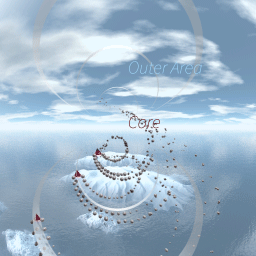' Magnetism '

This ' Antique method ' , works by the special case of a ratio to be 1 / 1 .

Using the ' Antique Approach ' might work out too
for the determination of the size of a photon ' s core :
We are going to calculate with
( 1 metre exponent ( e ) 3 ( the cubical diametre ) )
per
{
( mass of the core ( about 1 / 10 e18 kilogramms ) to the power of 4 )
times
fifth root of ( about 1 / 10 e18 kilogramms )
times
2048 th root of ( fifth root of ( about 1 / 10 e18 kilogramms ) )
...
( and so on , we will see the ' antique way ' )
...
}
What results a value similar to the way using the Classic Approach
( about ( 1 / 10 e 25 ) metres for the size of a photon ' s core ) .

...

I am still searching for a way like the Wavelength - or
Frequency - Approach to calculate size of the core , if possible .
And too , we are looking for a possibility
to see the sizes of the shell sub - quantums ,
if these own a dimensional size at all ,
may it be with negative scale too , like inverted space ...

It has been a long way to get up to the results written above :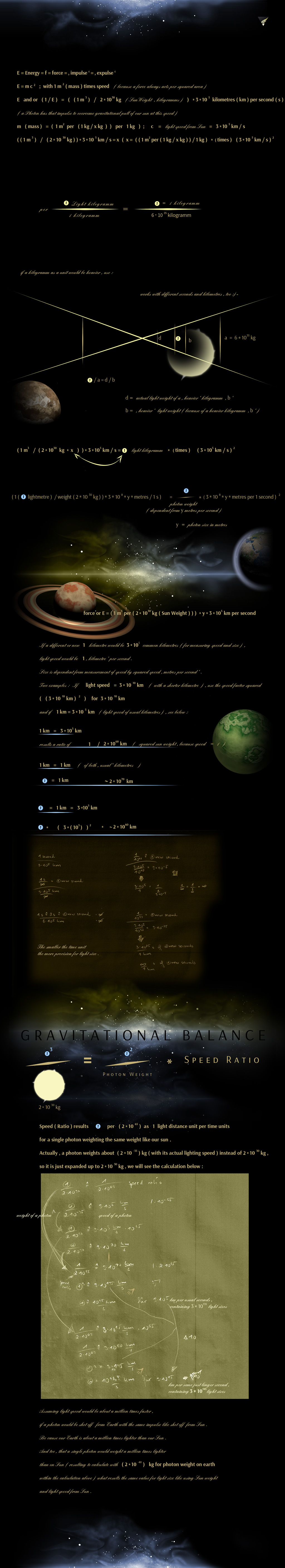1.2.1 Light Particles per cubical metre and ' Ratio ' - Approach 1

Assuming an average 'Light Particle' as a cube with lenght '1',
( 1 per Force ) to be ( 1 cubical metre per flexible weight ) times ( 1 second per flexible way ),
there should be about (( number times 10 to the power of number ) to the power of 3 )
Light particles per 1 cubical metre .

It is a rough calculation related to the units meter, second and kilogramm ,
using given Light Speed ( 3 times 10 to the power of 8 metres per second ) ,
Absolute Density of Ratio '1' as Ratio '2', Mass of Sun ( 2 times 10 to the power of 30 kilogramms ) ,
Ratio '1' as 'Lightsize per Lightweight', and Ratio '3'

If a Light Particle would have a weight of 0 Kilogramms,
it would move with a Speed of ' 1 ' within a scale from 0 to 1 .
And each mass should have its relative weight .

Note 1:
Using the term 'Mass' as kilogramm with one kilogramm as a unit to measure Gravitation
and 'Density of Mass' as 1 cubical metre per a variable size of kilogramm .

Note 2:
This calculation has led to an assumed number which is rounded roughly.
Shown by the graphics below :)

Note 3:
Be the cause
a Sun Light Particle needs exactly that impulse to leave gravitation of Sun,
similar to air particles to be bound to gravitation of Earth .

Note 4:
' Impulse making Particles slowing down to the power of two or even to the ' multiplication of Π ' in general '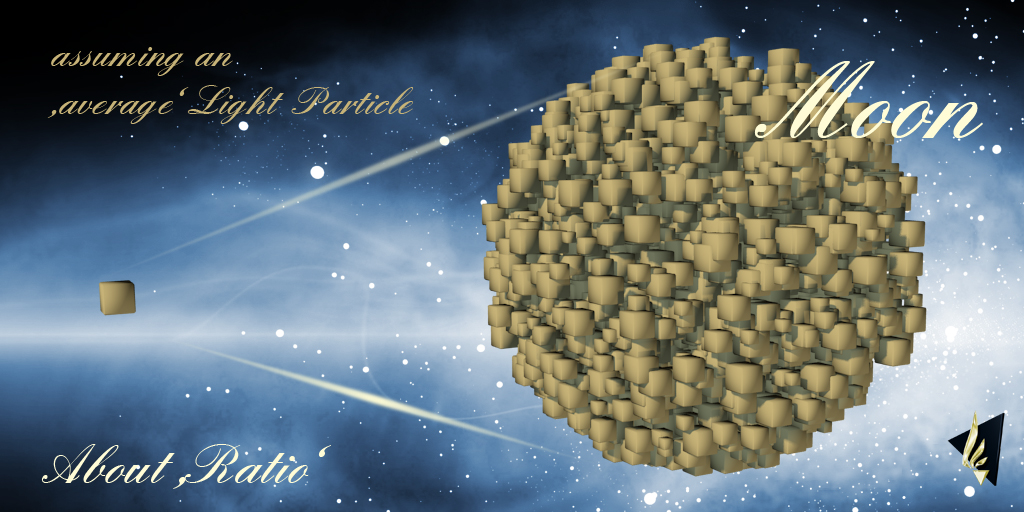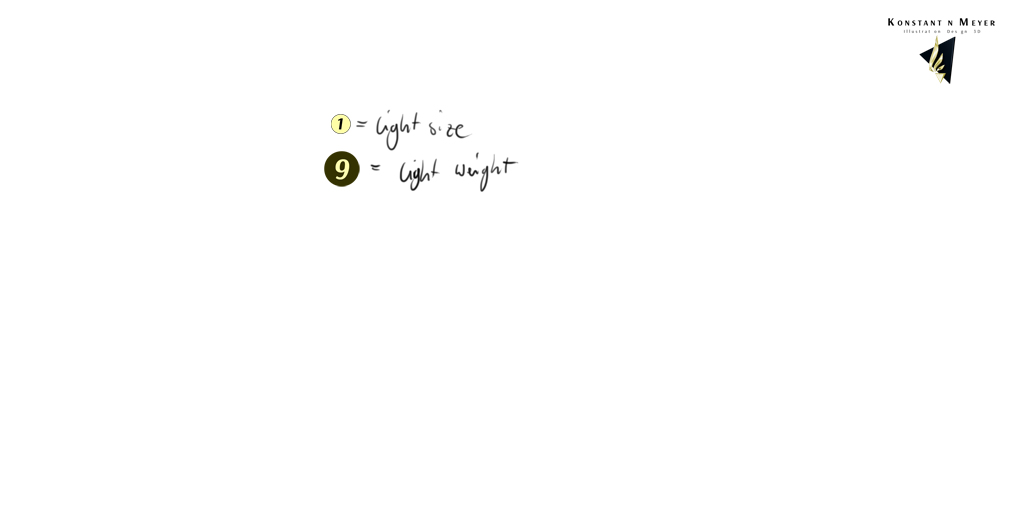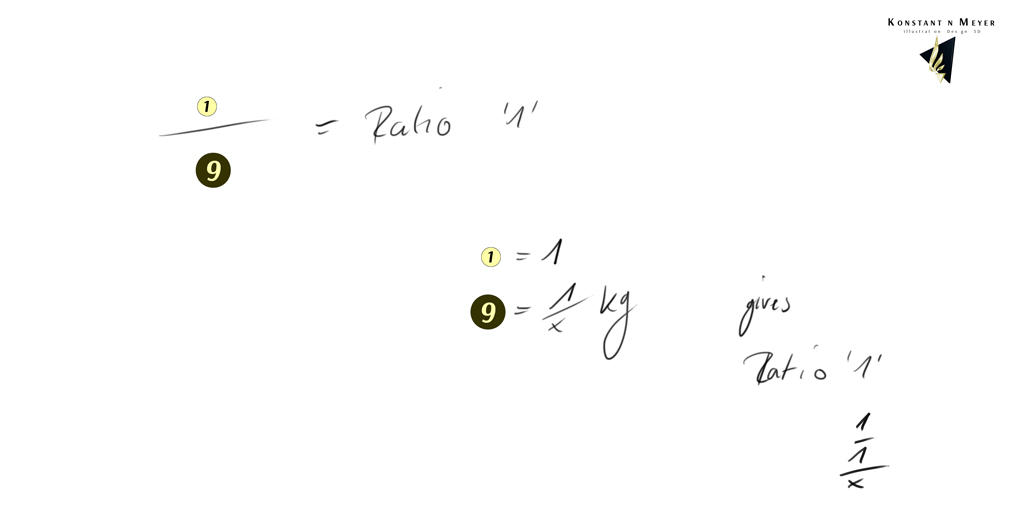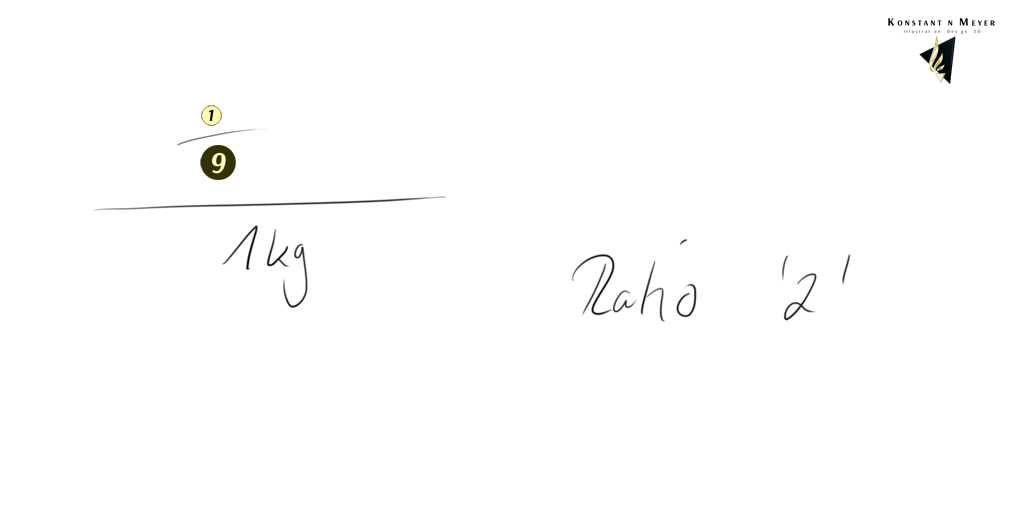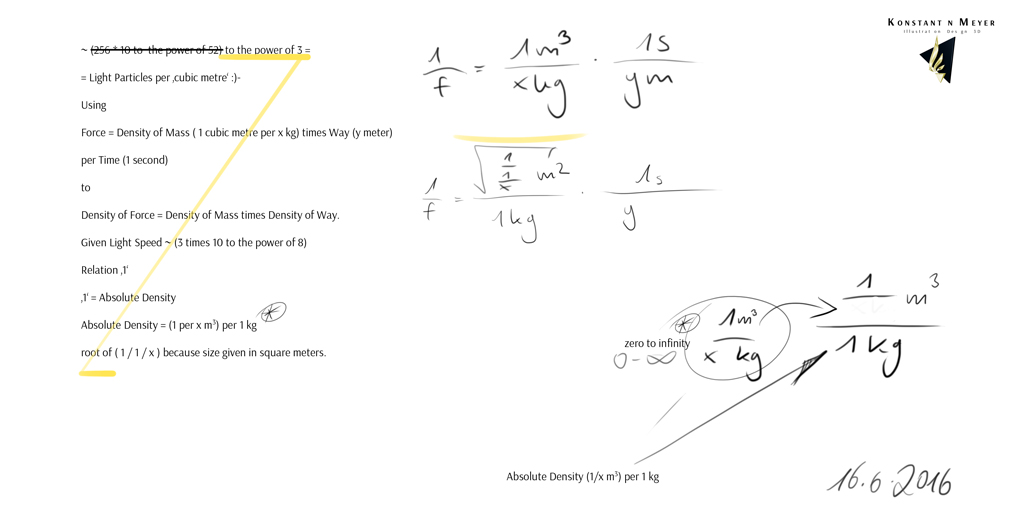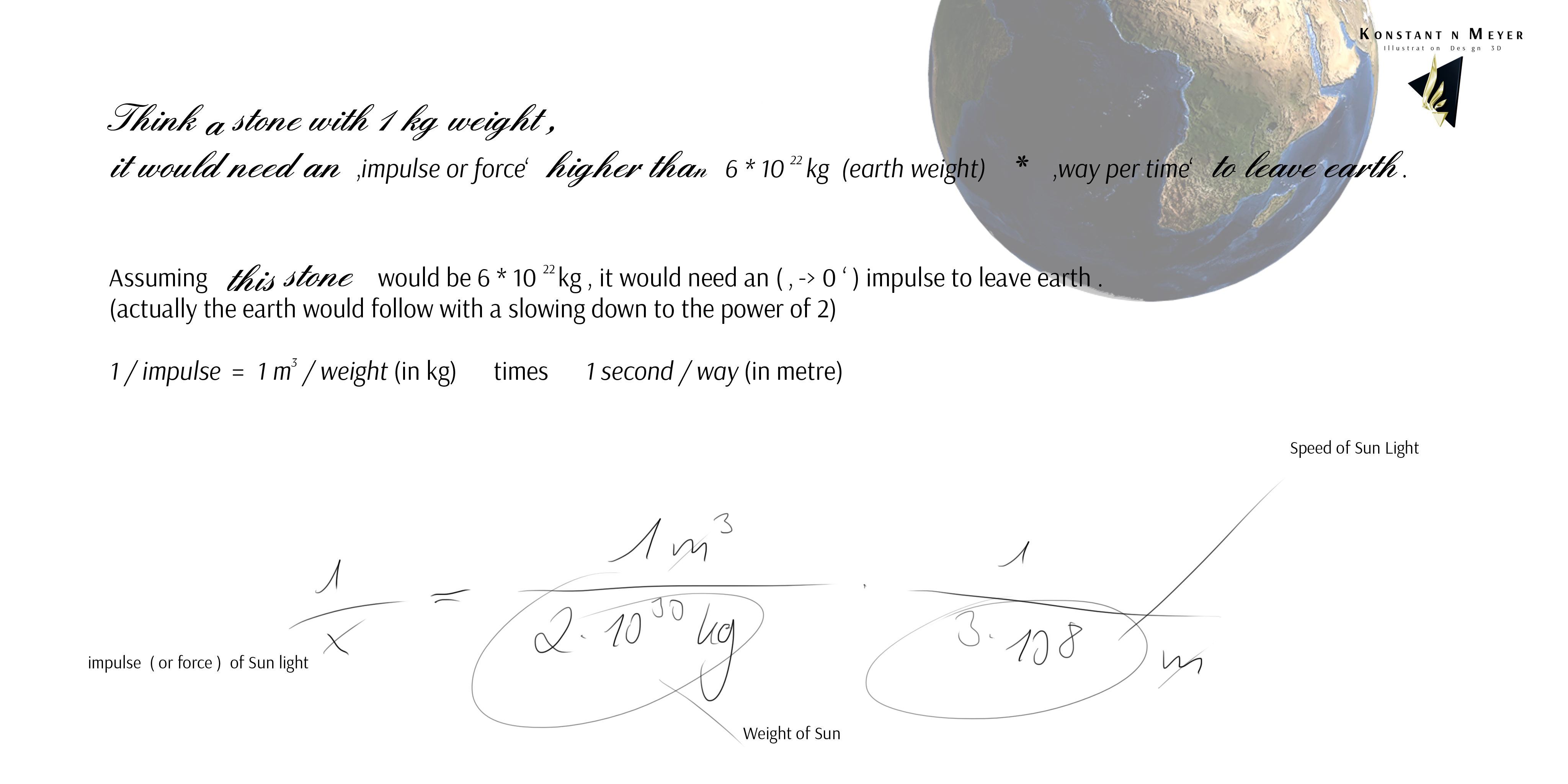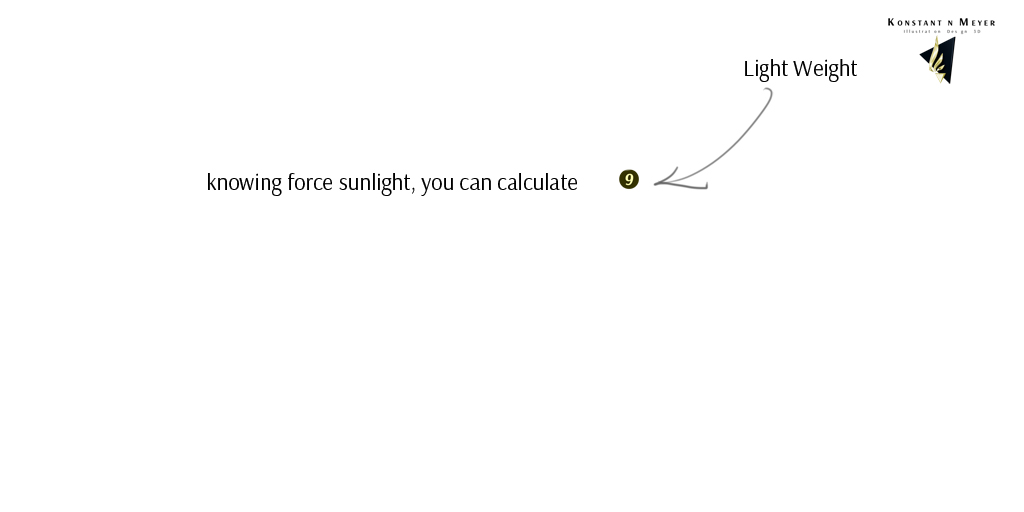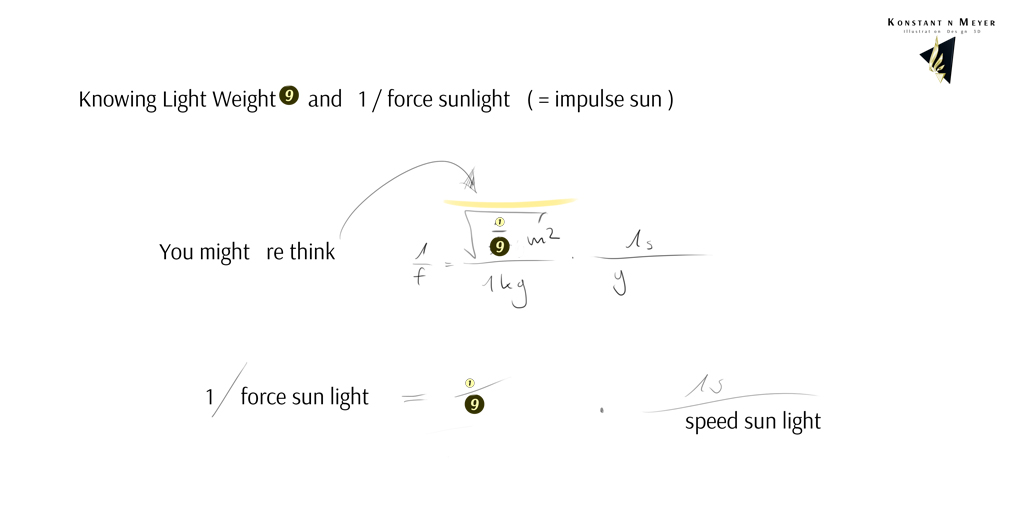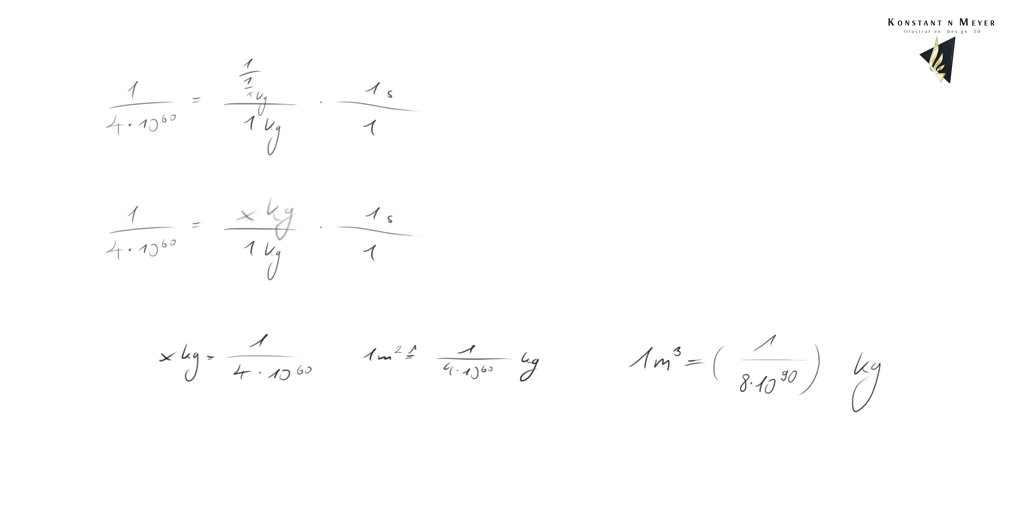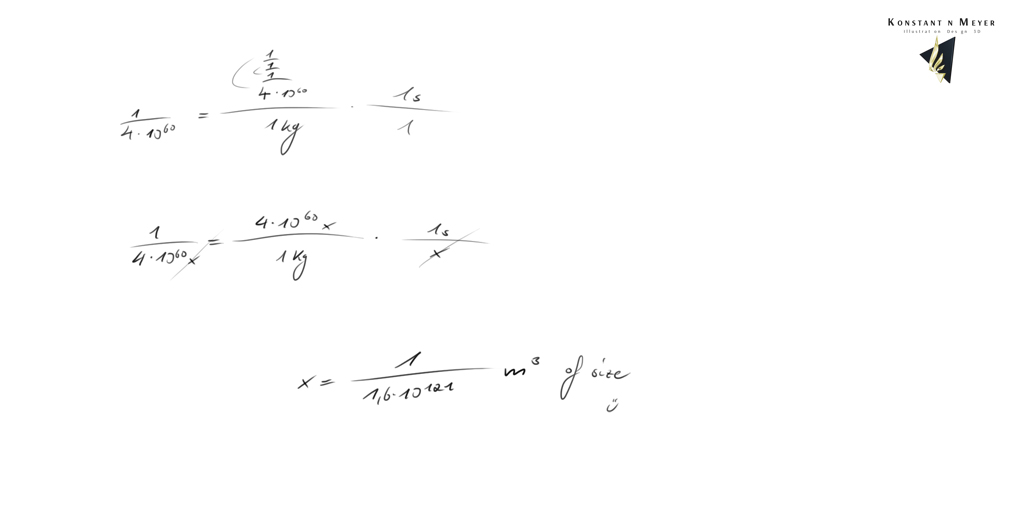1.2.1.1 Light Particles per cubical metre and ' Ratio ' - Approach 1 . 1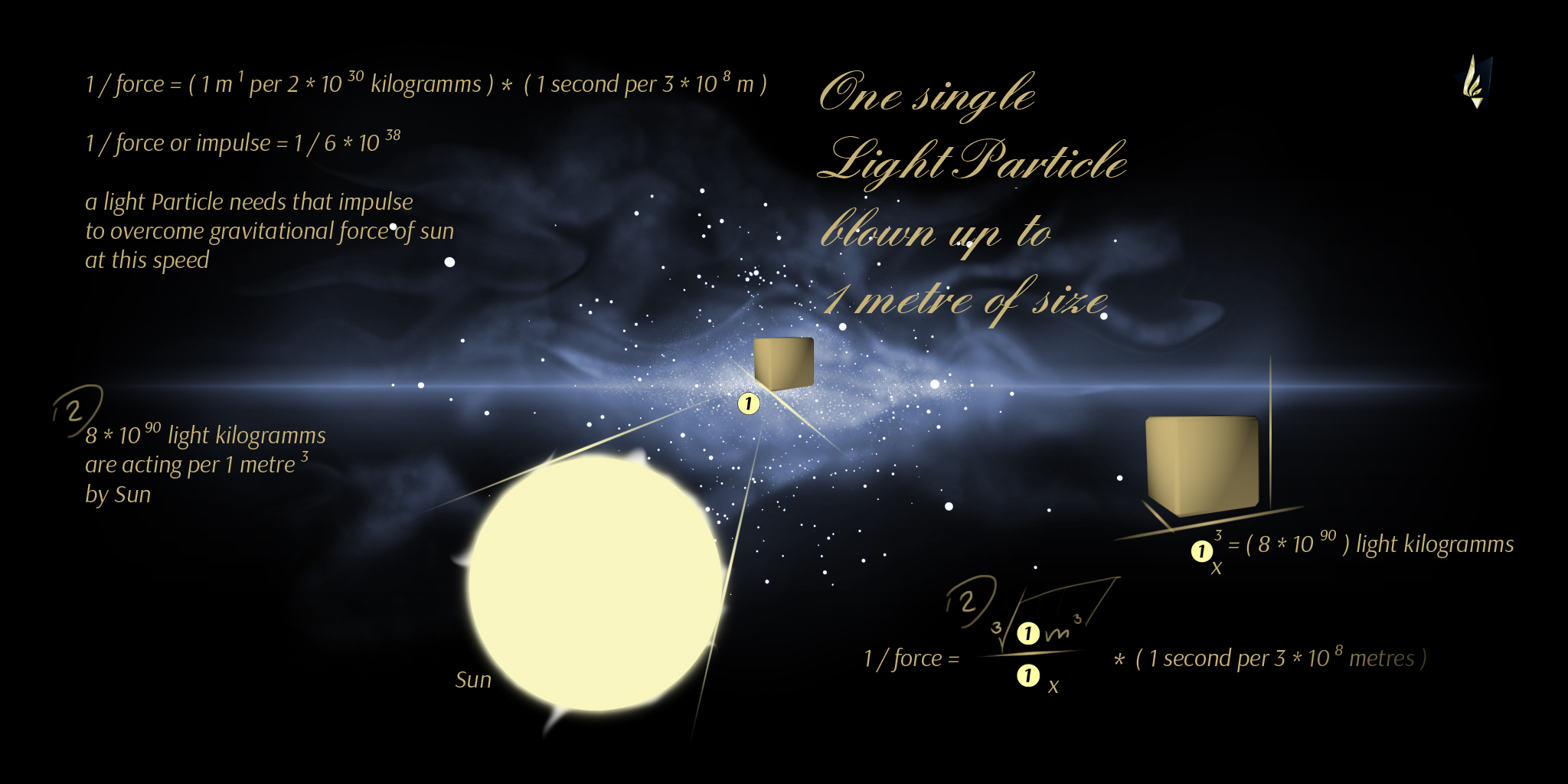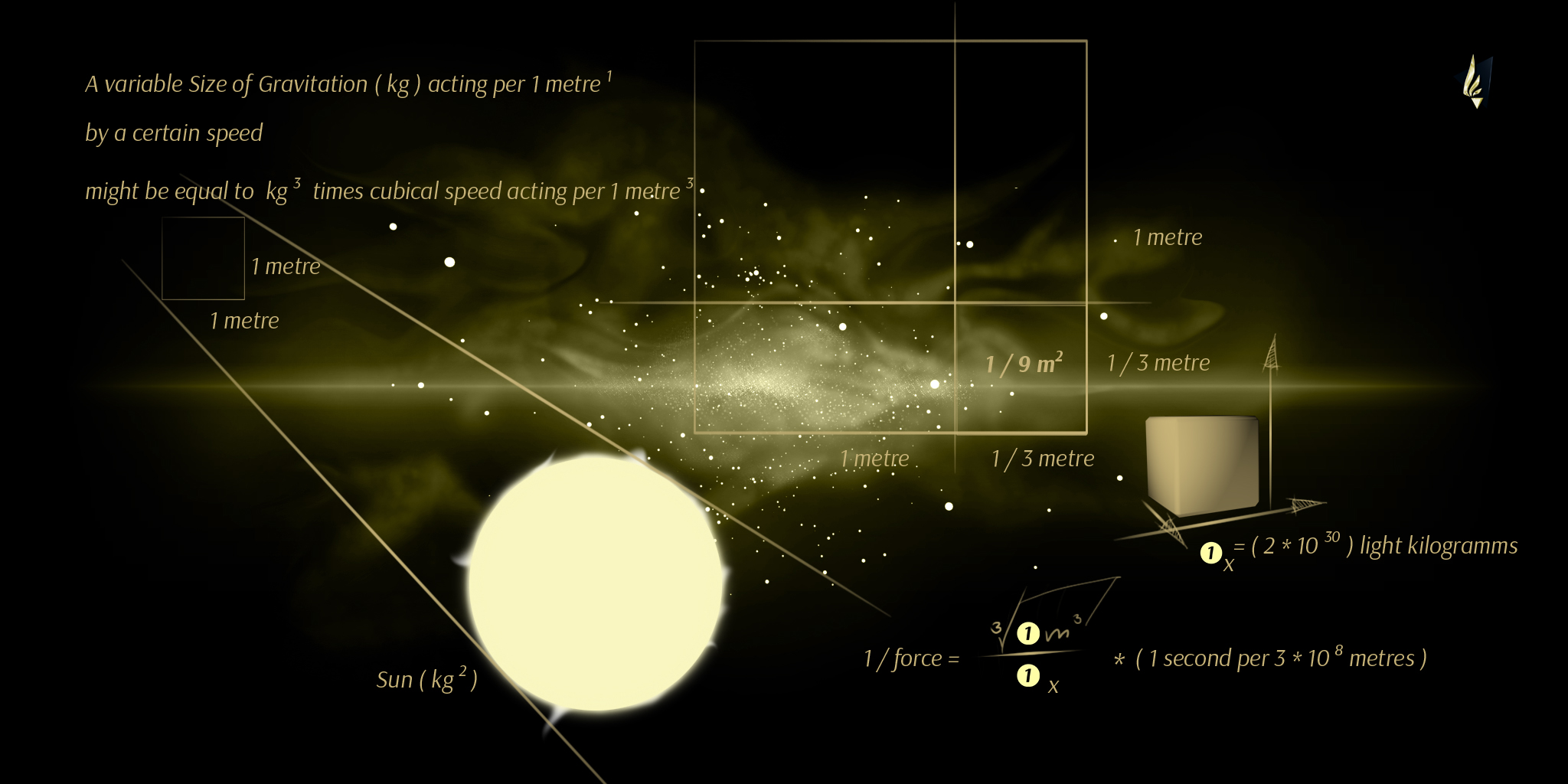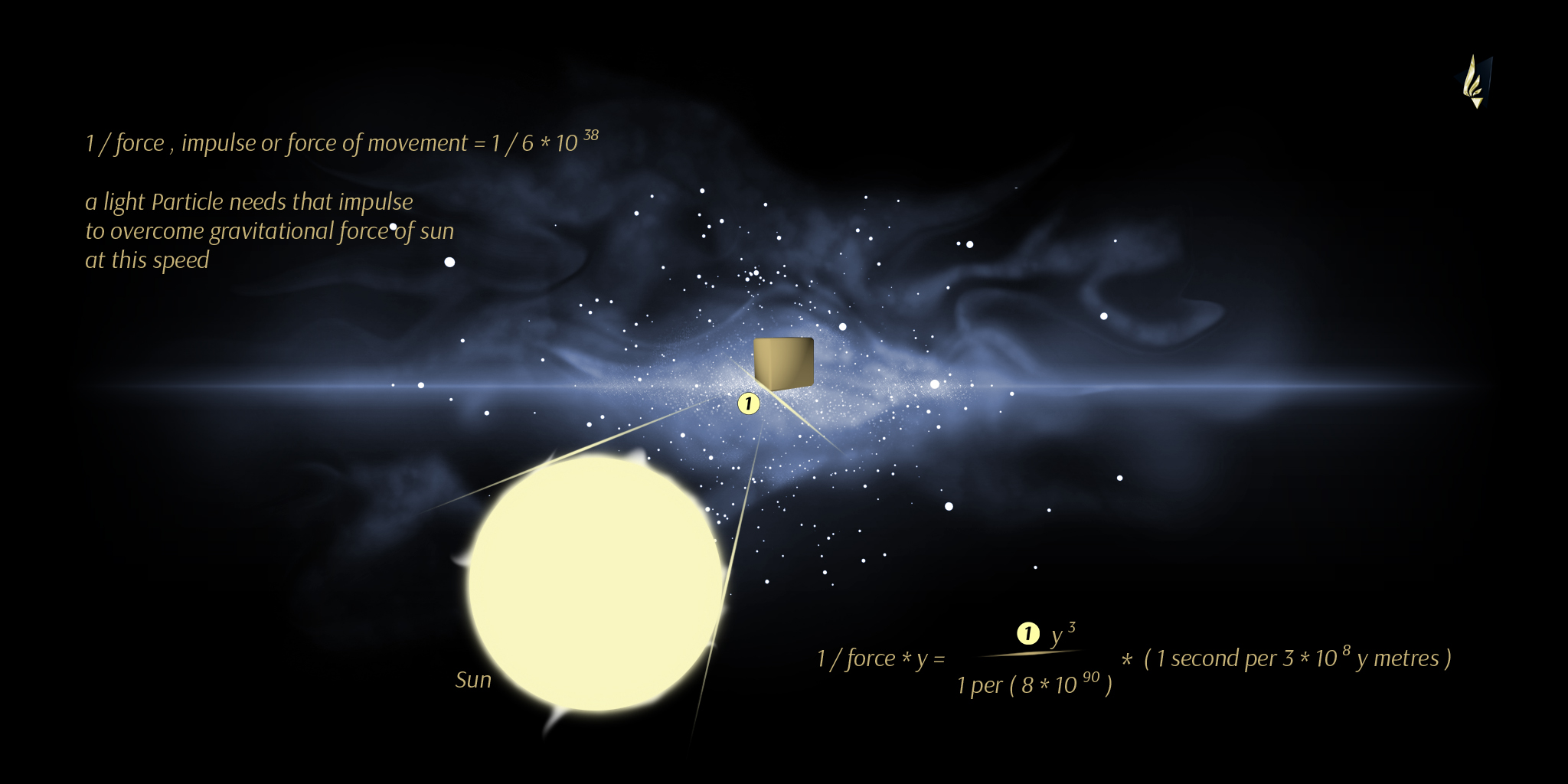Approach 1.1 resulting 1 per ( 8 times 10 to the power of 90 ) kilogramms
per 1 cubical metre of Light
and an average Size of about 1 per ( 10 to the power of 40 ) ‚a' lenght of a cube
( the cubical root of about 1 per ( 10 to the power of 120 ) metres )

1.2.2 Light Particles per cubical metre and ' Ratio ' - Approach 2

The whole Approach 2 has led our idea to calculate with :
( Sun weight ) times ( light speed – from Sun – to the power of 3 ) is equal to
( Earth weight ) times ( ( fleeing Velocity of Earth times ( temperature of our Sun ) ) to the power of 3 ) .
What results an average temperature of a value about a million degrees celsius on our Sun , heat meaning particle movement ...

A new idea ( and it should to be the right one :) leads us to use ( 1 per Sun weight ) times ( light speed squared ) is equal to
( 1 per Earth Weight ) times ( fleeing velocity , vertically , of Earth squared ) times temperature of our Sun
because E = Temperature = m c c and an object would have to overcome more force to leave a celestial body vertically ,
our result is something about 400 kilometres per second of an object to speed up to for leaving
gravitational pull of earth vertically , using Sun Corona with a temperature of something between one and two million Kelvin ...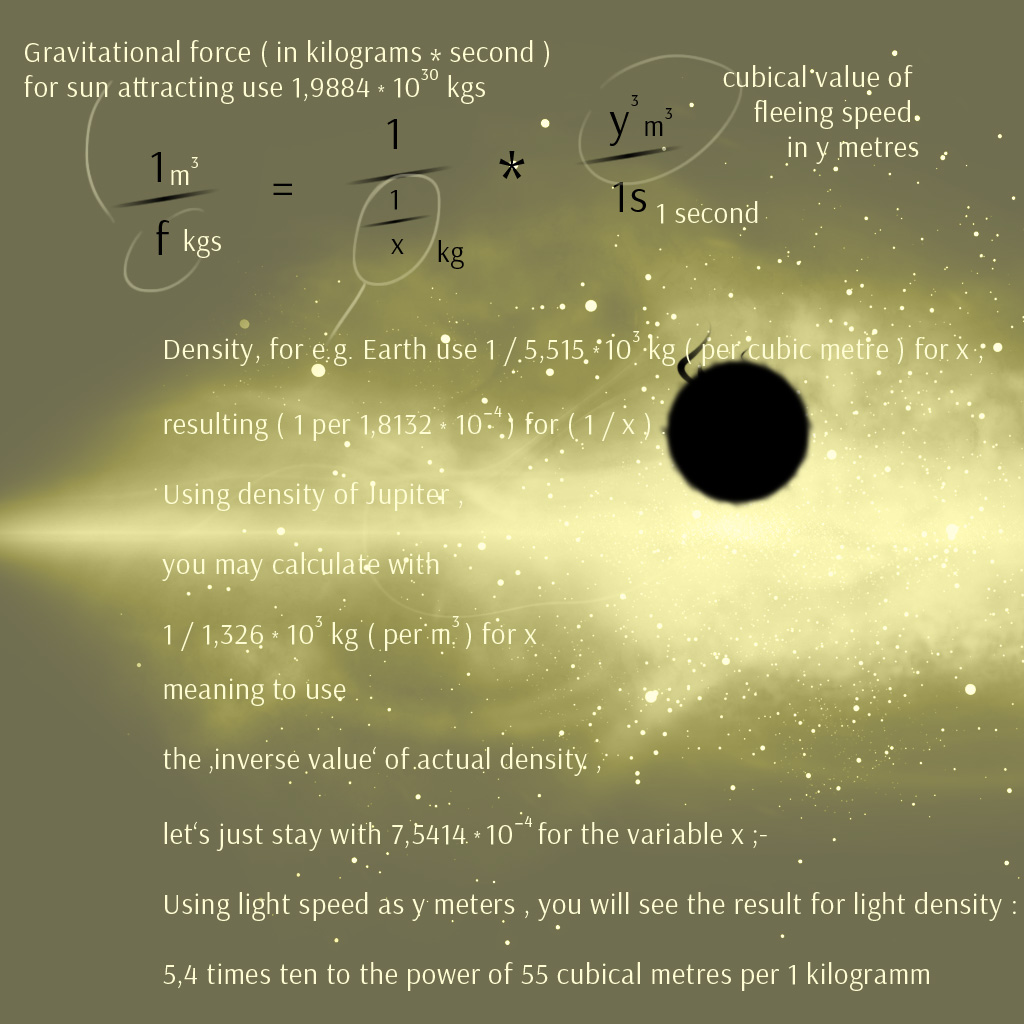Light Density Calculation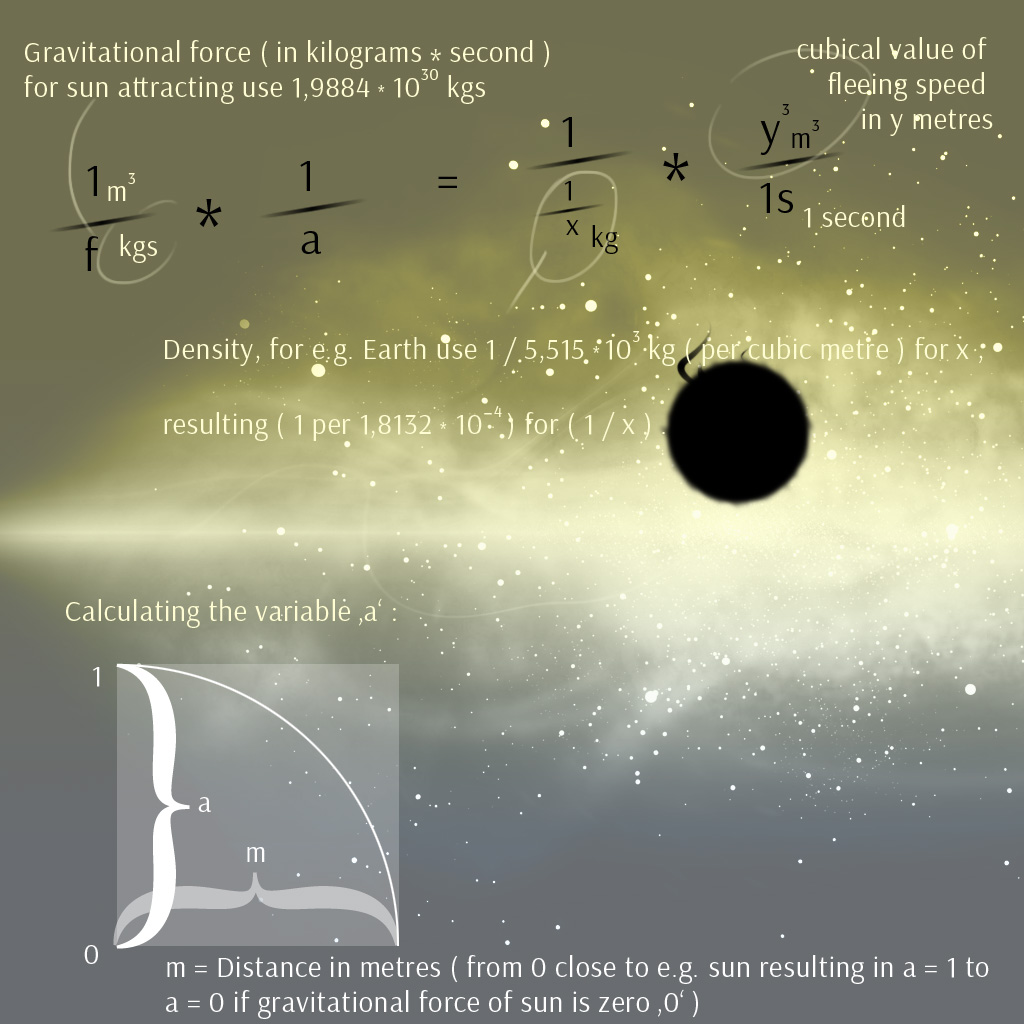Calculating with Gravitational Force, for light you might use a = 1 because close to sun

I found some ' magical ' 1 metre per 1 second what might be the fleeing Speed of our Sun
from the core of our Milky Way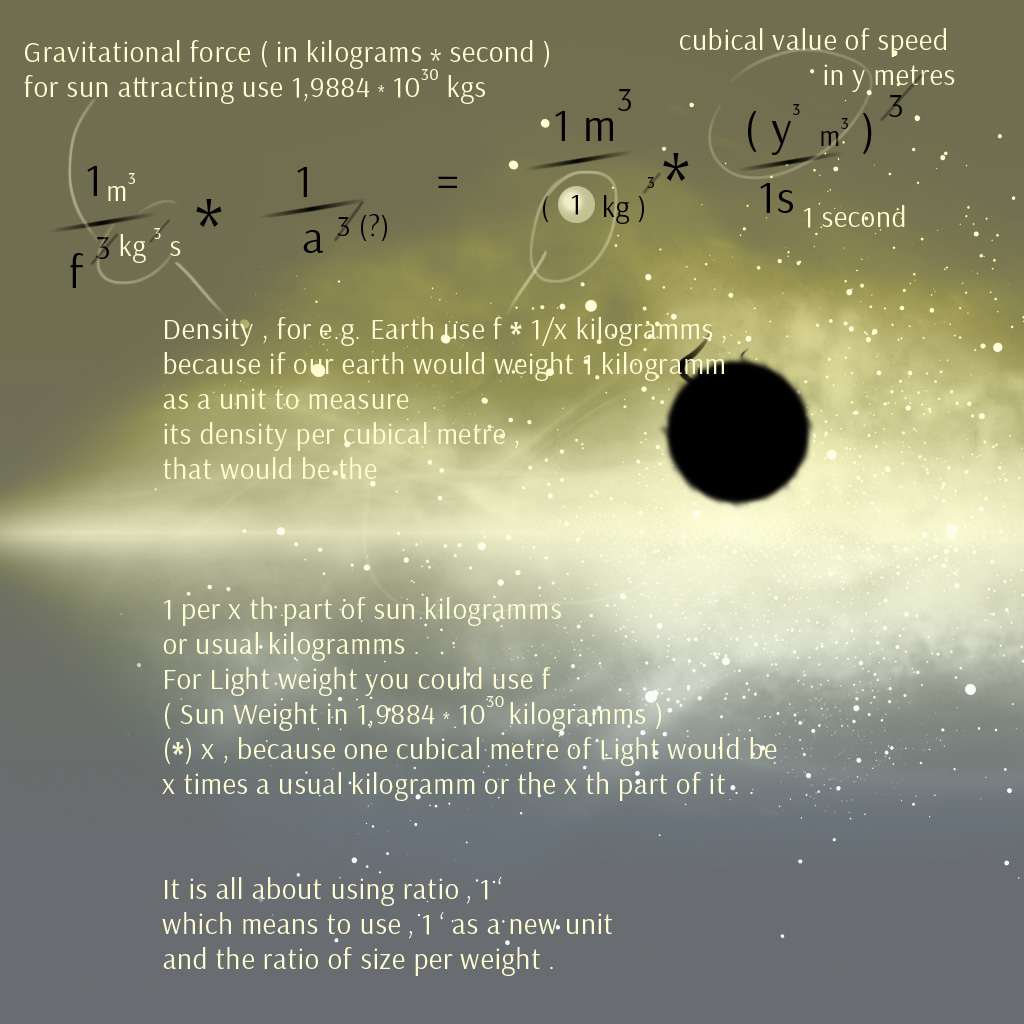Using 1 light-kilogramm per one light-metre , which is the very first idea from 2016
and cubical value of kilogramms of an object effecting one cubical metre of another object

Calculating with density of Jupiter would show
that Jupiter might leave Sun orbit about 2 cm a year, using a = 1 .

What is slower than the fleeing speed of Earth from Sun
despite of a smaller density
what would end up in an assumption of planets , that are attracted
by core of Milky Way rather by a star , if once have left gravitational influence of this star .

Note 5:
To get 'f' you might use ' 1 per force = density of mass times (*) density of speed ,
( (1/1/x per 1 kg) * 1 / (3 * 10 to the power of 8) ) '
by using x as light weight and density of average speed of sunlight
instead of calculating with using gravitational weight of sun (in kilogramms)
times speed how shown before by the upper graphics , what was I thought that days -
resulting to calculate with ( density of ) force , ( 1 / f ) and 'ratio 1' ( 1 / 1/x ) .

Note 6:
Or you just go ahead with the good old Isaac Newton
for ' force ' so you will use ' f = maa '
within the calculations described above ,
simply set his ' f ' in the formula with the squared root ( yellow highlighted ) .
Meaning light weight for mass ( 1/x ) , using its speed squared
as ' f ' in order to go with density of force ' f ' = density of mass times density of speed .

Note 7:
You may use speed ( of light ) squared , it is allowed ,
because you might think about unfolding an ' a lenght ' squared area
to get the point pressure a force is acting on by its speed .

This result has led my calculation to something
close to a trillionst part of a kilogramm ( 1 per squared speed )
for the weight of one cubical metre of light ,
using the calculation mentioned by note 6 and described above .
And if you will look through a microscope some day ,
seeing the little craters by ' light particle impacts '
you can check for the size if right or no ;) .

Note 8:
The closest approach to get the weight of one cubical metre of light I have found , is using just
1 cubical metre per Gravitational Pull of Sun in kilogramm seconds ( 2 times 10 to the power of 30 ) =
1 / 1/x ( 1 per light weight , or let us just say ' size per size per weight ' , what would result weight ) times
Speed in metres per second ( of Sunlight, 3 times 10 to the power of 8 ) to the power of 3 ,
see the graphic below .

Note 9:
The final result now for calculating the weight of one cubical metre of Light
should be to use
1 per ( Gravitational Pull of Sun ( 2 times 10 to the power of 30 ) in kg (*) times 1 second )
= equal to
( 1 per ( 1 / (1/x) ) ) as light density (*) times
cubical value of speed ( of Sunlight 2,7 times 10 to the power of 25 cubical meters per second ) .
What keeps gravitation which should to be measured in kilogramms in balance and
regarding unfolding an 'a' length cube
to get the point pressure a force is acting on by its speed , and

be the cause

a Sun Light Particle needs exactly that impulse to overcome gravitation of Sun,
similar to air particles to be bound to gravitational force of Earth .
A stone moving off earth with 3 meters per second
should have a ' gravitational weight ' of 1 per ( 3,6 times 10 to the power of 25 ) kilogramms
and its impulse ( ! should there be a need for an impulse ? )
to keep calculating with gravitational balance , no matter its size .

( see the graphic with the dark sun , shown below )

The Gravitational Force or Pull of Sun is more powerful the closer to Sun ,
and Light particles might react ( or ' being reflected ' ) faster
from Earth because the Earth has less of gravitational force ( measurement in kilogramms ) than sun .

It's the 21th of November 2018 today and I will continue from time
to time with thinking past about the offered ways to calculate ,
but I strongly think that it is done now :) ? !

Note 10 ( old ) :
' Number ay caramba , the way I pray to stay ' -
' Die Zahl zur Qual , der Weg der Steg '
The result should be the ( 5,4 times ten to the power of 55 )th part
of a one kilogramm for light weight per cubical metre
*** and *** the size of an average light particle, taken by 'ratio 1' :) ,
might unlikely (?) be the cubical root of 1 per ( 2,916 times 10 to the power of 111 ) metres
' a lenght ' of a cube .
Because of using ' ratio 1 ' to be 1 ( light size ) per ( 1 / light weight ) -
and ratio super emotio ;)-

A size of 1 cubical metre of light ,
it would have the 1 per ( 5,4 times 10 to the power of 55 ) part of a one kilogramm of weight .

Or let's just stay with a one kilogramm of light should measure about
5,4 times 10 to the power of 55 cubical meters ;)-

' Größe zu Gewicht wie ( 5,4 mal 10 hoch 55 ) zu 1 '
' Size per Weight is equal to ( 5,4 times 10 to the power of 55 ) per 1 '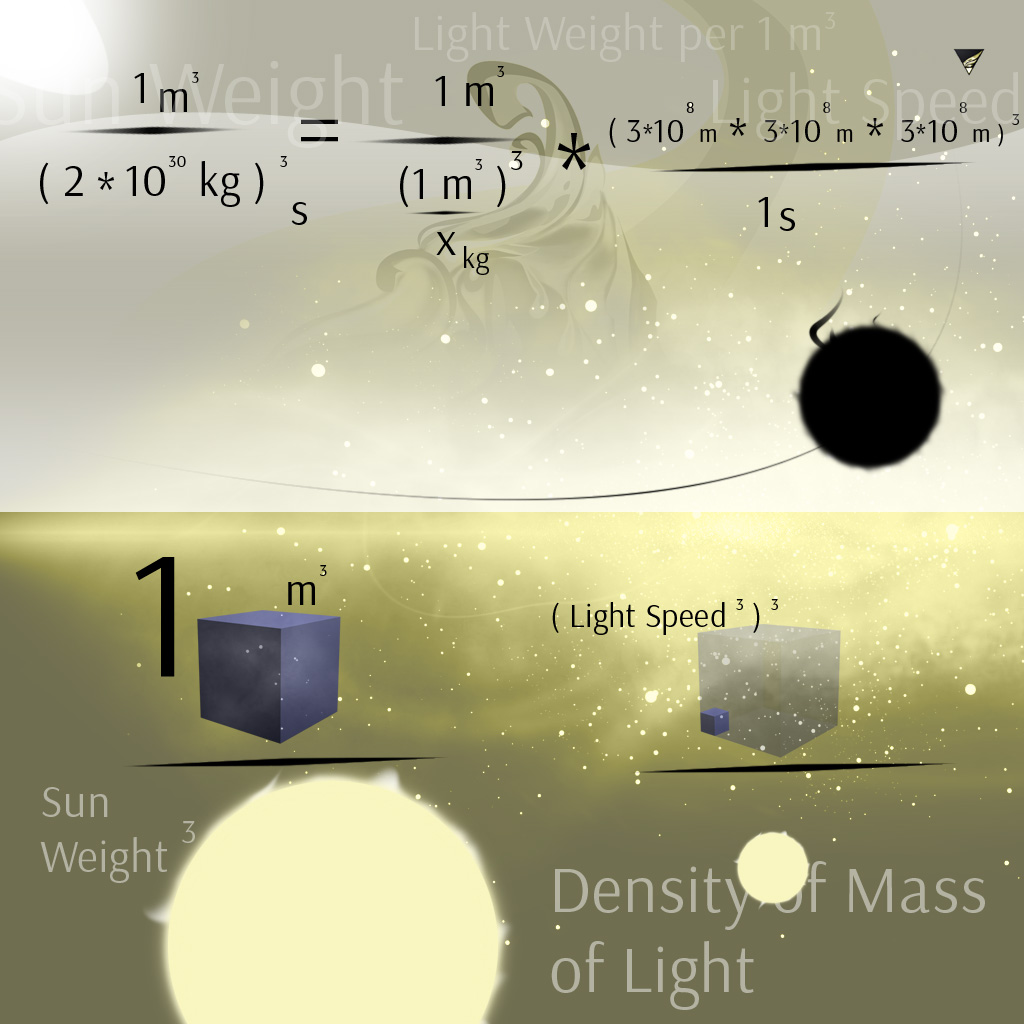Relations : Ratio '1' , and Ratio 'B' ( 1 per a variable size )

Note 11 ( new ) :
' Number ay caramba , the way I pray to stay ' -
' Die Zahl zur Qual , der Weg der Steg '
The result should be the cubical root of ( 1,6 times ten to the power of 167 )th part
of a one kilogramm for light weight per cubical metre
*** and *** the size of an average light particle, taken by 'ratio 1' :) ,
might unlikely (?) be ' the cubical root of the cubical root ' of
1 per ( 2,479 times 10 to the power of 334 ) ' a lenght ' metres of a cube .
Because of using ' ratio 1 ' to be 1 ( light size ) per ( 1 / light weight ) -
and ratio super emotio ;)-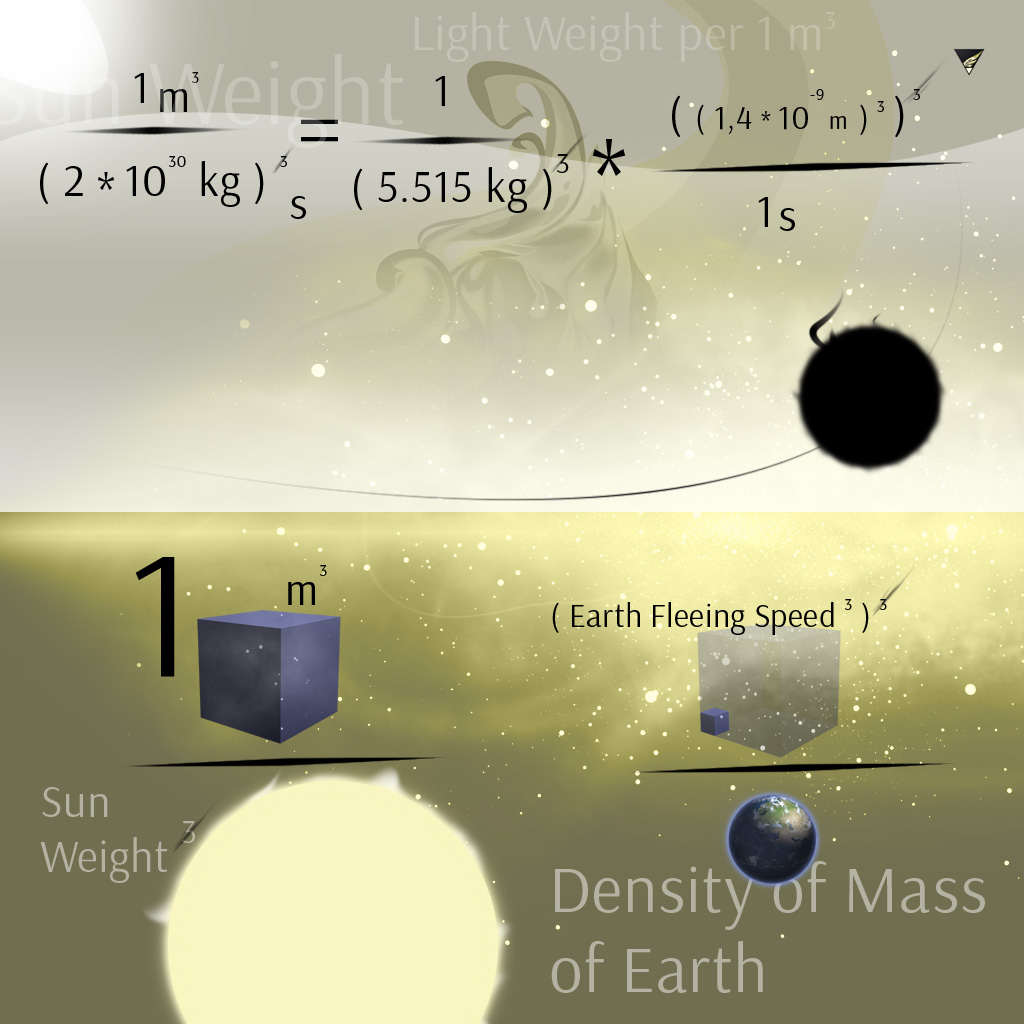An interesting thing I found , is that using Moon or Earth weight and their orbiting speed
returns results which are very close to the approach of using the calculation mentioned by Approach 4 .

Calculating with weight of Earth ( ~ partially ) and Sun ( partially, too ) and
density of Moon should result in speed Moon away from Earth.
Use Sun weight in kilogramms , because the Earth and Moon both are attracted by Sun .
Using Earth weight and density of Moon shows , that
our Moon would move about 2,5 m away from Earth during a year .
Calculating with 1 per kgs of Sun results 0,03745 metres a year of Moon leaving Earth orbit ,
which is very close to a current result of measurement .
Using 1 per Earth weight in kgs is an ' ideal sphere experiment ' with Earth and Moon isolated .
The result is regardless of other forces within our solar system or universe
and doesn't take distance and the by going loss of gravitational force into consideration .
To get a clear result you should make use of the variable 'a' ;)-

What I found too , is that ' speed squared ' per mass ( in kg ) of a moving object
might be of a fixed aspect ratio : 1 per ~lightSpeed squared ;
e.g. speed of Moon around Earth 'squared' per its weight should be about 1 per lightSpeed 'squared' ,
works with Sun and Earth also , approximately .

A later idea went me to use orbiting speed to the power of four
per weight what should result the fixed aspect ratio
of something about 1 per ten to the power of 10 .
I have tested on moon , earth and sun , and it seems
to work out without many doubts - or just limited ! ;)
Try using core of milky way , its moving speed within space
to the power of four per its weight .
Using kilogramms and metres per second .

Note :
I have been looking for a good ' all in one formula '
to describe and calculate objects within our universe ,
and some further ideas are in development ...

' Impulse making particles slowing down to the power of two '
In true , it should be the relation of a quarter circle instead of being squared , if so and if ...
And gravitational force should become less by ' a graph of a quarter circle ' , too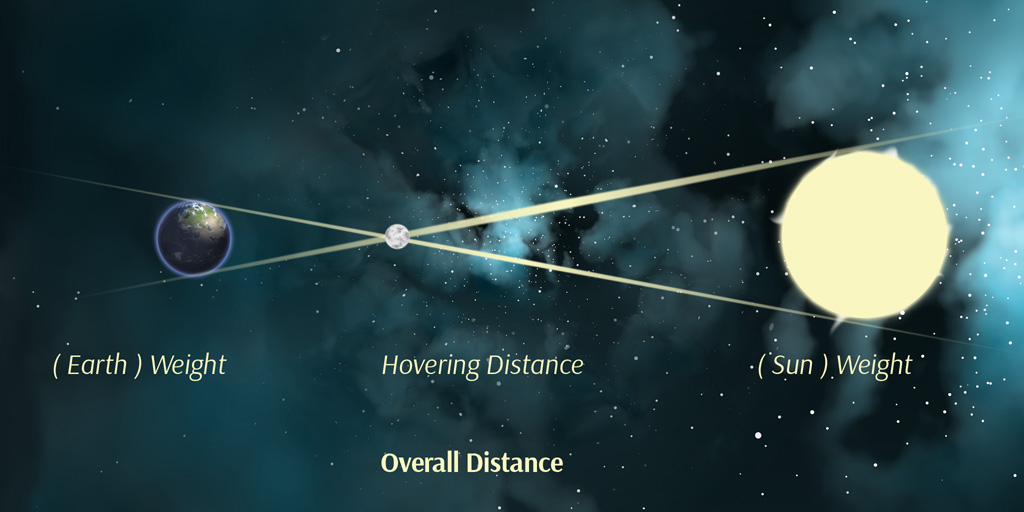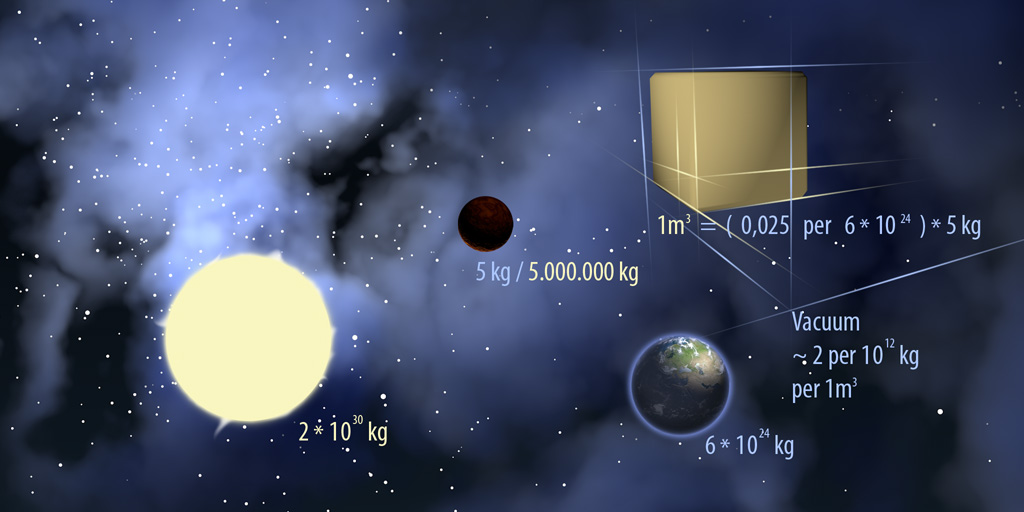A trial to prove Approach 2

Prove 1 ( within the formula )

According to the ratio used by Approach 2 , an object within space
with a weight of 5 kilogramms would require another object
to be of a density of 0,025 kilogramms per cubical metre ,
in order this another object to move away with a speed of
2 metres per second , what results 8 by its cubical value .

Weight is relative , and the 5 kilogramm space object
might to be in relation of earth ( or earth kilogramms )
what would result the calculation
' ( 0,025 kg per 6 times 10 to the power of 24 , earth weight )
times five kilogramms '
to receive the actual weight in relation to earth
of the 0,025 kg per 1 cubical metre object ,
which is the same density
required to
leave earth at a speed of 2 metres per second
using the calculation with earth weight .

This result actually is closer to the density of vacuum
( using an assumed value )
regarding the 5 kg object to be made of compressed density .
And according to Archimedes Law , things just arrange to
same ( average ) density .

The ratio used by Approach 2 returns Weight per Second
( while one Second can be very relative , too ) .
Surely , the pyramides have been sunken ' a bit '
since the first corner stone was set ? ;)-
And Jupiter might become an ' Earth II ' one day
when it finally has compressed by gravitational force :)

Prove 2 ( external prove )

Think a stone with 1 kilogramm of Weight ,
( and to be about 1 cubical metre of size or just infinitely small ) ,
it would need an impulse or force
higher than 6 times 10 to the power of 24 ( weight of Earth )
times (*) cubical speed
to overcome gravitational force of Earth .

1 kg ^= Earth Weight times cubical Speed

For light, it might be the same :

1 / x / 1 times ( 1 second per ( 1 kg times 1 cubical metre ) , ( light weight ) )
^=
1 second per ( Sun Weight times cubical Light Speed )
( in order to leave gravitational force of Sun )

what results 1 cubical metre of Light to be about
1 per ( 5,4 times 10 to the power of 55 ) kg of weight per second

The temperature of our Sun is about 10 to the power of 6 degrees Celsius
( a value rounded very roughly and used by an assumed average )

That means the impulse of Sun light to be that times stronger
than on Earth because you can use an average temperature of about
1 degree Celsius on our Earth .
For , let's just say shooting a little stone
( or light ball ) from Earth ,
we would need an impulse of 1 per one million because
our Earth is that times lighter than our Sun .
As Earth is something of about a million times lighter than Sun ,
and about a million times colder ,
light would need a 1 per one million impulse to leave Earth
which could be provided by electricity ,
and if our Earth would be of the same temperature like Sun ,
light would leave at a million times faster speed , assuming .

Another thing , which would be in harmony with Prove 1 too ,
is to use Earth weight in kilogramms instead of Sun Weight ,
to calculate Light Weight per cubical metre .
Because if we regard Prove 1 , we would need to divide
the Light Weight of 1 per 5,4 times 10 to the power 55 kilogramms
per 1 cubical metre
by earth weight firstly ,
and then to multiply with Sun Weight ,
because we think and measure Sun Weight in relation to
earth weight and Earth Kilogramms
( like shown by the graphic above and by Prove 1 ) .
Regarding a 1 kilogramm stone on Earth might weight
something of about a million kilogramms on Sun be the Cause
Sun is a million times heavier than Earth , too .

That would result a Weight of something about
1 per ( 1,6 times ten to the power of 50 ) kilogramms of Light Weight
in relation to Earth , using the Ratio and
what would be a million times heavier than
calculating with total Sun Weight ,
and our Sun is about a million times hotter .

We think the 1 kg stone to leave Earth again :

1 kg from Earth ^= 1 per ( 5,4 times 10 to the power of 55 ) kg light Weight
per second , leaving Sun
Impulse on Sun for Light is 10 to the power of 6 times stronger than on Earth ,
surely we are talking about heat , meaning Particle Movement

The 1 kg stone might need an impulse of 6 times ten to the power of 24 ( earth weight )
times
a speed of x to the power of 3 to leave Earth , which should be equal to the light impulse ,
( 5,4 times 10 to the power of 55 ) and we might regard the temperature delta of earth and sun which is something about 10 to the power of 6
in comparison to weight delta which should to be the same approximately or even exactly :)-

What should result a speed of something about
10 kilometres per second this 1 kg stone needs to be speeded up
in order to leave earth ;
which is a result very close to the speed Isaac Newton
has proven .
Claimed as common ( and assuming ) light to act with same speed more or less ,
but to mind the weight of the space object it leaves , like e.g. earth or sun ,
the calculation should provide a solid value to deal and to work with
if using it for e.g. the calculation a rocket needs to be speeded up to leave our earth .

You are using cubical value of Speed in metres to the power of 3
because of
calculating with e.g. Sun weight to be compressed within 1 cubical metre .

We might see the calculation below ...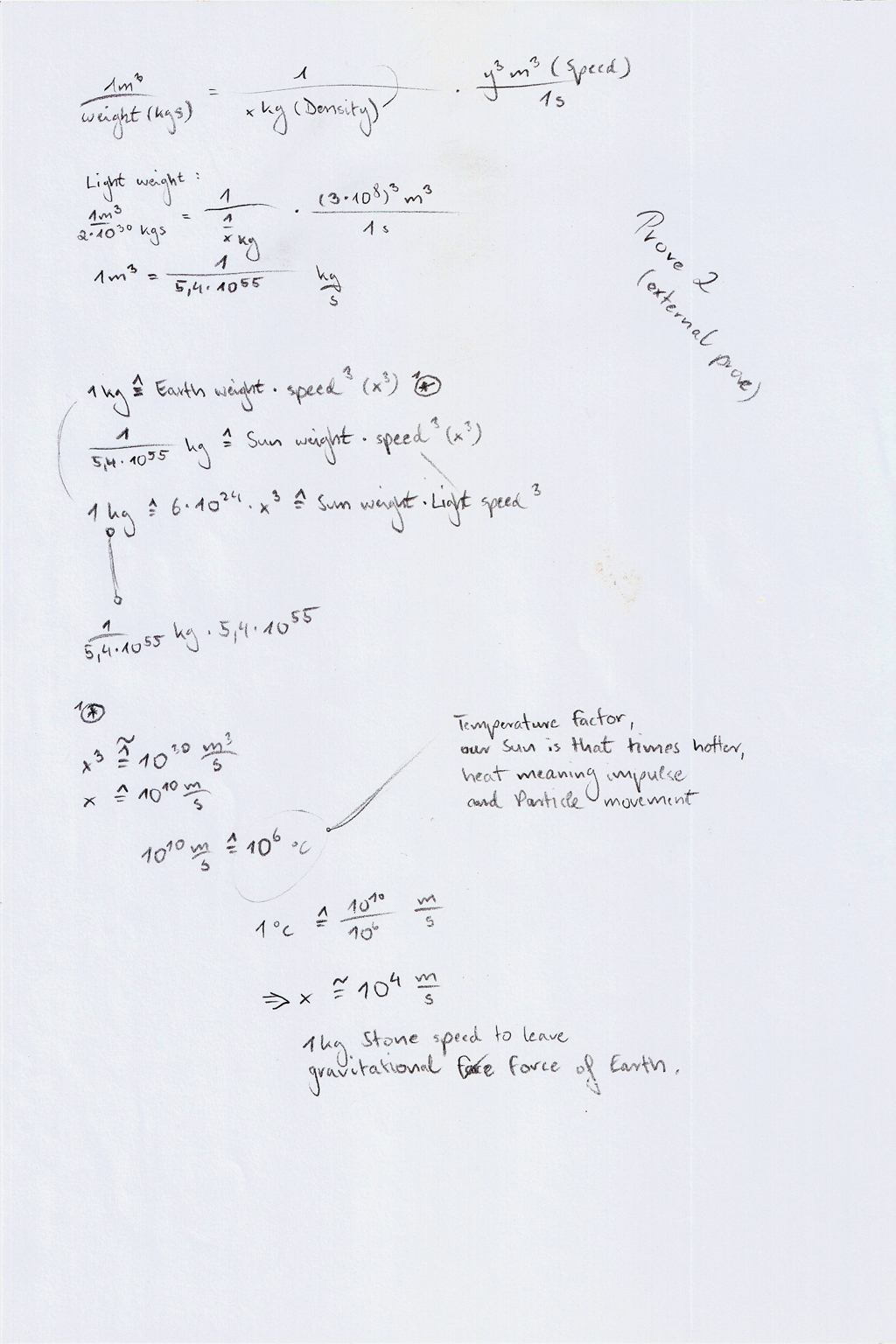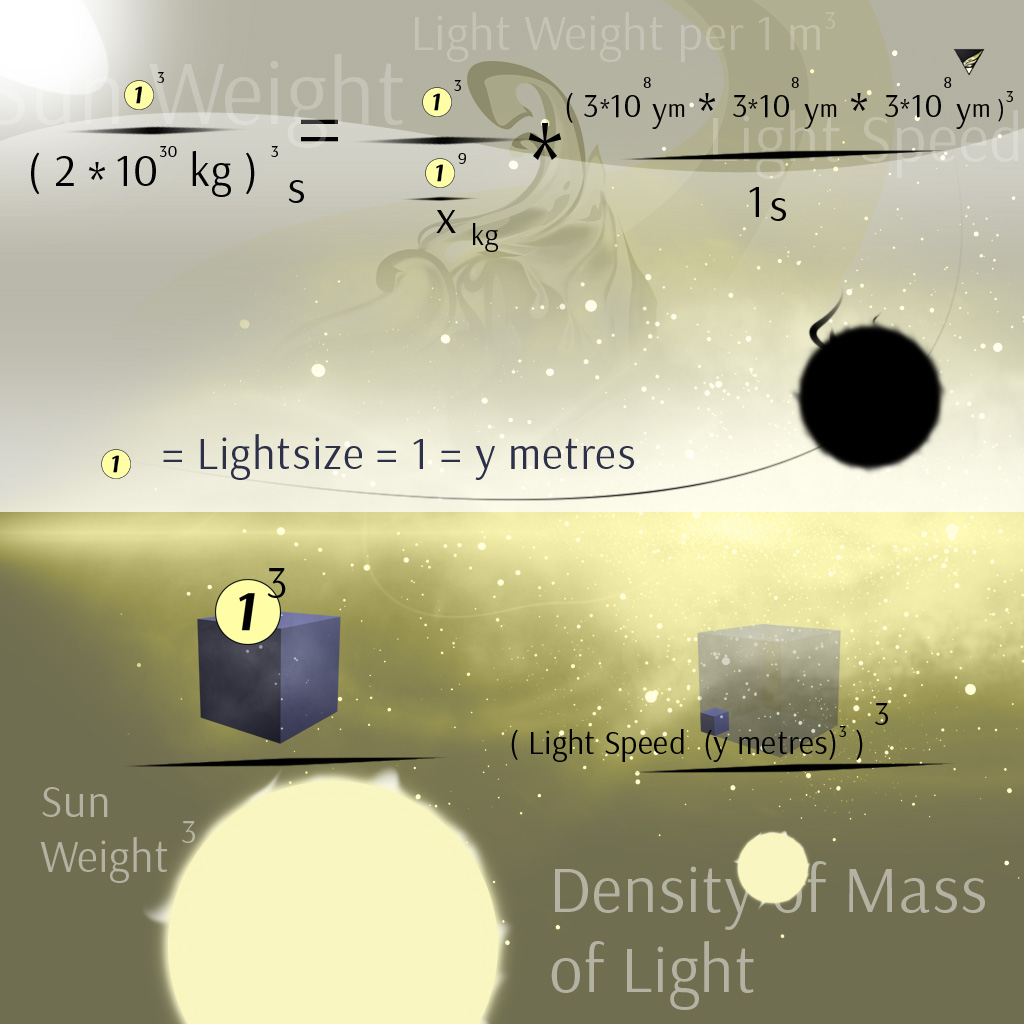Using Ratio '1' meaning 1 for light size as a unit , this are 'y' meters .

Approach 2 resulting 1 per (5,4 times 10 to the power of 55 ) kilogramms per
1 cubical metre of Light
and an average Size of about 1 per ( 10 to the power of 37 ) ‚a' lenght of a cube
( the cubical root of about 1 per ( 10 to the power of 111 ) metres )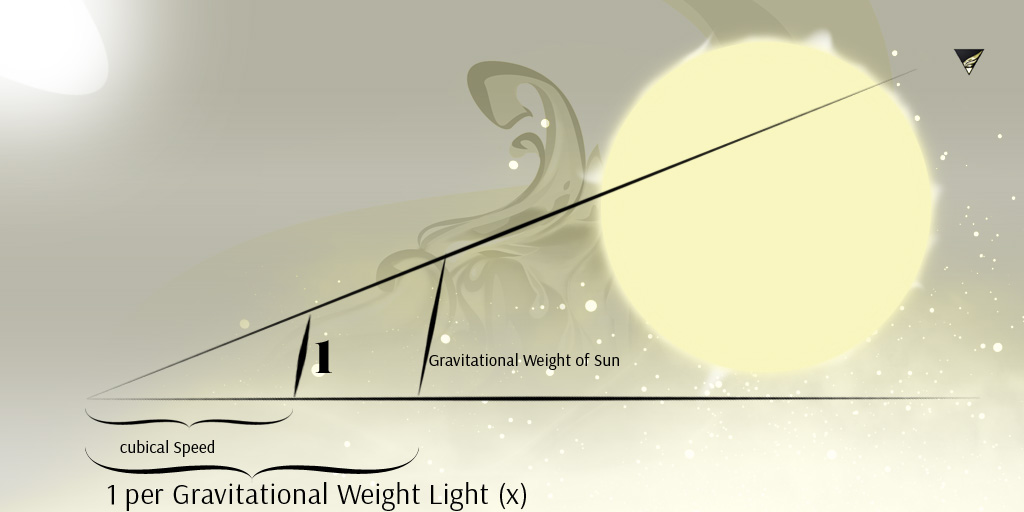Graphical Solution of the Calculation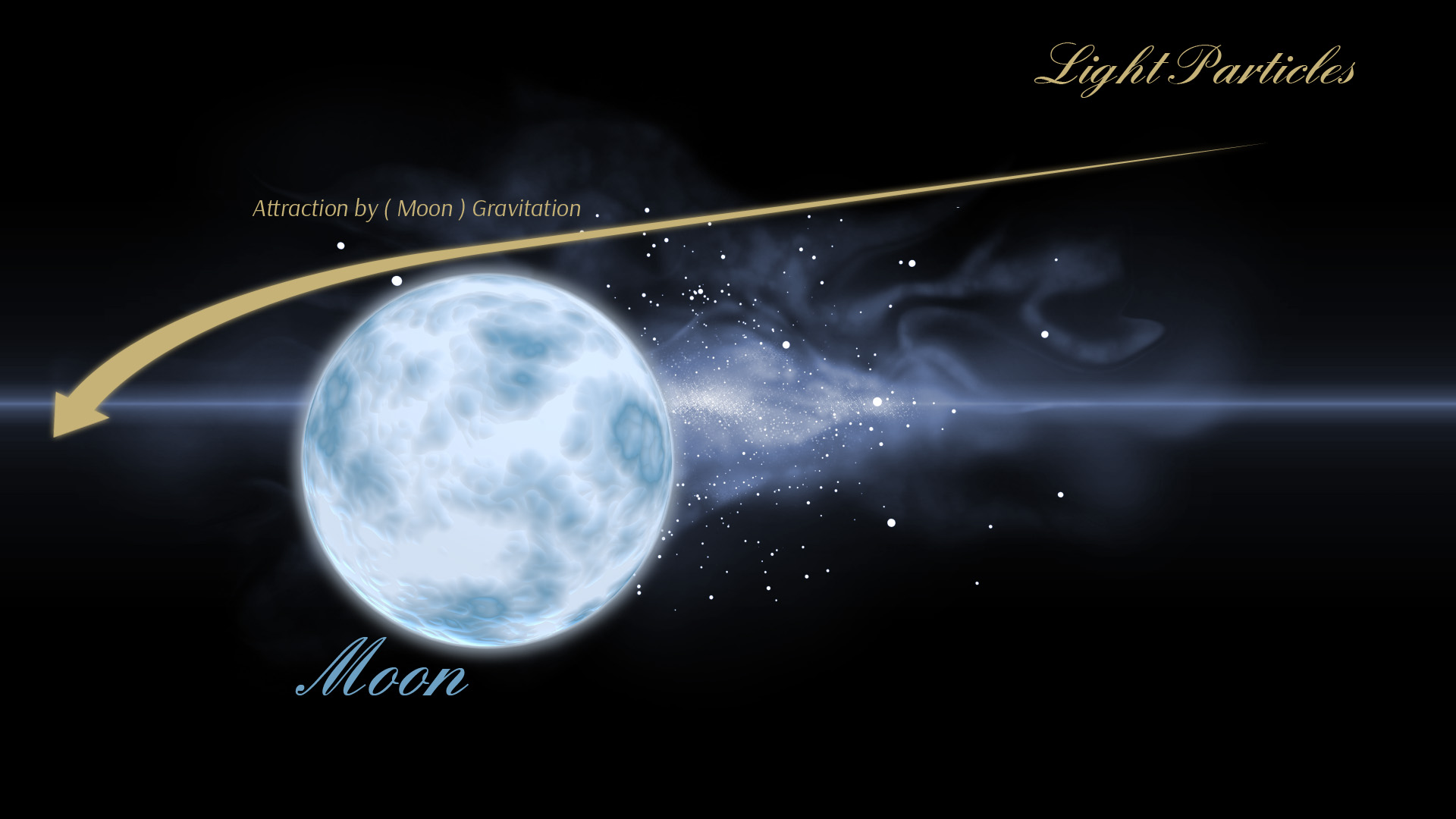Light Particle Attraction by Gravitational Force of e.g. Moon , Earth Satellite

An average white Light Particle sent from Earth by an impulse should be slower
and of shorter distance than sent from Moon with the same impulse .
Light should obey physics like any other object .
Just think about a Light Particle to be the same size like Earth or Moon in relation to infinity :)

The upper graphic would show a nice way to calculate the weight
of an assumed average white Light Particle
by knowing force and all other characteristics of a ' stone ' or ' iron ' ball
shot from the near area of e.g. Moon or Earth etc. while comparing that data
by measured Light Particle attraction of a ' light ray ' attracted by an object like Moon etc .
like the experiment of the thrown Paper Jet , see below ;)-A short story ...

I have been living near a train station .
While I was watching the tree behind a locomotive and its smoke stack
the tree seemed to flicker and to blur behind the chimney .
I suddenly had the idea that the little light molecules
have been thrown out of their natural passes
and to be pushed upward a little bit ,
surely because of the heat meaning particle movement .
Then I started to think about twice such a things :
Might this be an answer to the question of
light molecules reacting like domino particles either
or just behaving like little iron balls , once shot off
reaching their targets in a straight way .
An interesting quiz , which seems to remain a riddle to me up to today .

But keeping that little secret , it told me another one :
Throwing a paper jet above a heating source ,
we all might know speed , weight and hence force , too , of this experiment .
So , if measurement of light molecule distraction above a heating source
like that one above the smoke pipe of the locomotive would be possible
by a strong objective ; we would know speed of light as well and
comparing that data with the thrown paper jet and its force and
degree of distraction , it would be an easy thing
to calculate the weight of a single light particle .

Short words , long mathematics ;)-

Paper Jet weight = 1 / 1000 kilogramms
Paper Jet speed = 4 metres per 1 second
Paper Jet Force = mass times way per time = 1 per 250 kilogramm metres
Paper Jet Distraction by the Heat Source = 1 metre upward

1 metre upward = 1 / 250 kgm

Light Particle weight = x kilogramms
Light Particle speed = 3 times ten to power of eight metres per one second
Light Particle Force = 3 times ten to power of eight metres times x kilogramms
Light Particle distraction by the Heat Source = y metres ; known

1 metre upward of the paper Jet = 'z' times upward of the Light Particle , 'z' times y metres

1 per 'z' = Light Speed times 'x' kilogramms

Nono end ;)-

1.2.3 Light Particles per cubical metre and ' Ratio ' - Approach 3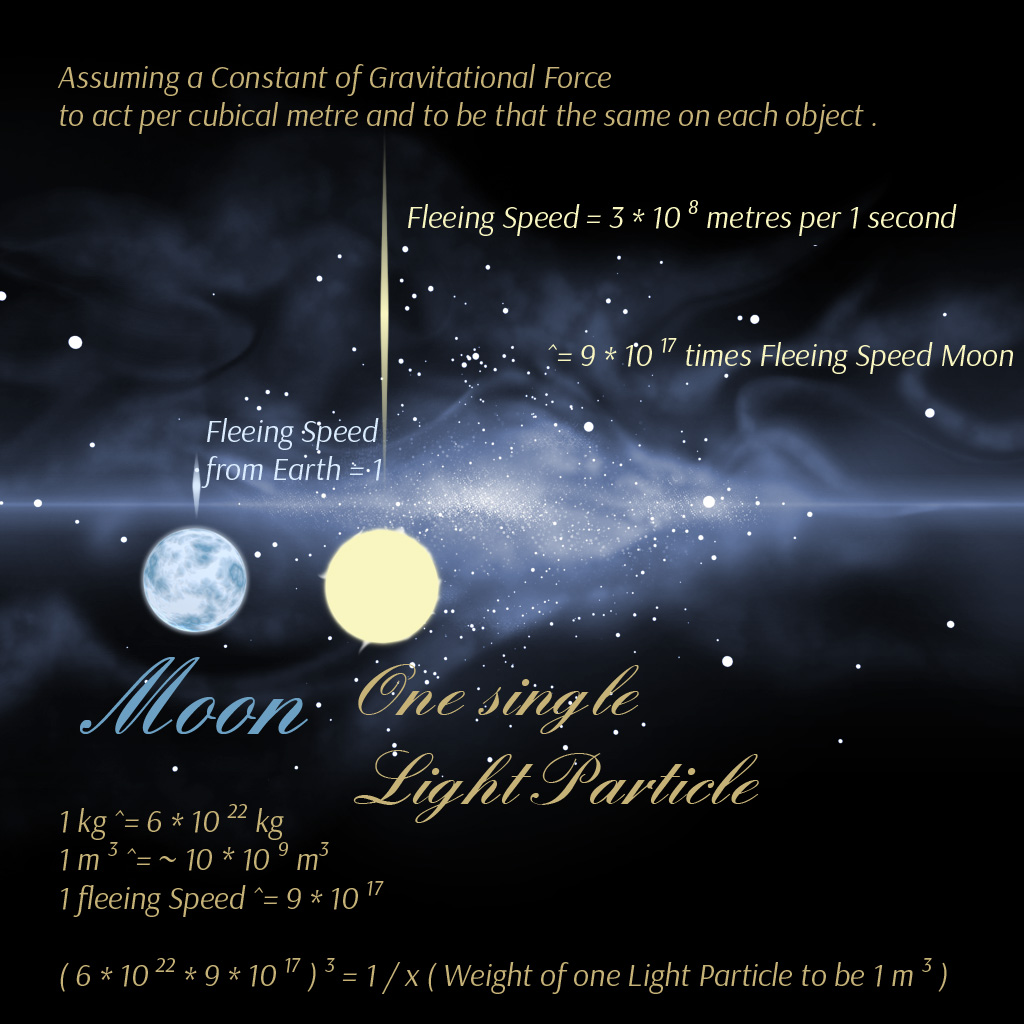A Light Particle to be same size and weight like Moon and
to be blown up to 1 kilogramm of weight and 1 metre of size

If a Light Particle would ' weight ' 1 kilogramm , and
our Moon would weight 6 times 10 to the power of 22 kilogramms ,
may it to be a Light Particle the
1 per ( 6 times 10 to the power of 22 ) th part of a one kilogramm firstly ,
and then the ( 9 times 10 to the power of 17 ) th part of that .
Considering force of a Light Particle and force of Moon to be the same ,
and cubical value of gravitational force , kilogramms to the power of 3 ,
acting per cubical metre by cubical speed .

Assuming a Constant of Gravitational Force , meaning each object
to have the same energy or to be influenced by , within our universe .
Resulting the calculation of the weight of an average light molecule
to be estimated about 1 per ( 10 to power of 120 ) kilogramms ,

and about 1 per ( 10 to the power of 37 ) metres of size
regarding our Moon measures about 10 times 10 to the power of 9 cubical metres
which is that times bigger than one cubical metre a Light Particle is set to .
What would provide a value some how in harmony with
the rework of the very first approach from the year 2016
and with the second approach of august 2018 , too .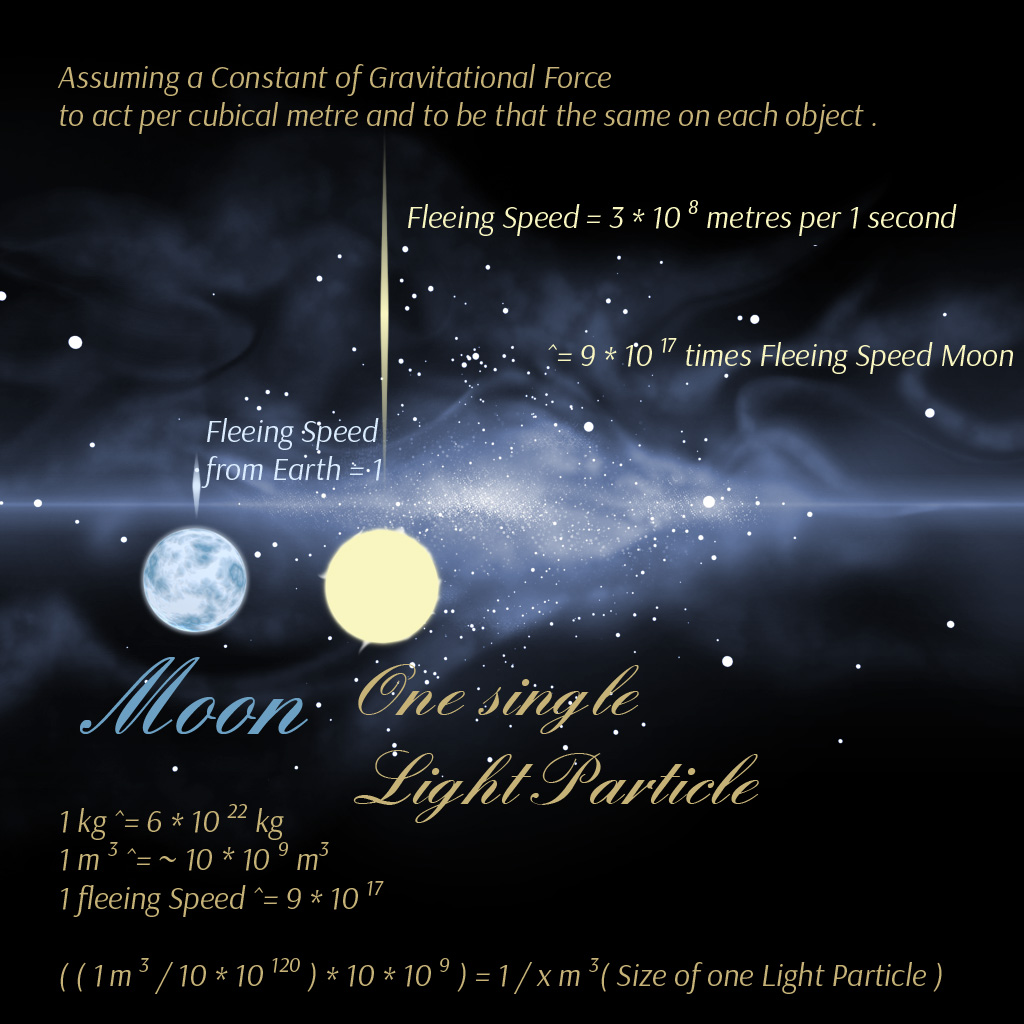Approach 3 resulting 1 per ( 10 times 10 to the power of 120 ) kilogramms
per 1 cubical metre of Light
and an average Size of about 1 per ( 10 to the power of 37 ) ‚a' lenght of a cube
( the cubical root of 1 per ( 10 to the power of 111 ) metres again )

Multiplication of the weight with Moon Size
is because of the Light Particle being blown up to all three parametres
Weight , Size and Speed
in order to achieve a balance

And using this calculation with Earth and Moon
it gives something about 4 times ten to the power of three
kilogramms per cubical metre of moon density .
Just use the Division Delta of both Earth and Moon fleeing Speed .
Division Delta means to reduce the ratio of Moon speed per Earth speed
which should to be ( 0,038 metres per year ) per ( 0,044 metres per year )
by using 0,38 per 0,44 ( just get rid of additional potences, meaning
erasing 10 to the power of -9 ( speed per second ) on both top and bottom
of the ratio to get a pure ratio , which is what we call ' Division Delta ' ) .

The whole Approach 3 is based on Delta Force of Space Objects
doesn ' t exist in relation to infinity .
And some idea about 2*2*2 = 8 and 3*3*3 = 27 ,
with a delta of either 1 or 19 ,
and too, because of assuming the cubical value of Speed acting
per cubical metre by cubical value of kilogramms like mentioned above .

1.2.4 Light Particles per cubical metre and ' Ratio ' - Approach 4 ( Mathematical error ? )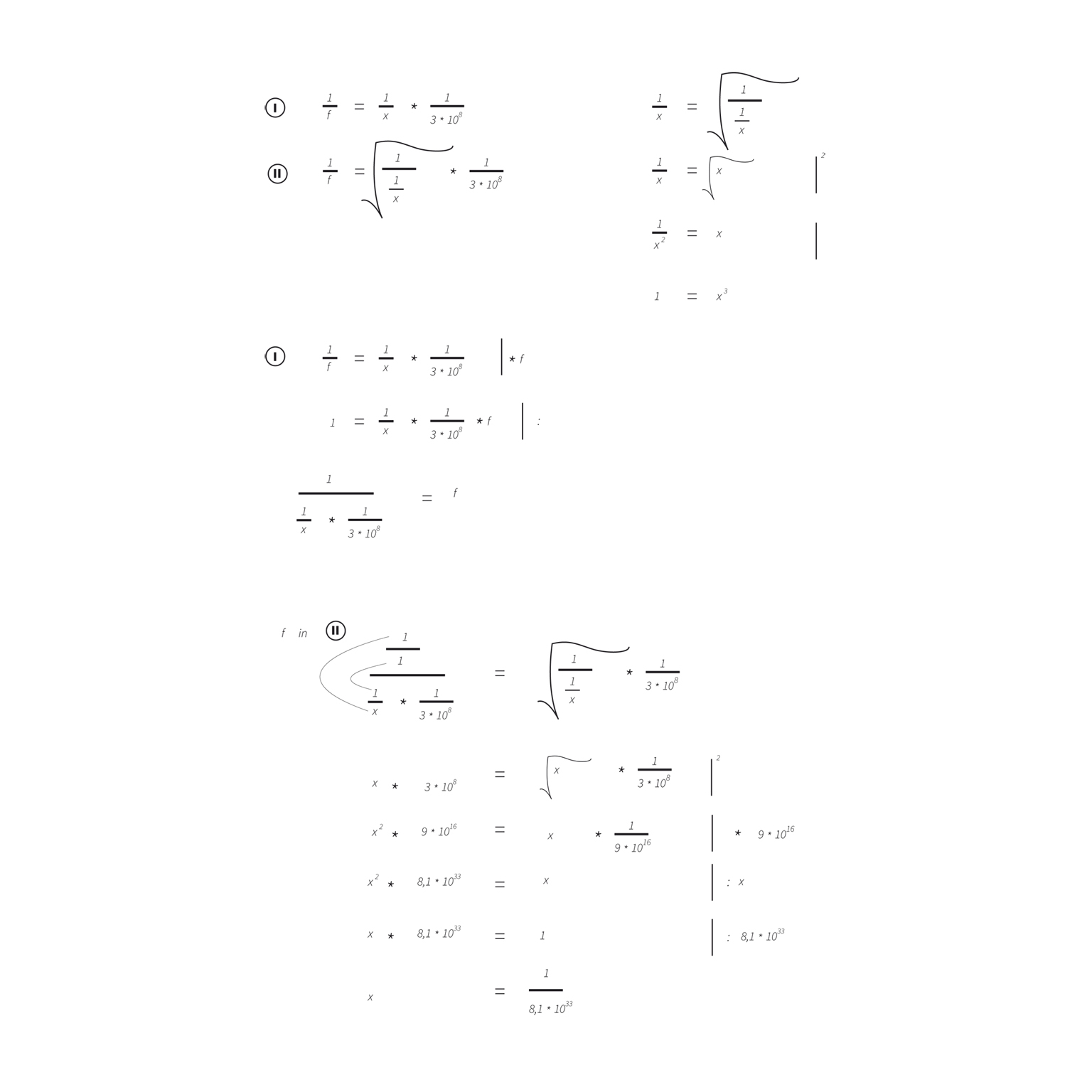Note 1
1 / f = 1 / x * 1/average Lightspeed
isolate f to :
f = 1 per ( 1 / x * 1/average Lightspeed )
and use this 'f' (force or impulse) now
within the calculation described below ,
simply set this 'f' in the formula
with the squared root , see about that above .

Calculation using both formulas in combination ,

1 / force is equal to 1 cubical metre per flexible weight ( not density of mass )
times speed ( 1 second per flexible way )
as force of movement

and

1 / force is equal to squared root of ( ( 1 cubical metre per x kilogramm ( as density of mass ) )
times speed ( 1 second per flexible way )
as gravitational force

and setting values of force equal to each other

what ended up in a mathematical error ( ? ) to receive a ratio

This has led my idea to distinguish between two main kinds of energy :
Gravitational Force beside ( and resulting in ) Force of Movement .
You might use speed squared , too .
A candy I can still show .
At the end , it is the relativity of weight which is causing clutter :)-
as well as the first three approaches result at least similar values .
It might be the cause light speed is used by its average , too ,
which could be very relative instead .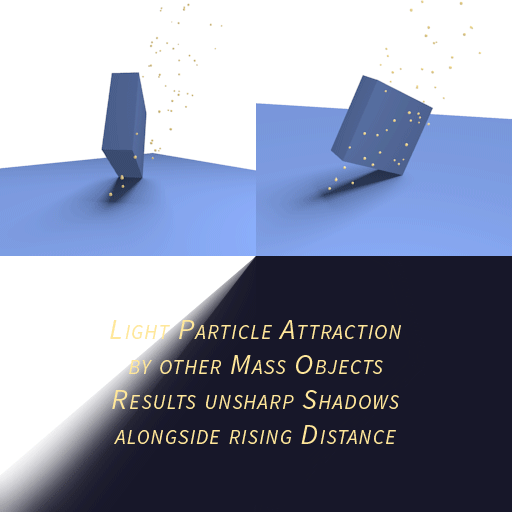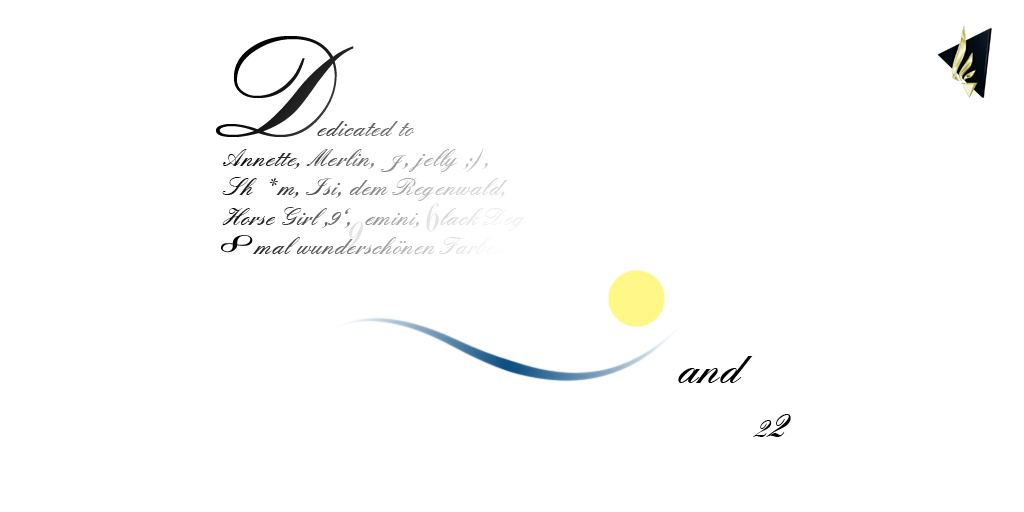Air and Light !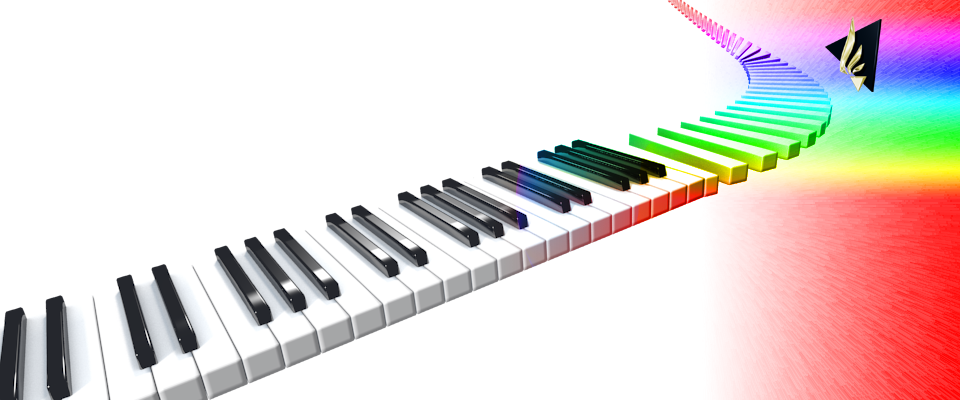Dedication

This piece of research work is dedicated especially to all of the former ,
current and future Members of The Royal Swedish Academy Of Sciencesand to my friends ( animals like men ) and parentsSee my site for more